### Maserati LogoHover over the thumbnail for a full-size version.

Author RavenMac author:ravenmac cool n-art rated 2008-07-07 2008-07-07 5 by 33 people. \$Maserati Logo#RavenMac#none#00000000000000000000000000000000000000000000000000000000000000000000000000000000000000000000000000000000000000000000000000000000000000000000000000000000000000000000000000000000000000000000000000000000000000000000000000000000000000000000000000000000000000000000000000000000000000000000000000000000000000000000000000000000000000000000000000000000000000000000000000000000000000000000000000000000000000000000000000000000000000000000000000000000000000000000000000000000000000000000000000000000000000000000000000000000000000000000000000000000000000000000000000000000000000000000000000000000000000000000000000000000000000000000000000000000000000000000000000000000000000000000000000000000000000000000000000000000000000000|8^378,443,1!1^379,442!1^380,443!8^380,443,1!1^381,442!1^382,443!8^382,443,1!1^383,442!1^384,443!8^384,443,1!1^385,442!1^386,443!8^386,443,1!1^387,442!1^388,443!8^388,443,1!1^389,442!1^390,443!8^390,443,1!1^391,442!1^392,443!8^366,441,1!1^367,440!1^368,441!8^368,441,1!1^369,440!1^370,441!8^370,441,1!1^371,440!1^372,441!8^372,441,1!1^373,440!1^374,441!8^374,441,1!1^375,440!1^376,441!8^376,441,1!1^377,440!1^378,441!8^378,441,1!1^379,440!1^380,441!8^380,441,1!1^381,440!1^382,441!8^382,441,1!1^383,440!1^384,441!8^384,441,1!1^385,440!1^386,441!8^386,441,1!1^387,440!1^388,441!8^388,441,1!1^389,440!1^390,441!8^390,441,1!1^391,440!1^392,441!8^392,441,1!1^393,440!1^394,441!8^394,441,1!1^395,440!1^396,441!8^396,441,1!1^397,440!1^398,441!8^398,441,1!1^399,440!1^400,441!8^400,441,1!1^401,440!1^402,441!8^360,439,1!1^361,438!1^362,439!8^362,439,1!1^363,438!1^364,439!8^364,439,1!1^365,438!1^366,439!8^366,439,1!1^367,438!1^368,439!8^368,439,1!1^369,438!1^370,439!8^398,439,1!1^399,438!1^400,439!8^400,439,1!1^401,438!1^402,439!8^402,439,1!1^403,438!1^404,439!8^404,439,1!1^405,438!1^406,439!8^406,439,1!1^407,438!1^408,439!8^356,437,1!1^357,436!1^358,437!8^358,437,1!1^359,436!1^360,437!8^360,437,1!1^361,436!1^362,437!8^362,437,1!1^363,436!1^364,437!8^406,437,1!1^407,436!1^408,437!8^408,437,1!1^409,436!1^410,437!8^410,437,1!1^411,436!1^412,437!8^412,437,1!1^413,436!1^414,437!8^352,435,1!1^353,434!1^354,435!8^354,435,1!1^355,434!1^356,435!8^356,435,1!1^357,434!1^358,435!8^410,435,1!1^411,434!1^412,435!8^412,435,1!1^413,434!1^414,435!8^414,435,1!1^415,434!1^416,435!8^416,435,1!1^417,434!1^418,435!8^348,433,1!1^349,432!1^350,433!8^350,433,1!1^351,432!1^352,433!8^352,433,1!1^353,432!1^354,433!8^372,433,1!1^373,432!1^374,433!8^374,433,1!1^375,432!1^376,433!8^376,433,1!1^377,432!1^378,433!8^378,433,1!1^379,432!1^380,433!8^380,433,1!1^381,432!1^382,433!8^382,433,1!1^383,432!1^384,433!8^384,433,1!1^385,432!1^386,433!8^386,433,1!1^387,432!1^388,433!8^388,433,1!1^389,432!1^390,433!8^390,433,1!1^391,432!1^392,433!8^392,433,1!1^393,432!1^394,433!8^394,433,1!1^395,432!1^396,433!8^396,433,1!1^397,432!1^398,433!8^414,433,1!1^415,432!1^416,433!8^416,433,1!1^417,432!1^418,433!8^418,433,1!1^419,432!1^420,433!8^346,431,1!1^347,430!1^348,431!8^348,431,1!1^349,430!1^350,431!8^364,431,1!1^365,430!1^366,431!8^366,431,1!1^367,430!1^368,431!8^368,431,1!1^369,430!1^370,431!8^370,431,1!1^371,430!1^372,431!8^372,431,1!1^373,430!1^374,431!8^374,431,1!1^375,430!1^376,431!8^376,431,1!1^377,430!1^378,431!8^378,431,1!1^379,430!1^380,431!8^380,431,1!1^381,430!1^382,431!8^382,431,1!1^383,430!1^384,431!8^384,431,1!1^385,430!1^386,431!8^386,431,1!1^387,430!1^388,431!8^388,431,1!1^389,430!1^390,431!8^390,431,1!1^391,430!1^392,431!8^392,431,1!1^393,430!1^394,431!8^394,431,1!1^395,430!1^396,431!8^396,431,1!1^397,430!1^398,431!8^398,431,1!1^399,430!1^400,431!8^400,431,1!1^401,430!1^402,431!8^402,431,1!1^403,430!1^404,431!8^418,431,1!1^419,430!1^420,431!8^420,431,1!1^421,430!1^422,431!8^422,431,1!1^423,430!1^424,431!8^342,429,1!1^343,428!1^344,429!8^344,429,1!1^345,428!1^346,429!8^346,429,1!1^347,428!1^348,429!8^360,429,1!1^361,428!1^362,429!8^362,429,1!1^363,428!1^364,429!8^364,429,1!1^365,428!1^366,429!8^366,429,1!1^367,428!1^368,429!8^368,429,1!1^369,428!1^370,429!8^370,429,1!1^371,428!1^372,429!8^372,429,1!1^373,428!1^374,429!8^374,429,1!1^375,428!1^376,429!8^376,429,1!1^377,428!1^378,429!8^378,429,1!1^379,428!1^380,429!8^380,429,1!1^381,428!1^382,429!8^382,429,1!1^383,428!1^384,429!8^384,429,1!1^385,428!1^386,429!8^386,429,1!1^387,428!1^388,429!8^388,429,1!1^389,428!1^390,429!8^390,429,1!1^391,428!1^392,429!8^392,429,1!1^393,428!1^394,429!8^394,429,1!1^395,428!1^396,429!8^396,429,1!1^397,428!1^398,429!8^398,429,1!1^399,428!1^400,429!8^400,429,1!1^401,428!1^402,429!8^402,429,1!1^403,428!1^404,429!8^404,429,1!1^405,428!1^406,429!8^406,429,1!1^407,428!1^408,429!8^408,429,1!1^409,428!1^410,429!8^422,429,1!1^423,428!1^424,429!8^424,429,1!1^425,428!1^426,429!8^340,427,1!1^341,426!1^342,427!8^342,427,1!1^343,426!1^344,427!8^356,427,1!1^357,426!1^358,427!8^358,427,1!1^359,426!1^360,427!8^360,427,1!1^361,426!1^362,427!8^362,427,1!1^363,426!1^364,427!8^364,427,1!1^365,426!1^366,427!8^366,427,1!1^367,426!1^368,427!8^368,427,1!1^369,426!1^370,427!8^370,427,1!1^371,426!1^372,427!8^372,427,1!1^373,426!1^374,427!8^374,427,1!1^375,426!1^376,427!8^376,427,1!1^377,426!1^378,427!8^378,427,1!1^379,426!1^380,427!8^380,427,1!1^381,426!1^382,427!8^382,427,1!1^383,426!1^384,427!8^384,427,1!1^385,426!1^386,427!8^386,427,1!1^387,426!1^388,427!8^388,427,1!1^389,426!1^390,427!8^390,427,1!1^391,426!1^392,427!8^392,427,1!1^393,426!1^394,427!8^394,427,1!1^395,426!1^396,427!8^396,427,1!1^397,426!1^398,427!8^398,427,1!1^399,426!1^400,427!8^400,427,1!1^401,426!1^402,427!8^402,427,1!1^403,426!1^404,427!8^404,427,1!1^405,426!1^406,427!8^406,427,1!1^407,426!1^408,427!8^408,427,1!1^409,426!1^410,427!8^410,427,1!1^411,426!1^412,427!8^412,427,1!1^413,426!1^414,427!8^424,427,1!1^425,426!1^426,427!8^426,427,1!1^427,426!1^428,427!8^428,427,1!1^429,426!1^430,427!8^338,425,1!1^339,424!1^340,425!8^340,425,1!1^341,424!1^342,425!8^352,425,1!1^353,424!1^354,425!8^354,425,1!1^355,424!1^356,425!8^356,425,1!1^357,424!1^358,425!8^358,425,1!1^359,424!1^360,425!8^360,425,1!1^361,424!1^362,425!8^362,425,1!1^363,424!1^364,425!8^364,425,1!1^365,424!1^366,425!8^366,425,1!1^367,424!1^368,425!8^368,425,1!1^369,424!1^370,425!8^370,425,1!1^371,424!1^372,425!8^372,425,1!1^373,424!1^374,425!8^374,425,1!1^375,424!1^376,425!8^376,425,1!1^377,424!1^378,425!8^378,425,1!1^379,424!1^380,425!8^380,425,1!1^381,424!1^382,425!8^382,425,1!1^383,424!1^384,425!8^384,425,1!1^385,424!1^386,425!8^386,425,1!1^387,424!1^388,425!8^388,425,1!1^389,424!1^390,425!8^390,425,1!1^391,424!1^392,425!8^392,425,1!1^393,424!1^394,425!8^394,425,1!1^395,424!1^396,425!8^396,425,1!1^397,424!1^398,425!8^398,425,1!1^399,424!1^400,425!8^400,425,1!1^401,424!1^402,425!8^402,425,1!1^403,424!1^404,425!8^404,425,1!1^405,424!1^406,425!8^406,425,1!1^407,424!1^408,425!8^408,425,1!1^409,424!1^410,425!8^410,425,1!1^411,424!1^412,425!8^412,425,1!1^413,424!1^414,425!8^414,425,1!1^415,424!1^416,425!8^428,425,1!1^429,424!1^430,425!8^430,425,1!1^431,424!1^432,425!8^336,423,1!1^337,422!1^338,423!8^338,423,1!1^339,422!1^340,423!8^350,423,1!1^351,422!1^352,423!8^352,423,1!1^353,422!1^354,423!8^354,423,1!1^355,422!1^356,423!8^356,423,1!1^357,422!1^358,423!8^358,423,1!1^359,422!1^360,423!8^360,423,1!1^361,422!1^362,423!8^362,423,1!1^363,422!1^364,423!8^364,423,1!1^365,422!1^366,423!8^366,423,1!1^367,422!1^368,423!8^368,423,1!1^369,422!1^370,423!8^370,423,1!1^371,422!1^372,423!8^372,423,1!1^373,422!1^374,423!8^374,423,1!1^375,422!1^376,423!8^376,423,1!1^377,422!1^378,423!8^378,423,1!1^379,422!1^380,423!8^380,423,1!1^381,422!1^382,423!8^382,423,1!1^383,422!1^384,423!8^384,423,1!1^385,422!1^386,423!8^386,423,1!1^387,422!1^388,423!8^388,423,1!1^389,422!1^390,423!8^390,423,1!1^391,422!1^392,423!8^392,423,1!1^393,422!1^394,423!8^394,423,1!1^395,422!1^396,423!8^396,423,1!1^397,422!1^398,423!8^398,423,1!1^399,422!1^400,423!8^400,423,1!1^401,422!1^402,423!8^402,423,1!1^403,422!1^404,423!8^404,423,1!1^405,422!1^406,423!8^406,423,1!1^407,422!1^408,423!8^408,423,1!1^409,422!1^410,423!8^410,423,1!1^411,422!1^412,423!8^412,423,1!1^413,422!1^414,423!8^414,423,1!1^415,422!1^416,423!8^416,423,1!1^417,422!1^418,423!8^418,423,1!1^419,422!1^420,423!8^430,423,1!1^431,422!1^432,423!8^432,423,1!1^433,422!1^434,423!8^334,421,1!1^335,420!1^336,421!8^336,421,1!1^337,420!1^338,421!8^346,421,1!1^347,420!1^348,421!8^348,421,1!1^349,420!1^350,421!8^350,421,1!1^351,420!1^352,421!8^352,421,1!1^353,420!1^354,421!8^354,421,1!1^355,420!1^356,421!8^356,421,1!1^357,420!1^358,421!8^358,421,1!1^359,420!1^360,421!8^360,421,1!1^361,420!1^362,421!8^362,421,1!1^363,420!1^364,421!8^364,421,1!1^365,420!1^366,421!8^366,421,1!1^367,420!1^368,421!8^368,421,1!1^369,420!1^370,421!8^370,421,1!1^371,420!1^372,421!8^372,421,1!1^373,420!1^374,421!8^374,421,1!1^375,420!1^376,421!8^376,421,1!1^377,420!1^378,421!8^378,421,1!1^379,420!1^380,421!8^380,421,1!1^381,420!1^382,421!8^382,421,1!1^383,420!1^384,421!8^384,421,1!1^385,420!1^386,421!8^386,421,1!1^387,420!1^388,421!8^388,421,1!1^389,420!1^390,421!8^390,421,1!1^391,420!1^392,421!8^392,421,1!1^393,420!1^394,421!8^394,421,1!1^395,420!1^396,421!8^396,421,1!1^397,420!1^398,421!8^398,421,1!1^399,420!1^400,421!8^400,421,1!1^401,420!1^402,421!8^402,421,1!1^403,420!1^404,421!8^404,421,1!1^405,420!1^406,421!8^406,421,1!1^407,420!1^408,421!8^408,421,1!1^409,420!1^410,421!8^410,421,1!1^411,420!1^412,421!8^412,421,1!1^413,420!1^414,421!8^414,421,1!1^415,420!1^416,421!8^416,421,1!1^417,420!1^418,421!8^418,421,1!1^419,420!1^420,421!8^420,421,1!1^421,420!1^422,421!8^432,421,1!1^433,420!1^434,421!8^434,421,1!1^435,420!1^436,421!8^332,419,1!1^333,418!1^334,419!8^334,419,1!1^335,418!1^336,419!8^344,419,1!1^345,418!1^346,419!8^346,419,1!1^347,418!1^348,419!8^348,419,1!1^349,418!1^350,419!8^350,419,1!1^351,418!1^352,419!8^352,419,1!1^353,418!1^354,419!8^354,419,1!1^355,418!1^356,419!8^356,419,1!1^357,418!1^358,419!8^358,419,1!1^359,418!1^360,419!8^360,419,1!1^361,418!1^362,419!8^362,419,1!1^363,418!1^364,419!8^364,419,1!1^365,418!1^366,419!8^366,419,1!1^367,418!1^368,419!8^368,419,1!1^369,418!1^370,419!8^370,419,1!1^371,418!1^372,419!8^372,419,1!1^373,418!1^374,419!8^374,419,1!1^375,418!1^376,419!8^376,419,1!1^377,418!1^378,419!8^378,419,1!1^379,418!1^380,419!8^380,419,1!1^381,418!1^382,419!8^382,419,1!1^383,418!1^384,419!8^384,419,1!1^385,418!1^386,419!8^386,419,1!1^387,418!1^388,419!8^388,419,1!1^389,418!1^390,419!8^390,419,1!1^391,418!1^392,419!8^392,419,1!1^393,418!1^394,419!8^394,419,1!1^395,418!1^396,419!8^396,419,1!1^397,418!1^398,419!8^398,419,1!1^399,418!1^400,419!8^400,419,1!1^401,418!1^402,419!8^402,419,1!1^403,418!1^404,419!8^404,419,1!1^405,418!1^406,419!8^406,419,1!1^407,418!1^408,419!8^408,419,1!1^409,418!1^410,419!8^410,419,1!1^411,418!1^412,419!8^412,419,1!1^413,418!1^414,419!8^414,419,1!1^415,418!1^416,419!8^416,419,1!1^417,418!1^418,419!8^418,419,1!1^419,418!1^420,419!8^420,419,1!1^421,418!1^422,419!8^422,419,1!1^423,418!1^424,419!8^424,419,1!1^425,418!1^426,419!8^434,419,1!1^435,418!1^436,419!8^436,419,1!1^437,418!1^438,419!8^330,417,1!1^331,416!1^332,417!8^332,417,1!1^333,416!1^334,417!8^342,417,1!1^343,416!1^344,417!8^344,417,1!1^345,416!1^346,417!8^346,417,1!1^347,416!1^348,417!8^348,417,1!1^349,416!1^350,417!8^350,417,1!1^351,416!1^352,417!8^352,417,1!1^353,416!1^354,417!8^354,417,1!1^355,416!1^356,417!8^356,417,1!1^357,416!1^358,417!8^358,417,1!1^359,416!1^360,417!8^360,417,1!1^361,416!1^362,417!8^362,417,1!1^363,416!1^364,417!8^364,417,1!1^365,416!1^366,417!8^366,417,1!1^367,416!1^368,417!8^368,417,1!1^369,416!1^370,417!8^370,417,1!1^371,416!1^372,417!8^372,417,1!1^373,416!1^374,417!8^374,417,1!1^375,416!1^376,417!8^376,417,1!1^377,416!1^378,417!8^378,417,1!1^379,416!1^380,417!8^380,417,1!1^381,416!1^382,417!8^382,417,1!1^383,416!1^384,417!8^384,417,1!1^385,416!1^386,417!8^386,417,1!1^387,416!1^388,417!8^388,417,1!1^389,416!1^390,417!8^390,417,1!1^391,416!1^392,417!8^392,417,1!1^393,416!1^394,417!8^394,417,1!1^395,416!1^396,417!8^396,417,1!1^397,416!1^398,417!8^398,417,1!1^399,416!1^400,417!8^400,417,1!1^401,416!1^402,417!8^402,417,1!1^403,416!1^404,417!8^404,417,1!1^405,416!1^406,417!8^406,417,1!1^407,416!1^408,417!8^408,417,1!1^409,416!1^410,417!8^410,417,1!1^411,416!1^412,417!8^412,417,1!1^413,416!1^414,417!8^414,417,1!1^415,416!1^416,417!8^416,417,1!1^417,416!1^418,417!8^418,417,1!1^419,416!1^420,417!8^420,417,1!1^421,416!1^422,417!8^422,417,1!1^423,416!1^424,417!8^424,417,1!1^425,416!1^426,417!8^426,417,1!1^427,416!1^428,417!8^436,417,1!1^437,416!1^438,417!8^438,417,1!1^439,416!1^440,417!8^328,415,1!1^329,414!1^330,415!8^330,415,1!1^331,414!1^332,415!8^340,415,1!1^341,414!1^342,415!8^342,415,1!1^343,414!1^344,415!8^344,415,1!1^345,414!1^346,415!8^346,415,1!1^347,414!1^348,415!8^348,415,1!1^349,414!1^350,415!8^350,415,1!1^351,414!1^352,415!8^352,415,1!1^353,414!1^354,415!8^354,415,1!1^355,414!1^356,415!8^356,415,1!1^357,414!1^358,415!8^358,415,1!1^359,414!1^360,415!8^360,415,1!1^361,414!1^362,415!8^362,415,1!1^363,414!1^364,415!8^364,415,1!1^365,414!1^366,415!8^366,415,1!1^367,414!1^368,415!8^368,415,1!1^369,414!1^370,415!8^370,415,1!1^371,414!1^372,415!8^372,415,1!1^373,414!1^374,415!8^374,415,1!1^375,414!1^376,415!8^376,415,1!1^377,414!1^378,415!8^378,415,1!1^379,414!1^380,415!8^380,415,1!1^381,414!1^382,415!8^382,415,1!1^383,414!1^384,415!8^384,415,1!1^385,414!1^386,415!8^386,415,1!1^387,414!1^388,415!8^388,415,1!1^389,414!1^390,415!8^390,415,1!1^391,414!1^392,415!8^392,415,1!1^393,414!1^394,415!8^394,415,1!1^395,414!1^396,415!8^396,415,1!1^397,414!1^398,415!8^398,415,1!1^399,414!1^400,415!8^400,415,1!1^401,414!1^402,415!8^402,415,1!1^403,414!1^404,415!8^404,415,1!1^405,414!1^406,415!8^406,415,1!1^407,414!1^408,415!8^408,415,1!1^409,414!1^410,415!8^410,415,1!1^411,414!1^412,415!8^412,415,1!1^413,414!1^414,415!8^414,415,1!1^415,414!1^416,415!8^416,415,1!1^417,414!1^418,415!8^418,415,1!1^419,414!1^420,415!8^420,415,1!1^421,414!1^422,415!8^422,415,1!1^423,414!1^424,415!8^424,415,1!1^425,414!1^426,415!8^426,415,1!1^427,414!1^428,415!8^428,415,1!1^429,414!1^430,415!8^438,415,1!1^439,414!1^440,415!8^440,415,1!1^441,414!1^442,415!8^326,413,1!1^327,412!1^328,413!8^328,413,1!1^329,412!1^330,413!8^338,413,1!1^339,412!1^340,413!8^340,413,1!1^341,412!1^342,413!8^342,413,1!1^343,412!1^344,413!8^344,413,1!1^345,412!1^346,413!8^346,413,1!1^347,412!1^348,413!8^348,413,1!1^349,412!1^350,413!8^350,413,1!1^351,412!1^352,413!8^352,413,1!1^353,412!1^354,413!8^354,413,1!1^355,412!1^356,413!8^356,413,1!1^357,412!1^358,413!8^358,413,1!1^359,412!1^360,413!8^360,413,1!1^361,412!1^362,413!8^362,413,1!1^363,412!1^364,413!8^364,413,1!1^365,412!1^366,413!8^366,413,1!1^367,412!1^368,413!8^368,413,1!1^369,412!1^370,413!8^370,413,1!1^371,412!1^372,413!8^372,413,1!1^373,412!1^374,413!8^374,413,1!1^375,412!1^376,413!8^376,413,1!1^377,412!1^378,413!8^378,413,1!1^379,412!1^380,413!8^380,413,1!1^381,412!1^382,413!8^382,413,1!1^383,412!1^384,413!8^384,413,1!1^385,412!1^386,413!8^386,413,1!1^387,412!1^388,413!8^388,413,1!1^389,412!1^390,413!8^390,413,1!1^391,412!1^392,413!8^392,413,1!1^393,412!1^394,413!8^394,413,1!1^395,412!1^396,413!8^396,413,1!1^397,412!1^398,413!8^398,413,1!1^399,412!1^400,413!8^400,413,1!1^401,412!1^402,413!8^402,413,1!1^403,412!1^404,413!8^404,413,1!1^405,412!1^406,413!8^406,413,1!1^407,412!1^408,413!8^408,413,1!1^409,412!1^410,413!8^410,413,1!1^411,412!1^412,413!8^412,413,1!1^413,412!1^414,413!8^414,413,1!1^415,412!1^416,413!8^416,413,1!1^417,412!1^418,413!8^418,413,1!1^419,412!1^420,413!8^420,413,1!1^421,412!1^422,413!8^422,413,1!1^423,412!1^424,413!8^424,413,1!1^425,412!1^426,413!8^426,413,1!1^427,412!1^428,413!8^428,413,1!1^429,412!1^430,413!8^430,413,1!1^431,412!1^432,413!8^440,413,1!1^441,412!1^442,413!8^442,413,1!1^443,412!1^444,413!8^324,411,1!1^325,410!1^326,411!8^326,411,1!1^327,410!1^328,411!8^336,411,1!1^337,410!1^338,411!8^338,411,1!1^339,410!1^340,411!8^340,411,1!1^341,410!1^342,411!8^342,411,1!1^343,410!1^344,411!8^344,411,1!1^345,410!1^346,411!8^346,411,1!1^347,410!1^348,411!8^348,411,1!1^349,410!1^350,411!8^350,411,1!1^351,410!1^352,411!8^352,411,1!1^353,410!1^354,411!8^354,411,1!1^355,410!1^356,411!8^356,411,1!1^357,410!1^358,411!8^358,411,1!1^359,410!1^360,411!8^360,411,1!1^361,410!1^362,411!8^362,411,1!1^363,410!1^364,411!8^364,411,1!1^365,410!1^366,411!8^366,411,1!1^367,410!1^368,411!8^368,411,1!1^369,410!1^370,411!8^370,411,1!1^371,410!1^372,411!8^372,411,1!1^373,410!1^374,411!8^374,411,1!1^375,410!1^376,411!8^376,411,1!1^377,410!1^378,411!8^378,411,1!1^379,410!1^380,411!8^380,411,1!1^381,410!1^382,411!8^382,411,1!1^383,410!1^384,411!8^384,411,1!1^385,410!1^386,411!8^386,411,1!1^387,410!1^388,411!8^388,411,1!1^389,410!1^390,411!8^390,411,1!1^391,410!1^392,411!8^392,411,1!1^393,410!1^394,411!8^394,411,1!1^395,410!1^396,411!8^396,411,1!1^397,410!1^398,411!8^398,411,1!1^399,410!1^400,411!8^400,411,1!1^401,410!1^402,411!8^402,411,1!1^403,410!1^404,411!8^404,411,1!1^405,410!1^406,411!8^406,411,1!1^407,410!1^408,411!8^408,411,1!1^409,410!1^410,411!8^410,411,1!1^411,410!1^412,411!8^412,411,1!1^413,410!1^414,411!8^414,411,1!1^415,410!1^416,411!8^416,411,1!1^417,410!1^418,411!8^418,411,1!1^419,410!1^420,411!8^420,411,1!1^421,410!1^422,411!8^422,411,1!1^423,410!1^424,411!8^424,411,1!1^425,410!1^426,411!8^426,411,1!1^427,410!1^428,411!8^428,411,1!1^429,410!1^430,411!8^430,411,1!1^431,410!1^432,411!8^432,411,1!1^433,410!1^434,411!8^442,411,1!1^443,410!1^444,411!8^444,411,1!1^445,410!1^446,411!8^322,409,1!1^323,408!1^324,409!8^324,409,1!1^325,408!1^326,409!8^334,409,1!1^335,408!1^336,409!8^336,409,1!1^337,408!1^338,409!8^338,409,1!1^339,408!1^340,409!8^340,409,1!1^341,408!1^342,409!8^342,409,1!1^343,408!1^344,409!8^344,409,1!1^345,408!1^346,409!8^346,409,1!1^347,408!1^348,409!8^348,409,1!1^349,408!1^350,409!8^350,409,1!1^351,408!1^352,409!8^352,409,1!1^353,408!1^354,409!8^354,409,1!1^355,408!1^356,409!8^356,409,1!1^357,408!1^358,409!8^358,409,1!1^359,408!1^360,409!8^360,409,1!1^361,408!1^362,409!8^362,409,1!1^363,408!1^364,409!8^364,409,1!1^365,408!1^366,409!8^366,409,1!1^367,408!1^368,409!8^368,409,1!1^369,408!1^370,409!8^370,409,1!1^371,408!1^372,409!8^372,409,1!1^373,408!1^374,409!8^374,409,1!1^375,408!1^376,409!8^376,409,1!1^377,408!1^378,409!8^378,409,1!1^379,408!1^380,409!8^380,409,1!1^381,408!1^382,409!8^382,409,1!1^383,408!1^384,409!8^384,409,1!1^385,408!1^386,409!8^386,409,1!1^387,408!1^388,409!8^388,409,1!1^389,408!1^390,409!8^390,409,1!1^391,408!1^392,409!8^392,409,1!1^393,408!1^394,409!8^394,409,1!1^395,408!1^396,409!8^396,409,1!1^397,408!1^398,409!8^398,409,1!1^399,408!1^400,409!8^400,409,1!1^401,408!1^402,409!8^402,409,1!1^403,408!1^404,409!8^404,409,1!1^405,408!1^406,409!8^406,409,1!1^407,408!1^408,409!8^408,409,1!1^409,408!1^410,409!8^410,409,1!1^411,408!1^412,409!8^412,409,1!1^413,408!1^414,409!8^414,409,1!1^415,408!1^416,409!8^416,409,1!1^417,408!1^418,409!8^418,409,1!1^419,408!1^420,409!8^420,409,1!1^421,408!1^422,409!8^422,409,1!1^423,408!1^424,409!8^424,409,1!1^425,408!1^426,409!8^426,409,1!1^427,408!1^428,409!8^428,409,1!1^429,408!1^430,409!8^430,409,1!1^431,408!1^432,409!8^432,409,1!1^433,408!1^434,409!8^434,409,1!1^435,408!1^436,409!8^444,409,1!1^445,408!1^446,409!8^446,409,1!1^447,408!1^448,409!8^320,407,1!1^321,406!1^322,407!8^322,407,1!1^323,406!1^324,407!8^332,407,1!1^333,406!1^334,407!8^334,407,1!1^335,406!1^336,407!8^336,407,1!1^337,406!1^338,407!8^338,407,1!1^339,406!1^340,407!8^340,407,1!1^341,406!1^342,407!8^342,407,1!1^343,406!1^344,407!8^344,407,1!1^345,406!1^346,407!8^346,407,1!1^347,406!1^348,407!8^348,407,1!1^349,406!1^350,407!8^350,407,1!1^351,406!1^352,407!8^352,407,1!1^353,406!1^354,407!8^354,407,1!1^355,406!1^356,407!8^356,407,1!1^357,406!1^358,407!8^358,407,1!1^359,406!1^360,407!8^360,407,1!1^361,406!1^362,407!8^362,407,1!1^363,406!1^364,407!8^364,407,1!1^365,406!1^366,407!8^366,407,1!1^367,406!1^368,407!8^368,407,1!1^369,406!1^370,407!8^370,407,1!1^371,406!1^372,407!8^372,407,1!1^373,406!1^374,407!8^374,407,1!1^375,406!1^376,407!8^376,407,1!1^377,406!1^378,407!8^378,407,1!1^379,406!1^380,407!8^380,407,1!1^381,406!1^382,407!8^382,407,1!1^383,406!1^384,407!8^384,407,1!1^385,406!1^386,407!8^386,407,1!1^387,406!1^388,407!8^388,407,1!1^389,406!1^390,407!8^390,407,1!1^391,406!1^392,407!8^392,407,1!1^393,406!1^394,407!8^394,407,1!1^395,406!1^396,407!8^396,407,1!1^397,406!1^398,407!8^398,407,1!1^399,406!1^400,407!8^400,407,1!1^401,406!1^402,407!8^402,407,1!1^403,406!1^404,407!8^404,407,1!1^405,406!1^406,407!8^406,407,1!1^407,406!1^408,407!8^408,407,1!1^409,406!1^410,407!8^410,407,1!1^411,406!1^412,407!8^412,407,1!1^413,406!1^414,407!8^414,407,1!1^415,406!1^416,407!8^416,407,1!1^417,406!1^418,407!8^418,407,1!1^419,406!1^420,407!8^420,407,1!1^421,406!1^422,407!8^422,407,1!1^423,406!1^424,407!8^424,407,1!1^425,406!1^426,407!8^426,407,1!1^427,406!1^428,407!8^428,407,1!1^429,406!1^430,407!8^430,407,1!1^431,406!1^432,407!8^432,407,1!1^433,406!1^434,407!8^434,407,1!1^435,406!1^436,407!8^436,407,1!1^437,406!1^438,407!8^446,407,1!1^447,406!1^448,407!8^318,405,1!1^319,404!1^320,405!8^320,405,1!1^321,404!1^322,405!8^330,405,1!1^331,404!1^332,405!8^332,405,1!1^333,404!1^334,405!8^334,405,1!1^335,404!1^336,405!8^336,405,1!1^337,404!1^338,405!8^338,405,1!1^339,404!1^340,405!8^340,405,1!1^341,404!1^342,405!8^342,405,1!1^343,404!1^344,405!8^344,405,1!1^345,404!1^346,405!8^346,405,1!1^347,404!1^348,405!8^348,405,1!1^349,404!1^350,405!8^350,405,1!1^351,404!1^352,405!8^352,405,1!1^353,404!1^354,405!8^354,405,1!1^355,404!1^356,405!8^356,405,1!1^357,404!1^358,405!8^358,405,1!1^359,404!1^360,405!8^360,405,1!1^361,404!1^362,405!8^362,405,1!1^363,404!1^364,405!8^364,405,1!1^365,404!1^366,405!8^366,405,1!1^367,404!1^368,405!8^368,405,1!1^369,404!1^370,405!8^370,405,1!1^371,404!1^372,405!8^372,405,1!1^373,404!1^374,405!8^374,405,1!1^375,404!1^376,405!8^376,405,1!1^377,404!1^378,405!8^378,405,1!1^379,404!1^380,405!8^380,405,1!1^381,404!1^382,405!8^382,405,1!1^383,404!1^384,405!8^384,405,1!1^385,404!1^386,405!8^386,405,1!1^387,404!1^388,405!8^388,405,1!1^389,404!1^390,405!8^390,405,1!1^391,404!1^392,405!8^392,405,1!1^393,404!1^394,405!8^394,405,1!1^395,404!1^396,405!8^396,405,1!1^397,404!1^398,405!8^398,405,1!1^399,404!1^400,405!8^400,405,1!1^401,404!1^402,405!8^402,405,1!1^403,404!1^404,405!8^404,405,1!1^405,404!1^406,405!8^406,405,1!1^407,404!1^408,405!8^408,405,1!1^409,404!1^410,405!8^410,405,1!1^411,404!1^412,405!8^412,405,1!1^413,404!1^414,405!8^414,405,1!1^415,404!1^416,405!8^416,405,1!1^417,404!1^418,405!8^418,405,1!1^419,404!1^420,405!8^420,405,1!1^421,404!1^422,405!8^422,405,1!1^423,404!1^424,405!8^424,405,1!1^425,404!1^426,405!8^426,405,1!1^427,404!1^428,405!8^428,405,1!1^429,404!1^430,405!8^430,405,1!1^431,404!1^432,405!8^432,405,1!1^433,404!1^434,405!8^434,405,1!1^435,404!1^436,405!8^436,405,1!1^437,404!1^438,405!8^438,405,1!1^439,404!1^440,405!8^446,405,1!1^447,404!1^448,405!8^448,405,1!1^449,404!1^450,405!8^318,403,1!1^319,402!1^320,403!8^320,403,1!1^321,402!1^322,403!8^328,403,1!1^329,402!1^330,403!8^330,403,1!1^331,402!1^332,403!8^332,403,1!1^333,402!1^334,403!8^334,403,1!1^335,402!1^336,403!8^336,403,1!1^337,402!1^338,403!8^338,403,1!1^339,402!1^340,403!8^340,403,1!1^341,402!1^342,403!8^342,403,1!1^343,402!1^344,403!8^344,403,1!1^345,402!1^346,403!8^346,403,1!1^347,402!1^348,403!8^348,403,1!1^349,402!1^350,403!8^350,403,1!1^351,402!1^352,403!8^352,403,1!1^353,402!1^354,403!8^354,403,1!1^355,402!1^356,403!8^356,403,1!1^357,402!1^358,403!8^358,403,1!1^359,402!1^360,403!8^360,403,1!1^361,402!1^362,403!8^362,403,1!1^363,402!1^364,403!8^364,403,1!1^365,402!1^366,403!8^366,403,1!1^367,402!1^368,403!8^368,403,1!1^369,402!1^370,403!8^370,403,1!1^371,402!1^372,403!8^372,403,1!1^373,402!1^374,403!8^374,403,1!1^375,402!1^376,403!8^376,403,1!1^377,402!1^378,403!8^378,403,1!1^379,402!1^380,403!8^380,403,1!1^381,402!1^382,403!8^382,403,1!1^383,402!1^384,403!8^384,403,1!1^385,402!1^386,403!8^386,403,1!1^387,402!1^388,403!8^388,403,1!1^389,402!1^390,403!8^390,403,1!1^391,402!1^392,403!8^392,403,1!1^393,402!1^394,403!8^394,403,1!1^395,402!1^396,403!8^396,403,1!1^397,402!1^398,403!8^398,403,1!1^399,402!1^400,403!8^400,403,1!1^401,402!1^402,403!8^402,403,1!1^403,402!1^404,403!8^404,403,1!1^405,402!1^406,403!8^406,403,1!1^407,402!1^408,403!8^408,403,1!1^409,402!1^410,403!8^410,403,1!1^411,402!1^412,403!8^412,403,1!1^413,402!1^414,403!8^414,403,1!1^415,402!1^416,403!8^416,403,1!1^417,402!1^418,403!8^418,403,1!1^419,402!1^420,403!8^420,403,1!1^421,402!1^422,403!8^422,403,1!1^423,402!1^424,403!8^424,403,1!1^425,402!1^426,403!8^426,403,1!1^427,402!1^428,403!8^428,403,1!1^429,402!1^430,403!8^430,403,1!1^431,402!1^432,403!8^432,403,1!1^433,402!1^434,403!8^434,403,1!1^435,402!1^436,403!8^436,403,1!1^437,402!1^438,403!8^438,403,1!1^439,402!1^440,403!8^440,403,1!1^441,402!1^442,403!8^448,403,1!1^449,402!1^450,403!8^450,403,1!1^451,402!1^452,403!8^316,401,1!1^317,400!1^318,401!8^318,401,1!1^319,400!1^320,401!8^328,401,1!1^329,400!1^330,401!8^330,401,1!1^331,400!1^332,401!8^332,401,1!1^333,400!1^334,401!8^334,401,1!1^335,400!1^336,401!8^336,401,1!1^337,400!1^338,401!8^338,401,1!1^339,400!1^340,401!8^340,401,1!1^341,400!1^342,401!8^342,401,1!1^343,400!1^344,401!8^344,401,1!1^345,400!1^346,401!8^346,401,1!1^347,400!1^348,401!8^348,401,1!1^349,400!1^350,401!8^350,401,1!1^351,400!1^352,401!8^352,401,1!1^353,400!1^354,401!8^354,401,1!1^355,400!1^356,401!8^356,401,1!1^357,400!1^358,401!8^358,401,1!1^359,400!1^360,401!8^360,401,1!1^361,400!1^362,401!8^362,401,1!1^363,400!1^364,401!8^364,401,1!1^365,400!1^366,401!8^366,401,1!1^367,400!1^368,401!8^368,401,1!1^369,400!1^370,401!8^370,401,1!1^371,400!1^372,401!8^372,401,1!1^373,400!1^374,401!8^374,401,1!1^375,400!1^376,401!8^376,401,1!1^377,400!1^378,401!8^378,401,1!1^379,400!1^380,401!8^380,401,1!1^381,400!1^382,401!8^382,401,1!1^383,400!1^384,401!8^384,401,1!1^385,400!1^386,401!8^386,401,1!1^387,400!1^388,401!8^388,401,1!1^389,400!1^390,401!8^390,401,1!1^391,400!1^392,401!8^392,401,1!1^393,400!1^394,401!8^394,401,1!1^395,400!1^396,401!8^396,401,1!1^397,400!1^398,401!8^398,401,1!1^399,400!1^400,401!8^400,401,1!1^401,400!1^402,401!8^402,401,1!1^403,400!1^404,401!8^404,401,1!1^405,400!1^406,401!8^406,401,1!1^407,400!1^408,401!8^408,401,1!1^409,400!1^410,401!8^410,401,1!1^411,400!1^412,401!8^412,401,1!1^413,400!1^414,401!8^414,401,1!1^415,400!1^416,401!8^416,401,1!1^417,400!1^418,401!8^418,401,1!1^419,400!1^420,401!8^420,401,1!1^421,400!1^422,401!8^422,401,1!1^423,400!1^424,401!8^424,401,1!1^425,400!1^426,401!8^426,401,1!1^427,400!1^428,401!8^428,401,1!1^429,400!1^430,401!8^430,401,1!1^431,400!1^432,401!8^432,401,1!1^433,400!1^434,401!8^434,401,1!1^435,400!1^436,401!8^436,401,1!1^437,400!1^438,401!8^438,401,1!1^439,400!1^440,401!8^440,401,1!1^441,400!1^442,401!8^450,401,1!1^451,400!1^452,401!8^452,401,1!1^453,400!1^454,401!8^314,399,1!1^315,398!1^316,399!8^316,399,1!1^317,398!1^318,399!8^326,399,1!1^327,398!1^328,399!8^328,399,1!1^329,398!1^330,399!8^330,399,1!1^331,398!1^332,399!8^332,399,1!1^333,398!1^334,399!8^334,399,1!1^335,398!1^336,399!8^336,399,1!1^337,398!1^338,399!8^338,399,1!1^339,398!1^340,399!8^340,399,1!1^341,398!1^342,399!8^342,399,1!1^343,398!1^344,399!8^344,399,1!1^345,398!1^346,399!8^346,399,1!1^347,398!1^348,399!8^348,399,1!1^349,398!1^350,399!8^350,399,1!1^351,398!1^352,399!8^352,399,1!1^353,398!1^354,399!8^354,399,1!1^355,398!1^356,399!8^356,399,1!1^357,398!1^358,399!8^358,399,1!1^359,398!1^360,399!8^360,399,1!1^361,398!1^362,399!8^362,399,1!1^363,398!1^364,399!8^364,399,1!1^365,398!1^366,399!8^366,399,1!1^367,398!1^368,399!8^368,399,1!1^369,398!1^370,399!8^370,399,1!1^371,398!1^372,399!8^372,399,1!1^373,398!1^374,399!8^374,399,1!1^375,398!1^376,399!8^376,399,1!1^377,398!1^378,399!8^378,399,1!1^379,398!1^380,399!8^380,399,1!1^381,398!1^382,399!8^382,399,1!1^383,398!1^384,399!8^384,399,1!1^385,398!1^386,399!8^386,399,1!1^387,398!1^388,399!8^388,399,1!1^389,398!1^390,399!8^390,399,1!1^391,398!1^392,399!8^392,399,1!1^393,398!1^394,399!8^394,399,1!1^395,398!1^396,399!8^396,399,1!1^397,398!1^398,399!8^398,399,1!1^399,398!1^400,399!8^400,399,1!1^401,398!1^402,399!8^402,399,1!1^403,398!1^404,399!8^404,399,1!1^405,398!1^406,399!8^406,399,1!1^407,398!1^408,399!8^408,399,1!1^409,398!1^410,399!8^410,399,1!1^411,398!1^412,399!8^412,399,1!1^413,398!1^414,399!8^414,399,1!1^415,398!1^416,399!8^416,399,1!1^417,398!1^418,399!8^418,399,1!1^419,398!1^420,399!8^420,399,1!1^421,398!1^422,399!8^422,399,1!1^423,398!1^424,399!8^424,399,1!1^425,398!1^426,399!8^426,399,1!1^427,398!1^428,399!8^428,399,1!1^429,398!1^430,399!8^430,399,1!1^431,398!1^432,399!8^432,399,1!1^433,398!1^434,399!8^434,399,1!1^435,398!1^436,399!8^436,399,1!1^437,398!1^438,399!8^438,399,1!1^439,398!1^440,399!8^440,399,1!1^441,398!1^442,399!8^442,399,1!1^443,398!1^444,399!8^450,399,1!1^451,398!1^452,399!8^452,399,1!1^453,398!1^454,399!8^314,397,1!1^315,396!1^316,397!8^316,397,1!1^317,396!1^318,397!8^324,397,1!1^325,396!1^326,397!8^326,397,1!1^327,396!1^328,397!8^328,397,1!1^329,396!1^330,397!8^330,397,1!1^331,396!1^332,397!8^332,397,1!1^333,396!1^334,397!8^334,397,1!1^335,396!1^336,397!8^336,397,1!1^337,396!1^338,397!8^338,397,1!1^339,396!1^340,397!8^340,397,1!1^341,396!1^342,397!8^342,397,1!1^343,396!1^344,397!8^344,397,1!1^345,396!1^346,397!8^346,397,1!1^347,396!1^348,397!8^348,397,1!1^349,396!1^350,397!8^350,397,1!1^351,396!1^352,397!8^352,397,1!1^353,396!1^354,397!8^354,397,1!1^355,396!1^356,397!8^356,397,1!1^357,396!1^358,397!8^358,397,1!1^359,396!1^360,397!8^360,397,1!1^361,396!1^362,397!8^362,397,1!1^363,396!1^364,397!8^364,397,1!1^365,396!1^366,397!8^366,397,1!1^367,396!1^368,397!8^368,397,1!1^369,396!1^370,397!8^370,397,1!1^371,396!1^372,397!8^372,397,1!1^373,396!1^374,397!8^374,397,1!1^375,396!1^376,397!8^376,397,1!1^377,396!1^378,397!8^378,397,1!1^379,396!1^380,397!8^380,397,1!1^381,396!1^382,397!8^382,397,1!1^383,396!1^384,397!8^384,397,1!1^385,396!1^386,397!8^386,397,1!1^387,396!1^388,397!8^388,397,1!1^389,396!1^390,397!8^390,397,1!1^391,396!1^392,397!8^392,397,1!1^393,396!1^394,397!8^394,397,1!1^395,396!1^396,397!8^396,397,1!1^397,396!1^398,397!8^398,397,1!1^399,396!1^400,397!8^400,397,1!1^401,396!1^402,397!8^402,397,1!1^403,396!1^404,397!8^404,397,1!1^405,396!1^406,397!8^406,397,1!1^407,396!1^408,397!8^408,397,1!1^409,396!1^410,397!8^410,397,1!1^411,396!1^412,397!8^412,397,1!1^413,396!1^414,397!8^414,397,1!1^415,396!1^416,397!8^416,397,1!1^417,396!1^418,397!8^418,397,1!1^419,396!1^420,397!8^420,397,1!1^421,396!1^422,397!8^422,397,1!1^423,396!1^424,397!8^424,397,1!1^425,396!1^426,397!8^426,397,1!1^427,396!1^428,397!8^428,397,1!1^429,396!1^430,397!8^430,397,1!1^431,396!1^432,397!8^432,397,1!1^433,396!1^434,397!8^434,397,1!1^435,396!1^436,397!8^436,397,1!1^437,396!1^438,397!8^438,397,1!1^439,396!1^440,397!8^440,397,1!1^441,396!1^442,397!8^442,397,1!1^443,396!1^444,397!8^444,397,1!1^445,396!1^446,397!8^452,397,1!1^453,396!1^454,397!8^454,397,1!1^455,396!1^456,397!8^312,395,1!1^313,394!1^314,395!8^314,395,1!1^315,394!1^316,395!8^322,395,1!1^323,394!1^324,395!8^324,395,1!1^325,394!1^326,395!8^326,395,1!1^327,394!1^328,395!8^328,395,1!1^329,394!1^330,395!8^330,395,1!1^331,394!1^332,395!8^332,395,1!1^333,394!1^334,395!8^334,395,1!1^335,394!1^336,395!8^336,395,1!1^337,394!1^338,395!8^338,395,1!1^339,394!1^340,395!8^340,395,1!1^341,394!1^342,395!8^342,395,1!1^343,394!1^344,395!8^344,395,1!1^345,394!1^346,395!8^346,395,1!1^347,394!1^348,395!8^348,395,1!1^349,394!1^350,395!8^350,395,1!1^351,394!1^352,395!8^352,395,1!1^353,394!1^354,395!8^354,395,1!1^355,394!1^356,395!8^356,395,1!1^357,394!1^358,395!8^358,395,1!1^359,394!1^360,395!8^360,395,1!1^361,394!1^362,395!8^362,395,1!1^363,394!1^364,395!8^364,395,1!1^365,394!1^366,395!8^366,395,1!1^367,394!1^368,395!8^368,395,1!1^369,394!1^370,395!8^370,395,1!1^371,394!1^372,395!8^372,395,1!1^373,394!1^374,395!8^374,395,1!1^375,394!1^376,395!8^376,395,1!1^377,394!1^378,395!8^378,395,1!1^379,394!1^380,395!8^380,395,1!1^381,394!1^382,395!8^382,395,1!1^383,394!1^384,395!8^384,395,1!1^385,394!1^386,395!8^386,395,1!1^387,394!1^388,395!8^388,395,1!1^389,394!1^390,395!8^390,395,1!1^391,394!1^392,395!8^392,395,1!1^393,394!1^394,395!8^394,395,1!1^395,394!1^396,395!8^396,395,1!1^397,394!1^398,395!8^398,395,1!1^399,394!1^400,395!8^400,395,1!1^401,394!1^402,395!8^402,395,1!1^403,394!1^404,395!8^404,395,1!1^405,394!1^406,395!8^406,395,1!1^407,394!1^408,395!8^408,395,1!1^409,394!1^410,395!8^410,395,1!1^411,394!1^412,395!8^412,395,1!1^413,394!1^414,395!8^414,395,1!1^415,394!1^416,395!8^416,395,1!1^417,394!1^418,395!8^418,395,1!1^419,394!1^420,395!8^420,395,1!1^421,394!1^422,395!8^422,395,1!1^423,394!1^424,395!8^424,395,1!1^425,394!1^426,395!8^426,395,1!1^427,394!1^428,395!8^428,395,1!1^429,394!1^430,395!8^430,395,1!1^431,394!1^432,395!8^432,395,1!1^433,394!1^434,395!8^434,395,1!1^435,394!1^436,395!8^436,395,1!1^437,394!1^438,395!8^438,395,1!1^439,394!1^440,395!8^440,395,1!1^441,394!1^442,395!8^442,395,1!1^443,394!1^444,395!8^444,395,1!1^445,394!1^446,395!8^454,395,1!1^455,394!1^456,395!8^312,393,1!1^313,392!1^314,393!8^322,393,1!1^323,392!1^324,393!8^324,393,1!1^325,392!1^326,393!8^326,393,1!1^327,392!1^328,393!8^328,393,1!1^329,392!1^330,393!8^330,393,1!1^331,392!1^332,393!8^332,393,1!1^333,392!1^334,393!8^334,393,1!1^335,392!1^336,393!8^336,393,1!1^337,392!1^338,393!8^338,393,1!1^339,392!1^340,393!8^340,393,1!1^341,392!1^342,393!8^342,393,1!1^343,392!1^344,393!8^344,393,1!1^345,392!1^346,393!8^346,393,1!1^347,392!1^348,393!8^348,393,1!1^349,392!1^350,393!8^350,393,1!1^351,392!1^352,393!8^352,393,1!1^353,392!1^354,393!8^354,393,1!1^355,392!1^356,393!8^356,393,1!1^357,392!1^358,393!8^358,393,1!1^359,392!1^360,393!8^360,393,1!1^361,392!1^362,393!8^362,393,1!1^363,392!1^364,393!8^364,393,1!1^365,392!1^366,393!8^366,393,1!1^367,392!1^368,393!8^368,393,1!1^369,392!1^370,393!8^370,393,1!1^371,392!1^372,393!8^372,393,1!1^373,392!1^374,393!8^374,393,1!1^375,392!1^376,393!8^376,393,1!1^377,392!1^378,393!8^378,393,1!1^379,392!1^380,393!8^380,393,1!1^381,392!1^382,393!8^382,393,1!1^383,392!1^384,393!8^384,393,1!1^385,392!1^386,393!8^386,393,1!1^387,392!1^388,393!8^388,393,1!1^389,392!1^390,393!8^390,393,1!1^391,392!1^392,393!8^392,393,1!1^393,392!1^394,393!8^394,393,1!1^395,392!1^396,393!8^396,393,1!1^397,392!1^398,393!8^398,393,1!1^399,392!1^400,393!8^400,393,1!1^401,392!1^402,393!8^402,393,1!1^403,392!1^404,393!8^404,393,1!1^405,392!1^406,393!8^406,393,1!1^407,392!1^408,393!8^408,393,1!1^409,392!1^410,393!8^410,393,1!1^411,392!1^412,393!8^412,393,1!1^413,392!1^414,393!8^414,393,1!1^415,392!1^416,393!8^416,393,1!1^417,392!1^418,393!8^418,393,1!1^419,392!1^420,393!8^420,393,1!1^421,392!1^422,393!8^422,393,1!1^423,392!1^424,393!8^424,393,1!1^425,392!1^426,393!8^426,393,1!1^427,392!1^428,393!8^428,393,1!1^429,392!1^430,393!8^430,393,1!1^431,392!1^432,393!8^432,393,1!1^433,392!1^434,393!8^434,393,1!1^435,392!1^436,393!8^436,393,1!1^437,392!1^438,393!8^438,393,1!1^439,392!1^440,393!8^440,393,1!1^441,392!1^442,393!8^442,393,1!1^443,392!1^444,393!8^444,393,1!1^445,392!1^446,393!8^446,393,1!1^447,392!1^448,393!8^454,393,1!1^455,392!1^456,393!8^456,393,1!1^457,392!1^458,393!8^310,391,1!1^311,390!1^312,391!8^312,391,1!1^313,390!1^314,391!8^320,391,1!1^321,390!1^322,391!8^322,391,1!1^323,390!1^324,391!8^324,391,1!1^325,390!1^326,391!8^326,391,1!1^327,390!1^328,391!8^328,391,1!1^329,390!1^330,391!8^330,391,1!1^331,390!1^332,391!8^332,391,1!1^333,390!1^334,391!8^334,391,1!1^335,390!1^336,391!8^336,391,1!1^337,390!1^338,391!8^338,391,1!1^339,390!1^340,391!8^340,391,1!1^341,390!1^342,391!8^342,391,1!1^343,390!1^344,391!8^344,391,1!1^345,390!1^346,391!8^346,391,1!1^347,390!1^348,391!8^348,391,1!1^349,390!1^350,391!8^350,391,1!1^351,390!1^352,391!8^352,391,1!1^353,390!1^354,391!8^354,391,1!1^355,390!1^356,391!8^356,391,1!1^357,390!1^358,391!8^358,391,1!1^359,390!1^360,391!8^360,391,1!1^361,390!1^362,391!8^362,391,1!1^363,390!1^364,391!8^364,391,1!1^365,390!1^366,391!8^366,391,1!1^367,390!1^368,391!8^368,391,1!1^369,390!1^370,391!8^370,391,1!1^371,390!1^372,391!8^372,391,1!1^373,390!1^374,391!8^374,391,1!1^375,390!1^376,391!8^376,391,1!1^377,390!1^378,391!8^378,391,1!1^379,390!1^380,391!8^380,391,1!1^381,390!1^382,391!8^382,391,1!1^383,390!1^384,391!8^384,391,1!1^385,390!1^386,391!8^386,391,1!1^387,390!1^388,391!8^388,391,1!1^389,390!1^390,391!8^390,391,1!1^391,390!1^392,391!8^392,391,1!1^393,390!1^394,391!8^394,391,1!1^395,390!1^396,391!8^396,391,1!1^397,390!1^398,391!8^398,391,1!1^399,390!1^400,391!8^400,391,1!1^401,390!1^402,391!8^402,391,1!1^403,390!1^404,391!8^404,391,1!1^405,390!1^406,391!8^406,391,1!1^407,390!1^408,391!8^408,391,1!1^409,390!1^410,391!8^410,391,1!1^411,390!1^412,391!8^412,391,1!1^413,390!1^414,391!8^414,391,1!1^415,390!1^416,391!8^416,391,1!1^417,390!1^418,391!8^418,391,1!1^419,390!1^420,391!8^420,391,1!1^421,390!1^422,391!8^422,391,1!1^423,390!1^424,391!8^424,391,1!1^425,390!1^426,391!8^426,391,1!1^427,390!1^428,391!8^428,391,1!1^429,390!1^430,391!8^430,391,1!1^431,390!1^432,391!8^432,391,1!1^433,390!1^434,391!8^434,391,1!1^435,390!1^436,391!8^436,391,1!1^437,390!1^438,391!8^438,391,1!1^439,390!1^440,391!8^440,391,1!1^441,390!1^442,391!8^442,391,1!1^443,390!1^444,391!8^444,391,1!1^445,390!1^446,391!8^446,391,1!1^447,390!1^448,391!8^448,391,1!1^449,390!1^450,391!8^456,391,1!1^457,390!1^458,391!8^458,391,1!1^459,390!1^460,391!8^308,389,1!1^309,388!1^310,389!8^310,389,1!1^311,388!1^312,389!8^320,389,1!1^321,388!1^322,389!8^322,389,1!1^323,388!1^324,389!8^324,389,1!1^325,388!1^326,389!8^326,389,1!1^327,388!1^328,389!8^328,389,1!1^329,388!1^330,389!8^330,389,1!1^331,388!1^332,389!8^332,389,1!1^333,388!1^334,389!8^334,389,1!1^335,388!1^336,389!8^336,389,1!1^337,388!1^338,389!8^338,389,1!1^339,388!1^340,389!8^340,389,1!1^341,388!1^342,389!8^342,389,1!1^343,388!1^344,389!8^344,389,1!1^345,388!1^346,389!8^346,389,1!1^347,388!1^348,389!8^348,389,1!1^349,388!1^350,389!8^350,389,1!1^351,388!1^352,389!8^352,389,1!1^353,388!1^354,389!8^354,389,1!1^355,388!1^356,389!8^356,389,1!1^357,388!1^358,389!8^358,389,1!1^359,388!1^360,389!8^360,389,1!1^361,388!1^362,389!8^362,389,1!1^363,388!1^364,389!8^364,389,1!1^365,388!1^366,389!8^366,389,1!1^367,388!1^368,389!8^368,389,1!1^369,388!1^370,389!8^370,389,1!1^371,388!1^372,389!8^372,389,1!1^373,388!1^374,389!8^374,389,1!1^375,388!1^376,389!8^376,389,1!1^377,388!1^378,389!8^378,389,1!1^379,388!1^380,389!8^380,389,1!1^381,388!1^382,389!8^382,389,1!1^383,388!1^384,389!8^384,389,1!1^385,388!1^386,389!8^386,389,1!1^387,388!1^388,389!8^388,389,1!1^389,388!1^390,389!8^390,389,1!1^391,388!1^392,389!8^392,389,1!1^393,388!1^394,389!8^394,389,1!1^395,388!1^396,389!8^396,389,1!1^397,388!1^398,389!8^398,389,1!1^399,388!1^400,389!8^400,389,1!1^401,388!1^402,389!8^402,389,1!1^403,388!1^404,389!8^404,389,1!1^405,388!1^406,389!8^406,389,1!1^407,388!1^408,389!8^408,389,1!1^409,388!1^410,389!8^410,389,1!1^411,388!1^412,389!8^412,389,1!1^413,388!1^414,389!8^414,389,1!1^415,388!1^416,389!8^416,389,1!1^417,388!1^418,389!8^418,389,1!1^419,388!1^420,389!8^420,389,1!1^421,388!1^422,389!8^422,389,1!1^423,388!1^424,389!8^424,389,1!1^425,388!1^426,389!8^426,389,1!1^427,388!1^428,389!8^428,389,1!1^429,388!1^430,389!8^430,389,1!1^431,388!1^432,389!8^432,389,1!1^433,388!1^434,389!8^434,389,1!1^435,388!1^436,389!8^436,389,1!1^437,388!1^438,389!8^438,389,1!1^439,388!1^440,389!8^440,389,1!1^441,388!1^442,389!8^442,389,1!1^443,388!1^444,389!8^444,389,1!1^445,388!1^446,389!8^446,389,1!1^447,388!1^448,389!8^448,389,1!1^449,388!1^450,389!8^456,389,1!1^457,388!1^458,389!8^458,389,1!1^459,388!1^460,389!8^308,387,1!1^309,386!1^310,387!8^310,387,1!1^311,386!1^312,387!8^318,387,1!1^319,386!1^320,387!8^320,387,1!1^321,386!1^322,387!8^322,387,1!1^323,386!1^324,387!8^324,387,1!1^325,386!1^326,387!8^326,387,1!1^327,386!1^328,387!8^328,387,1!1^329,386!1^330,387!8^330,387,1!1^331,386!1^332,387!8^332,387,1!1^333,386!1^334,387!8^334,387,1!1^335,386!1^336,387!8^336,387,1!1^337,386!1^338,387!8^338,387,1!1^339,386!1^340,387!8^340,387,1!1^341,386!1^342,387!8^342,387,1!1^343,386!1^344,387!8^344,387,1!1^345,386!1^346,387!8^346,387,1!1^347,386!1^348,387!8^348,387,1!1^349,386!1^350,387!8^350,387,1!1^351,386!1^352,387!8^352,387,1!1^353,386!1^354,387!8^354,387,1!1^355,386!1^356,387!8^356,387,1!1^357,386!1^358,387!8^358,387,1!1^359,386!1^360,387!8^360,387,1!1^361,386!1^362,387!8^362,387,1!1^363,386!1^364,387!8^364,387,1!1^365,386!1^366,387!8^366,387,1!1^367,386!1^368,387!8^368,387,1!1^369,386!1^370,387!8^370,387,1!1^371,386!1^372,387!8^372,387,1!1^373,386!1^374,387!8^374,387,1!1^375,386!1^376,387!8^376,387,1!1^377,386!1^378,387!8^378,387,1!1^379,386!1^380,387!8^380,387,1!1^381,386!1^382,387!8^382,387,1!1^383,386!1^384,387!8^384,387,1!1^385,386!1^386,387!8^386,387,1!1^387,386!1^388,387!8^388,387,1!1^389,386!1^390,387!8^390,387,1!1^391,386!1^392,387!8^392,387,1!1^393,386!1^394,387!8^394,387,1!1^395,386!1^396,387!8^396,387,1!1^397,386!1^398,387!8^398,387,1!1^399,386!1^400,387!8^400,387,1!1^401,386!1^402,387!8^402,387,1!1^403,386!1^404,387!8^404,387,1!1^405,386!1^406,387!8^406,387,1!1^407,386!1^408,387!8^408,387,1!1^409,386!1^410,387!8^410,387,1!1^411,386!1^412,387!8^412,387,1!1^413,386!1^414,387!8^414,387,1!1^415,386!1^416,387!8^416,387,1!1^417,386!1^418,387!8^418,387,1!1^419,386!1^420,387!8^420,387,1!1^421,386!1^422,387!8^422,387,1!1^423,386!1^424,387!8^424,387,1!1^425,386!1^426,387!8^426,387,1!1^427,386!1^428,387!8^428,387,1!1^429,386!1^430,387!8^430,387,1!1^431,386!1^432,387!8^432,387,1!1^433,386!1^434,387!8^434,387,1!1^435,386!1^436,387!8^436,387,1!1^437,386!1^438,387!8^438,387,1!1^439,386!1^440,387!8^440,387,1!1^441,386!1^442,387!8^442,387,1!1^443,386!1^444,387!8^444,387,1!1^445,386!1^446,387!8^446,387,1!1^447,386!1^448,387!8^448,387,1!1^449,386!1^450,387!8^450,387,1!1^451,386!1^452,387!8^458,387,1!1^459,386!1^460,387!8^460,387,1!1^461,386!1^462,387!8^306,385,1!1^307,384!1^308,385!8^308,385,1!1^309,384!1^310,385!8^318,385,1!1^319,384!1^320,385!8^320,385,1!1^321,384!1^322,385!8^322,385,1!1^323,384!1^324,385!8^324,385,1!1^325,384!1^326,385!8^326,385,1!1^327,384!1^328,385!8^328,385,1!1^329,384!1^330,385!8^330,385,1!1^331,384!1^332,385!8^332,385,1!1^333,384!1^334,385!8^334,385,1!1^335,384!1^336,385!8^336,385,1!1^337,384!1^338,385!8^338,385,1!1^339,384!1^340,385!8^340,385,1!1^341,384!1^342,385!8^342,385,1!1^343,384!1^344,385!8^344,385,1!1^345,384!1^346,385!8^346,385,1!1^347,384!1^348,385!8^348,385,1!1^349,384!1^350,385!8^350,385,1!1^351,384!1^352,385!8^352,385,1!1^353,384!1^354,385!8^354,385,1!1^355,384!1^356,385!8^356,385,1!1^357,384!1^358,385!8^358,385,1!1^359,384!1^360,385!8^360,385,1!1^361,384!1^362,385!8^362,385,1!1^363,384!1^364,385!8^364,385,1!1^365,384!1^366,385!8^366,385,1!1^367,384!1^368,385!8^368,385,1!1^369,384!1^370,385!8^370,385,1!1^371,384!1^372,385!8^372,385,1!1^373,384!1^374,385!8^374,385,1!1^375,384!1^376,385!8^376,385,1!1^377,384!1^378,385!8^378,385,1!1^379,384!1^380,385!8^380,385,1!1^381,384!1^382,385!8^382,385,1!1^383,384!1^384,385!8^384,385,1!1^385,384!1^386,385!8^386,385,1!1^387,384!1^388,385!8^388,385,1!1^389,384!1^390,385!8^390,385,1!1^391,384!1^392,385!8^392,385,1!1^393,384!1^394,385!8^394,385,1!1^395,384!1^396,385!8^396,385,1!1^397,384!1^398,385!8^398,385,1!1^399,384!1^400,385!8^400,385,1!1^401,384!1^402,385!8^402,385,1!1^403,384!1^404,385!8^404,385,1!1^405,384!1^406,385!8^406,385,1!1^407,384!1^408,385!8^408,385,1!1^409,384!1^410,385!8^410,385,1!1^411,384!1^412,385!8^412,385,1!1^413,384!1^414,385!8^414,385,1!1^415,384!1^416,385!8^416,385,1!1^417,384!1^418,385!8^418,385,1!1^419,384!1^420,385!8^420,385,1!1^421,384!1^422,385!8^422,385,1!1^423,384!1^424,385!8^424,385,1!1^425,384!1^426,385!8^426,385,1!1^427,384!1^428,385!8^428,385,1!1^429,384!1^430,385!8^430,385,1!1^431,384!1^432,385!8^432,385,1!1^433,384!1^434,385!8^434,385,1!1^435,384!1^436,385!8^436,385,1!1^437,384!1^438,385!8^438,385,1!1^439,384!1^440,385!8^440,385,1!1^441,384!1^442,385!8^442,385,1!1^443,384!1^444,385!8^444,385,1!1^445,384!1^446,385!8^446,385,1!1^447,384!1^448,385!8^448,385,1!1^449,384!1^450,385!8^450,385,1!1^451,384!1^452,385!8^458,385,1!1^459,384!1^460,385!8^460,385,1!1^461,384!1^462,385!8^306,383,1!1^307,382!1^308,383!8^308,383,1!1^309,382!1^310,383!8^316,383,1!1^317,382!1^318,383!8^318,383,1!1^319,382!1^320,383!8^320,383,1!1^321,382!1^322,383!8^322,383,1!1^323,382!1^324,383!8^324,383,1!1^325,382!1^326,383!8^326,383,1!1^327,382!1^328,383!8^328,383,1!1^329,382!1^330,383!8^330,383,1!1^331,382!1^332,383!8^332,383,1!1^333,382!1^334,383!8^334,383,1!1^335,382!1^336,383!8^336,383,1!1^337,382!1^338,383!8^338,383,1!1^339,382!1^340,383!8^340,383,1!1^341,382!1^342,383!8^342,383,1!1^343,382!1^344,383!8^344,383,1!1^345,382!1^346,383!8^346,383,1!1^347,382!1^348,383!8^348,383,1!1^349,382!1^350,383!8^350,383,1!1^351,382!1^352,383!8^352,383,1!1^353,382!1^354,383!8^354,383,1!1^355,382!1^356,383!8^356,383,1!1^357,382!1^358,383!8^358,383,1!1^359,382!1^360,383!8^360,383,1!1^361,382!1^362,383!8^362,383,1!1^363,382!1^364,383!8^364,383,1!1^365,382!1^366,383!8^366,383,1!1^367,382!1^368,383!8^368,383,1!1^369,382!1^370,383!8^370,383,1!1^371,382!1^372,383!8^372,383,1!1^373,382!1^374,383!8^374,383,1!1^375,382!1^376,383!8^376,383,1!1^377,382!1^378,383!8^378,383,1!1^379,382!1^380,383!8^380,383,1!1^381,382!1^382,383!8^382,383,1!1^383,382!1^384,383!8^384,383,1!1^385,382!1^386,383!8^386,383,1!1^387,382!1^388,383!8^388,383,1!1^389,382!1^390,383!8^390,383,1!1^391,382!1^392,383!8^392,383,1!1^393,382!1^394,383!8^394,383,1!1^395,382!1^396,383!8^396,383,1!1^397,382!1^398,383!8^398,383,1!1^399,382!1^400,383!8^400,383,1!1^401,382!1^402,383!8^402,383,1!1^403,382!1^404,383!8^404,383,1!1^405,382!1^406,383!8^406,383,1!1^407,382!1^408,383!8^408,383,1!1^409,382!1^410,383!8^410,383,1!1^411,382!1^412,383!8^412,383,1!1^413,382!1^414,383!8^414,383,1!1^415,382!1^416,383!8^416,383,1!1^417,382!1^418,383!8^418,383,1!1^419,382!1^420,383!8^420,383,1!1^421,382!1^422,383!8^422,383,1!1^423,382!1^424,383!8^424,383,1!1^425,382!1^426,383!8^426,383,1!1^427,382!1^428,383!8^428,383,1!1^429,382!1^430,383!8^430,383,1!1^431,382!1^432,383!8^432,383,1!1^433,382!1^434,383!8^434,383,1!1^435,382!1^436,383!8^436,383,1!1^437,382!1^438,383!8^438,383,1!1^439,382!1^440,383!8^440,383,1!1^441,382!1^442,383!8^442,383,1!1^443,382!1^444,383!8^444,383,1!1^445,382!1^446,383!8^446,383,1!1^447,382!1^448,383!8^448,383,1!1^449,382!1^450,383!8^450,383,1!1^451,382!1^452,383!8^452,383,1!1^453,382!1^454,383!8^460,383,1!1^461,382!1^462,383!8^462,383,1!1^463,382!1^464,383!8^304,381,1!1^305,380!1^306,381!8^306,381,1!1^307,380!1^308,381!8^316,381,1!1^317,380!1^318,381!8^318,381,1!1^319,380!1^320,381!8^320,381,1!1^321,380!1^322,381!8^322,381,1!1^323,380!1^324,381!8^324,381,1!1^325,380!1^326,381!8^326,381,1!1^327,380!1^328,381!8^328,381,1!1^329,380!1^330,381!8^330,381,1!1^331,380!1^332,381!8^332,381,1!1^333,380!1^334,381!8^334,381,1!1^335,380!1^336,381!8^336,381,1!1^337,380!1^338,381!8^338,381,1!1^339,380!1^340,381!8^340,381,1!1^341,380!1^342,381!8^342,381,1!1^343,380!1^344,381!8^344,381,1!1^345,380!1^346,381!8^346,381,1!1^347,380!1^348,381!8^348,381,1!1^349,380!1^350,381!8^350,381,1!1^351,380!1^352,381!8^352,381,1!1^353,380!1^354,381!8^354,381,1!1^355,380!1^356,381!8^356,381,1!1^357,380!1^358,381!8^358,381,1!1^359,380!1^360,381!8^360,381,1!1^361,380!1^362,381!8^362,381,1!1^363,380!1^364,381!8^364,381,1!1^365,380!1^366,381!8^366,381,1!1^367,380!1^368,381!8^368,381,1!1^369,380!1^370,381!8^370,381,1!1^371,380!1^372,381!8^372,381,1!1^373,380!1^374,381!8^374,381,1!1^375,380!1^376,381!8^376,381,1!1^377,380!1^378,381!8^378,381,1!1^379,380!1^380,381!8^380,381,1!1^381,380!1^382,381!8^382,381,1!1^383,380!1^384,381!8^384,381,1!1^385,380!1^386,381!8^386,381,1!1^387,380!1^388,381!8^388,381,1!1^389,380!1^390,381!8^390,381,1!1^391,380!1^392,381!8^392,381,1!1^393,380!1^394,381!8^394,381,1!1^395,380!1^396,381!8^396,381,1!1^397,380!1^398,381!8^398,381,1!1^399,380!1^400,381!8^400,381,1!1^401,380!1^402,381!8^402,381,1!1^403,380!1^404,381!8^404,381,1!1^405,380!1^406,381!8^406,381,1!1^407,380!1^408,381!8^408,381,1!1^409,380!1^410,381!8^410,381,1!1^411,380!1^412,381!8^412,381,1!1^413,380!1^414,381!8^414,381,1!1^415,380!1^416,381!8^416,381,1!1^417,380!1^418,381!8^418,381,1!1^419,380!1^420,381!8^420,381,1!1^421,380!1^422,381!8^422,381,1!1^423,380!1^424,381!8^424,381,1!1^425,380!1^426,381!8^426,381,1!1^427,380!1^428,381!8^428,381,1!1^429,380!1^430,381!8^430,381,1!1^431,380!1^432,381!8^432,381,1!1^433,380!1^434,381!8^434,381,1!1^435,380!1^436,381!8^436,381,1!1^437,380!1^438,381!8^438,381,1!1^439,380!1^440,381!8^440,381,1!1^441,380!1^442,381!8^442,381,1!1^443,380!1^444,381!8^444,381,1!1^445,380!1^446,381!8^446,381,1!1^447,380!1^448,381!8^448,381,1!1^449,380!1^450,381!8^450,381,1!1^451,380!1^452,381!8^452,381,1!1^453,380!1^454,381!8^460,381,1!1^461,380!1^462,381!8^462,381,1!1^463,380!1^464,381!8^304,379,1!1^305,378!1^306,379!8^306,379,1!1^307,378!1^308,379!8^314,379,1!1^315,378!1^316,379!8^316,379,1!1^317,378!1^318,379!8^318,379,1!1^319,378!1^320,379!8^320,379,1!1^321,378!1^322,379!8^322,379,1!1^323,378!1^324,379!8^324,379,1!1^325,378!1^326,379!8^326,379,1!1^327,378!1^328,379!8^328,379,1!1^329,378!1^330,379!8^330,379,1!1^331,378!1^332,379!8^332,379,1!1^333,378!1^334,379!8^334,379,1!1^335,378!1^336,379!8^336,379,1!1^337,378!1^338,379!8^338,379,1!1^339,378!1^340,379!8^340,379,1!1^341,378!1^342,379!8^342,379,1!1^343,378!1^344,379!8^344,379,1!1^345,378!1^346,379!8^346,379,1!1^347,378!1^348,379!8^348,379,1!1^349,378!1^350,379!8^350,379,1!1^351,378!1^352,379!8^352,379,1!1^353,378!1^354,379!8^354,379,1!1^355,378!1^356,379!8^356,379,1!1^357,378!1^358,379!8^358,379,1!1^359,378!1^360,379!8^360,379,1!1^361,378!1^362,379!8^362,379,1!1^363,378!1^364,379!8^364,379,1!1^365,378!1^366,379!8^366,379,1!1^367,378!1^368,379!8^368,379,1!1^369,378!1^370,379!8^370,379,1!1^371,378!1^372,379!8^372,379,1!1^373,378!1^374,379!8^374,379,1!1^375,378!1^376,379!8^376,379,1!1^377,378!1^378,379!8^378,379,1!1^379,378!1^380,379!8^380,379,1!1^381,378!1^382,379!8^382,379,1!1^383,378!1^384,379!8^384,379,1!1^385,378!1^386,379!8^386,379,1!1^387,378!1^388,379!8^388,379,1!1^389,378!1^390,379!8^390,379,1!1^391,378!1^392,379!8^392,379,1!1^393,378!1^394,379!8^394,379,1!1^395,378!1^396,379!8^396,379,1!1^397,378!1^398,379!8^398,379,1!1^399,378!1^400,379!8^400,379,1!1^401,378!1^402,379!8^402,379,1!1^403,378!1^404,379!8^404,379,1!1^405,378!1^406,379!8^406,379,1!1^407,378!1^408,379!8^408,379,1!1^409,378!1^410,379!8^410,379,1!1^411,378!1^412,379!8^412,379,1!1^413,378!1^414,379!8^414,379,1!1^415,378!1^416,379!8^416,379,1!1^417,378!1^418,379!8^418,379,1!1^419,378!1^420,379!8^420,379,1!1^421,378!1^422,379!8^422,379,1!1^423,378!1^424,379!8^424,379,1!1^425,378!1^426,379!8^426,379,1!1^427,378!1^428,379!8^428,379,1!1^429,378!1^430,379!8^430,379,1!1^431,378!1^432,379!8^432,379,1!1^433,378!1^434,379!8^434,379,1!1^435,378!1^436,379!8^436,379,1!1^437,378!1^438,379!8^438,379,1!1^439,378!1^440,379!8^440,379,1!1^441,378!1^442,379!8^442,379,1!1^443,378!1^444,379!8^444,379,1!1^445,378!1^446,379!8^446,379,1!1^447,378!1^448,379!8^448,379,1!1^449,378!1^450,379!8^450,379,1!1^451,378!1^452,379!8^452,379,1!1^453,378!1^454,379!8^454,379,1!1^455,378!1^456,379!8^462,379,1!1^463,378!1^464,379!8^464,379,1!1^465,378!1^466,379!8^304,377,1!1^305,376!1^306,377!8^314,377,1!1^315,376!1^316,377!8^316,377,1!1^317,376!1^318,377!8^318,377,1!1^319,376!1^320,377!8^320,377,1!1^321,376!1^322,377!8^322,377,1!1^323,376!1^324,377!8^324,377,1!1^325,376!1^326,377!8^326,377,1!1^327,376!1^328,377!8^328,377,1!1^329,376!1^330,377!8^330,377,1!1^331,376!1^332,377!8^332,377,1!1^333,376!1^334,377!8^334,377,1!1^335,376!1^336,377!8^336,377,1!1^337,376!1^338,377!8^338,377,1!1^339,376!1^340,377!8^340,377,1!1^341,376!1^342,377!8^342,377,1!1^343,376!1^344,377!8^344,377,1!1^345,376!1^346,377!8^346,377,1!1^347,376!1^348,377!8^348,377,1!1^349,376!1^350,377!8^350,377,1!1^351,376!1^352,377!8^352,377,1!1^353,376!1^354,377!8^354,377,1!1^355,376!1^356,377!8^356,377,1!1^357,376!1^358,377!8^358,377,1!1^359,376!1^360,377!8^360,377,1!1^361,376!1^362,377!8^362,377,1!1^363,376!1^364,377!8^364,377,1!1^365,376!1^366,377!8^366,377,1!1^367,376!1^368,377!8^368,377,1!1^369,376!1^370,377!8^370,377,1!1^371,376!1^372,377!8^372,377,1!1^373,376!1^374,377!8^374,377,1!1^375,376!1^376,377!8^376,377,1!1^377,376!1^378,377!8^378,377,1!1^379,376!1^380,377!8^380,377,1!1^381,376!1^382,377!8^382,377,1!1^383,376!1^384,377!8^384,377,1!1^385,376!1^386,377!8^386,377,1!1^387,376!1^388,377!8^388,377,1!1^389,376!1^390,377!8^390,377,1!1^391,376!1^392,377!8^392,377,1!1^393,376!1^394,377!8^394,377,1!1^395,376!1^396,377!8^396,377,1!1^397,376!1^398,377!8^398,377,1!1^399,376!1^400,377!8^400,377,1!1^401,376!1^402,377!8^402,377,1!1^403,376!1^404,377!8^404,377,1!1^405,376!1^406,377!8^406,377,1!1^407,376!1^408,377!8^408,377,1!1^409,376!1^410,377!8^410,377,1!1^411,376!1^412,377!8^412,377,1!1^413,376!1^414,377!8^414,377,1!1^415,376!1^416,377!8^416,377,1!1^417,376!1^418,377!8^418,377,1!1^419,376!1^420,377!8^420,377,1!1^421,376!1^422,377!8^422,377,1!1^423,376!1^424,377!8^424,377,1!1^425,376!1^426,377!8^426,377,1!1^427,376!1^428,377!8^428,377,1!1^429,376!1^430,377!8^430,377,1!1^431,376!1^432,377!8^432,377,1!1^433,376!1^434,377!8^434,377,1!1^435,376!1^436,377!8^436,377,1!1^437,376!1^438,377!8^438,377,1!1^439,376!1^440,377!8^440,377,1!1^441,376!1^442,377!8^442,377,1!1^443,376!1^444,377!8^444,377,1!1^445,376!1^446,377!8^446,377,1!1^447,376!1^448,377!8^448,377,1!1^449,376!1^450,377!8^450,377,1!1^451,376!1^452,377!8^452,377,1!1^453,376!1^454,377!8^454,377,1!1^455,376!1^456,377!8^462,377,1!1^463,376!1^464,377!8^464,377,1!1^465,376!1^466,377!8^302,375,1!1^303,374!1^304,375!8^304,375,1!1^305,374!1^306,375!8^312,375,1!1^313,374!1^314,375!8^314,375,1!1^315,374!1^316,375!8^316,375,1!1^317,374!1^318,375!8^318,375,1!1^319,374!1^320,375!8^320,375,1!1^321,374!1^322,375!8^322,375,1!1^323,374!1^324,375!8^324,375,1!1^325,374!1^326,375!8^326,375,1!1^327,374!1^328,375!8^328,375,1!1^329,374!1^330,375!8^330,375,1!1^331,374!1^332,375!8^332,375,1!1^333,374!1^334,375!8^334,375,1!1^335,374!1^336,375!8^336,375,1!1^337,374!1^338,375!8^338,375,1!1^339,374!1^340,375!8^340,375,1!1^341,374!1^342,375!8^342,375,1!1^343,374!1^344,375!8^344,375,1!1^345,374!1^346,375!8^346,375,1!1^347,374!1^348,375!8^348,375,1!1^349,374!1^350,375!8^350,375,1!1^351,374!1^352,375!8^352,375,1!1^353,374!1^354,375!8^354,375,1!1^355,374!1^356,375!8^356,375,1!1^357,374!1^358,375!8^358,375,1!1^359,374!1^360,375!8^360,375,1!1^361,374!1^362,375!8^362,375,1!1^363,374!1^364,375!8^364,375,1!1^365,374!1^366,375!8^366,375,1!1^367,374!1^368,375!8^368,375,1!1^369,374!1^370,375!8^370,375,1!1^371,374!1^372,375!8^372,375,1!1^373,374!1^374,375!8^374,375,1!1^375,374!1^376,375!8^376,375,1!1^377,374!1^378,375!8^378,375,1!1^379,374!1^380,375!8^380,375,1!1^381,374!1^382,375!8^382,375,1!1^383,374!1^384,375!8^384,375,1!1^385,374!1^386,375!8^386,375,1!1^387,374!1^388,375!8^388,375,1!1^389,374!1^390,375!8^390,375,1!1^391,374!1^392,375!8^392,375,1!1^393,374!1^394,375!8^394,375,1!1^395,374!1^396,375!8^396,375,1!1^397,374!1^398,375!8^398,375,1!1^399,374!1^400,375!8^400,375,1!1^401,374!1^402,375!8^402,375,1!1^403,374!1^404,375!8^404,375,1!1^405,374!1^406,375!8^406,375,1!1^407,374!1^408,375!8^408,375,1!1^409,374!1^410,375!8^410,375,1!1^411,374!1^412,375!8^412,375,1!1^413,374!1^414,375!8^414,375,1!1^415,374!1^416,375!8^416,375,1!1^417,374!1^418,375!8^418,375,1!1^419,374!1^420,375!8^420,375,1!1^421,374!1^422,375!8^422,375,1!1^423,374!1^424,375!8^424,375,1!1^425,374!1^426,375!8^426,375,1!1^427,374!1^428,375!8^428,375,1!1^429,374!1^430,375!8^430,375,1!1^431,374!1^432,375!8^432,375,1!1^433,374!1^434,375!8^434,375,1!1^435,374!1^436,375!8^436,375,1!1^437,374!1^438,375!8^438,375,1!1^439,374!1^440,375!8^440,375,1!1^441,374!1^442,375!8^442,375,1!1^443,374!1^444,375!8^444,375,1!1^445,374!1^446,375!8^446,375,1!1^447,374!1^448,375!8^448,375,1!1^449,374!1^450,375!8^450,375,1!1^451,374!1^452,375!8^452,375,1!1^453,374!1^454,375!8^454,375,1!1^455,374!1^456,375!8^456,375,1!1^457,374!1^458,375!8^464,375,1!1^465,374!1^466,375!8^302,373,1!1^303,372!1^304,373!8^312,373,1!1^313,372!1^314,373!8^314,373,1!1^315,372!1^316,373!8^316,373,1!1^317,372!1^318,373!8^318,373,1!1^319,372!1^320,373!8^320,373,1!1^321,372!1^322,373!8^322,373,1!1^323,372!1^324,373!8^324,373,1!1^325,372!1^326,373!8^326,373,1!1^327,372!1^328,373!8^328,373,1!1^329,372!1^330,373!8^330,373,1!1^331,372!1^332,373!8^332,373,1!1^333,372!1^334,373!8^334,373,1!1^335,372!1^336,373!8^336,373,1!1^337,372!1^338,373!8^338,373,1!1^339,372!1^340,373!8^340,373,1!1^341,372!1^342,373!8^342,373,1!1^343,372!1^344,373!8^344,373,1!1^345,372!1^346,373!8^346,373,1!1^347,372!1^348,373!8^348,373,1!1^349,372!1^350,373!8^350,373,1!1^351,372!1^352,373!8^352,373,1!1^353,372!1^354,373!8^354,373,1!1^355,372!1^356,373!8^356,373,1!1^357,372!1^358,373!8^358,373,1!1^359,372!1^360,373!8^360,373,1!1^361,372!1^362,373!8^362,373,1!1^363,372!1^364,373!8^364,373,1!1^365,372!1^366,373!8^366,373,1!1^367,372!1^368,373!8^368,373,1!1^369,372!1^370,373!8^370,373,1!1^371,372!1^372,373!8^372,373,1!1^373,372!1^374,373!8^374,373,1!1^375,372!1^376,373!8^376,373,1!1^377,372!1^378,373!8^378,373,1!1^379,372!1^380,373!8^380,373,1!1^381,372!1^382,373!8^382,373,1!1^383,372!1^384,373!8^384,373,1!1^385,372!1^386,373!8^386,373,1!1^387,372!1^388,373!8^388,373,1!1^389,372!1^390,373!8^390,373,1!1^391,372!1^392,373!8^392,373,1!1^393,372!1^394,373!8^394,373,1!1^395,372!1^396,373!8^396,373,1!1^397,372!1^398,373!8^398,373,1!1^399,372!1^400,373!8^400,373,1!1^401,372!1^402,373!8^402,373,1!1^403,372!1^404,373!8^404,373,1!1^405,372!1^406,373!8^406,373,1!1^407,372!1^408,373!8^408,373,1!1^409,372!1^410,373!8^410,373,1!1^411,372!1^412,373!8^412,373,1!1^413,372!1^414,373!8^414,373,1!1^415,372!1^416,373!8^416,373,1!1^417,372!1^418,373!8^418,373,1!1^419,372!1^420,373!8^420,373,1!1^421,372!1^422,373!8^422,373,1!1^423,372!1^424,373!8^424,373,1!1^425,372!1^426,373!8^426,373,1!1^427,372!1^428,373!8^428,373,1!1^429,372!1^430,373!8^430,373,1!1^431,372!1^432,373!8^432,373,1!1^433,372!1^434,373!8^434,373,1!1^435,372!1^436,373!8^436,373,1!1^437,372!1^438,373!8^438,373,1!1^439,372!1^440,373!8^440,373,1!1^441,372!1^442,373!8^442,373,1!1^443,372!1^444,373!8^444,373,1!1^445,372!1^446,373!8^446,373,1!1^447,372!1^448,373!8^448,373,1!1^449,372!1^450,373!8^450,373,1!1^451,372!1^452,373!8^452,373,1!1^453,372!1^454,373!8^454,373,1!1^455,372!1^456,373!8^456,373,1!1^457,372!1^458,373!8^464,373,1!1^465,372!1^466,373!8^466,373,1!1^467,372!1^468,373!8^300,371,1!1^301,370!1^302,371!8^302,371,1!1^303,370!1^304,371!8^310,371,1!1^311,370!1^312,371!8^312,371,1!1^313,370!1^314,371!8^314,371,1!1^315,370!1^316,371!8^316,371,1!1^317,370!1^318,371!8^318,371,1!1^319,370!1^320,371!8^320,371,1!1^321,370!1^322,371!8^322,371,1!1^323,370!1^324,371!8^324,371,1!1^325,370!1^326,371!8^326,371,1!1^327,370!1^328,371!8^328,371,1!1^329,370!1^330,371!8^330,371,1!1^331,370!1^332,371!8^332,371,1!1^333,370!1^334,371!8^334,371,1!1^335,370!1^336,371!8^336,371,1!1^337,370!1^338,371!8^338,371,1!1^339,370!1^340,371!8^340,371,1!1^341,370!1^342,371!8^342,371,1!1^343,370!1^344,371!8^344,371,1!1^345,370!1^346,371!8^346,371,1!1^347,370!1^348,371!8^348,371,1!1^349,370!1^350,371!8^350,371,1!1^351,370!1^352,371!8^352,371,1!1^353,370!1^354,371!8^354,371,1!1^355,370!1^356,371!8^356,371,1!1^357,370!1^358,371!8^358,371,1!1^359,370!1^360,371!8^360,371,1!1^361,370!1^362,371!8^362,371,1!1^363,370!1^364,371!8^364,371,1!1^365,370!1^366,371!8^366,371,1!1^367,370!1^368,371!8^368,371,1!1^369,370!1^370,371!8^370,371,1!1^371,370!1^372,371!8^372,371,1!1^373,370!1^374,371!8^374,371,1!1^375,370!1^376,371!8^376,371,1!1^377,370!1^378,371!8^378,371,1!1^379,370!1^380,371!8^380,371,1!1^381,370!1^382,371!8^382,371,1!1^383,370!1^384,371!8^384,371,1!1^385,370!1^386,371!8^386,371,1!1^387,370!1^388,371!8^388,371,1!1^389,370!1^390,371!8^390,371,1!1^391,370!1^392,371!8^392,371,1!1^393,370!1^394,371!8^394,371,1!1^395,370!1^396,371!8^396,371,1!1^397,370!1^398,371!8^398,371,1!1^399,370!1^400,371!8^400,371,1!1^401,370!1^402,371!8^402,371,1!1^403,370!1^404,371!8^404,371,1!1^405,370!1^406,371!8^406,371,1!1^407,370!1^408,371!8^408,371,1!1^409,370!1^410,371!8^410,371,1!1^411,370!1^412,371!8^412,371,1!1^413,370!1^414,371!8^414,371,1!1^415,370!1^416,371!8^416,371,1!1^417,370!1^418,371!8^418,371,1!1^419,370!1^420,371!8^420,371,1!1^421,370!1^422,371!8^422,371,1!1^423,370!1^424,371!8^424,371,1!1^425,370!1^426,371!8^426,371,1!1^427,370!1^428,371!8^428,371,1!1^429,370!1^430,371!8^430,371,1!1^431,370!1^432,371!8^432,371,1!1^433,370!1^434,371!8^434,371,1!1^435,370!1^436,371!8^436,371,1!1^437,370!1^438,371!8^438,371,1!1^439,370!1^440,371!8^440,371,1!1^441,370!1^442,371!8^442,371,1!1^443,370!1^444,371!8^444,371,1!1^445,370!1^446,371!8^446,371,1!1^447,370!1^448,371!8^448,371,1!1^449,370!1^450,371!8^450,371,1!1^451,370!1^452,371!8^452,371,1!1^453,370!1^454,371!8^454,371,1!1^455,370!1^456,371!8^456,371,1!1^457,370!1^458,371!8^458,371,1!1^459,370!1^460,371!8^466,371,1!1^467,370!1^468,371!8^300,369,1!1^301,368!1^302,369!8^302,369,1!1^303,368!1^304,369!8^310,369,1!1^311,368!1^312,369!8^312,369,1!1^313,368!1^314,369!8^314,369,1!1^315,368!1^316,369!8^316,369,1!1^317,368!1^318,369!8^318,369,1!1^319,368!1^320,369!8^320,369,1!1^321,368!1^322,369!8^322,369,1!1^323,368!1^324,369!8^324,369,1!1^325,368!1^326,369!8^326,369,1!1^327,368!1^328,369!8^328,369,1!1^329,368!1^330,369!8^330,369,1!1^331,368!1^332,369!8^332,369,1!1^333,368!1^334,369!8^334,369,1!1^335,368!1^336,369!8^336,369,1!1^337,368!1^338,369!8^338,369,1!1^339,368!1^340,369!8^340,369,1!1^341,368!1^342,369!8^342,369,1!1^343,368!1^344,369!8^344,369,1!1^345,368!1^346,369!8^346,369,1!1^347,368!1^348,369!8^348,369,1!1^349,368!1^350,369!8^350,369,1!1^351,368!1^352,369!8^352,369,1!1^353,368!1^354,369!8^354,369,1!1^355,368!1^356,369!8^356,369,1!1^357,368!1^358,369!8^358,369,1!1^359,368!1^360,369!8^360,369,1!1^361,368!1^362,369!8^362,369,1!1^363,368!1^364,369!8^364,369,1!1^365,368!1^366,369!8^366,369,1!1^367,368!1^368,369!8^368,369,1!1^369,368!1^370,369!8^370,369,1!1^371,368!1^372,369!8^372,369,1!1^373,368!1^374,369!8^374,369,1!1^375,368!1^376,369!8^376,369,1!1^377,368!1^378,369!8^378,369,1!1^379,368!1^380,369!8^380,369,1!1^381,368!1^382,369!8^382,369,1!1^383,368!1^384,369!8^384,369,1!1^385,368!1^386,369!8^386,369,1!1^387,368!1^388,369!8^388,369,1!1^389,368!1^390,369!8^390,369,1!1^391,368!1^392,369!8^392,369,1!1^393,368!1^394,369!8^394,369,1!1^395,368!1^396,369!8^396,369,1!1^397,368!1^398,369!8^398,369,1!1^399,368!1^400,369!8^400,369,1!1^401,368!1^402,369!8^402,369,1!1^403,368!1^404,369!8^404,369,1!1^405,368!1^406,369!8^406,369,1!1^407,368!1^408,369!8^408,369,1!1^409,368!1^410,369!8^410,369,1!1^411,368!1^412,369!8^412,369,1!1^413,368!1^414,369!8^414,369,1!1^415,368!1^416,369!8^416,369,1!1^417,368!1^418,369!8^418,369,1!1^419,368!1^420,369!8^420,369,1!1^421,368!1^422,369!8^422,369,1!1^423,368!1^424,369!8^424,369,1!1^425,368!1^426,369!8^426,369,1!1^427,368!1^428,369!8^428,369,1!1^429,368!1^430,369!8^430,369,1!1^431,368!1^432,369!8^432,369,1!1^433,368!1^434,369!8^434,369,1!1^435,368!1^436,369!8^436,369,1!1^437,368!1^438,369!8^438,369,1!1^439,368!1^440,369!8^440,369,1!1^441,368!1^442,369!8^442,369,1!1^443,368!1^444,369!8^444,369,1!1^445,368!1^446,369!8^446,369,1!1^447,368!1^448,369!8^448,369,1!1^449,368!1^450,369!8^450,369,1!1^451,368!1^452,369!8^452,369,1!1^453,368!1^454,369!8^454,369,1!1^455,368!1^456,369!8^456,369,1!1^457,368!1^458,369!8^458,369,1!1^459,368!1^460,369!8^466,369,1!1^467,368!1^468,369!8^468,369,1!1^469,368!1^470,369!8^300,367,1!1^301,366!1^302,367!8^310,367,1!1^311,366!1^312,367!8^312,367,1!1^313,366!1^314,367!8^314,367,1!1^315,366!1^316,367!8^316,367,1!1^317,366!1^318,367!8^318,367,1!1^319,366!1^320,367!8^320,367,1!1^321,366!1^322,367!8^322,367,1!1^323,366!1^324,367!8^324,367,1!1^325,366!1^326,367!8^326,367,1!1^327,366!1^328,367!8^328,367,1!1^329,366!1^330,367!8^330,367,1!1^331,366!1^332,367!8^332,367,1!1^333,366!1^334,367!8^334,367,1!1^335,366!1^336,367!8^336,367,1!1^337,366!1^338,367!8^338,367,1!1^339,366!1^340,367!8^340,367,1!1^341,366!1^342,367!8^342,367,1!1^343,366!1^344,367!8^344,367,1!1^345,366!1^346,367!8^346,367,1!1^347,366!1^348,367!8^348,367,1!1^349,366!1^350,367!8^350,367,1!1^351,366!1^352,367!8^352,367,1!1^353,366!1^354,367!8^354,367,1!1^355,366!1^356,367!8^356,367,1!1^357,366!1^358,367!8^358,367,1!1^359,366!1^360,367!8^360,367,1!1^361,366!1^362,367!8^362,367,1!1^363,366!1^364,367!8^364,367,1!1^365,366!1^366,367!8^366,367,1!1^367,366!1^368,367!8^368,367,1!1^369,366!1^370,367!8^370,367,1!1^371,366!1^372,367!8^372,367,1!1^373,366!1^374,367!8^374,367,1!1^375,366!1^376,367!8^376,367,1!1^377,366!1^378,367!8^378,367,1!1^379,366!1^380,367!8^380,367,1!1^381,366!1^382,367!8^382,367,1!1^383,366!1^384,367!8^384,367,1!1^385,366!1^386,367!8^386,367,1!1^387,366!1^388,367!8^388,367,1!1^389,366!1^390,367!8^390,367,1!1^391,366!1^392,367!8^392,367,1!1^393,366!1^394,367!8^394,367,1!1^395,366!1^396,367!8^396,367,1!1^397,366!1^398,367!8^398,367,1!1^399,366!1^400,367!8^400,367,1!1^401,366!1^402,367!8^402,367,1!1^403,366!1^404,367!8^404,367,1!1^405,366!1^406,367!8^406,367,1!1^407,366!1^408,367!8^408,367,1!1^409,366!1^410,367!8^410,367,1!1^411,366!1^412,367!8^412,367,1!1^413,366!1^414,367!8^414,367,1!1^415,366!1^416,367!8^416,367,1!1^417,366!1^418,367!8^418,367,1!1^419,366!1^420,367!8^420,367,1!1^421,366!1^422,367!8^422,367,1!1^423,366!1^424,367!8^424,367,1!1^425,366!1^426,367!8^426,367,1!1^427,366!1^428,367!8^428,367,1!1^429,366!1^430,367!8^430,367,1!1^431,366!1^432,367!8^432,367,1!1^433,366!1^434,367!8^434,367,1!1^435,366!1^436,367!8^436,367,1!1^437,366!1^438,367!8^438,367,1!1^439,366!1^440,367!8^440,367,1!1^441,366!1^442,367!8^442,367,1!1^443,366!1^444,367!8^444,367,1!1^445,366!1^446,367!8^446,367,1!1^447,366!1^448,367!8^448,367,1!1^449,366!1^450,367!8^450,367,1!1^451,366!1^452,367!8^452,367,1!1^453,366!1^454,367!8^454,367,1!1^455,366!1^456,367!8^456,367,1!1^457,366!1^458,367!8^458,367,1!1^459,366!1^460,367!8^466,367,1!1^467,366!1^468,367!8^468,367,1!1^469,366!1^470,367!8^298,365,1!1^299,364!1^300,365!8^300,365,1!1^301,364!1^302,365!8^308,365,1!1^309,364!1^310,365!8^310,365,1!1^311,364!1^312,365!8^312,365,1!1^313,364!1^314,365!8^314,365,1!1^315,364!1^316,365!8^316,365,1!1^317,364!1^318,365!8^318,365,1!1^319,364!1^320,365!8^320,365,1!1^321,364!1^322,365!8^322,365,1!1^323,364!1^324,365!8^324,365,1!1^325,364!1^326,365!8^326,365,1!1^327,364!1^328,365!8^328,365,1!1^329,364!1^330,365!8^330,365,1!1^331,364!1^332,365!8^332,365,1!1^333,364!1^334,365!8^334,365,1!1^335,364!1^336,365!8^336,365,1!1^337,364!1^338,365!8^338,365,1!1^339,364!1^340,365!8^340,365,1!1^341,364!1^342,365!8^342,365,1!1^343,364!1^344,365!8^344,365,1!1^345,364!1^346,365!8^346,365,1!1^347,364!1^348,365!8^348,365,1!1^349,364!1^350,365!8^350,365,1!1^351,364!1^352,365!8^352,365,1!1^353,364!1^354,365!8^354,365,1!1^355,364!1^356,365!8^356,365,1!1^357,364!1^358,365!8^358,365,1!1^359,364!1^360,365!8^360,365,1!1^361,364!1^362,365!8^362,365,1!1^363,364!1^364,365!8^364,365,1!1^365,364!1^366,365!8^366,365,1!1^367,364!1^368,365!8^368,365,1!1^369,364!1^370,365!8^370,365,1!1^371,364!1^372,365!8^372,365,1!1^373,364!1^374,365!8^374,365,1!1^375,364!1^376,365!8^376,365,1!1^377,364!1^378,365!8^378,365,1!1^379,364!1^380,365!8^380,365,1!1^381,364!1^382,365!8^382,365,1!1^383,364!1^384,365!8^384,365,1!1^385,364!1^386,365!8^386,365,1!1^387,364!1^388,365!8^388,365,1!1^389,364!1^390,365!8^390,365,1!1^391,364!1^392,365!8^392,365,1!1^393,364!1^394,365!8^394,365,1!1^395,364!1^396,365!8^396,365,1!1^397,364!1^398,365!8^398,365,1!1^399,364!1^400,365!8^400,365,1!1^401,364!1^402,365!8^402,365,1!1^403,364!1^404,365!8^404,365,1!1^405,364!1^406,365!8^406,365,1!1^407,364!1^408,365!8^408,365,1!1^409,364!1^410,365!8^410,365,1!1^411,364!1^412,365!8^412,365,1!1^413,364!1^414,365!8^414,365,1!1^415,364!1^416,365!8^416,365,1!1^417,364!1^418,365!8^418,365,1!1^419,364!1^420,365!8^420,365,1!1^421,364!1^422,365!8^422,365,1!1^423,364!1^424,365!8^424,365,1!1^425,364!1^426,365!8^426,365,1!1^427,364!1^428,365!8^428,365,1!1^429,364!1^430,365!8^430,365,1!1^431,364!1^432,365!8^432,365,1!1^433,364!1^434,365!8^434,365,1!1^435,364!1^436,365!8^436,365,1!1^437,364!1^438,365!8^438,365,1!1^439,364!1^440,365!8^440,365,1!1^441,364!1^442,365!8^442,365,1!1^443,364!1^444,365!8^444,365,1!1^445,364!1^446,365!8^446,365,1!1^447,364!1^448,365!8^448,365,1!1^449,364!1^450,365!8^450,365,1!1^451,364!1^452,365!8^452,365,1!1^453,364!1^454,365!8^454,365,1!1^455,364!1^456,365!8^456,365,1!1^457,364!1^458,365!8^458,365,1!1^459,364!1^460,365!8^460,365,1!1^461,364!1^462,365!8^468,365,1!1^469,364!1^470,365!8^298,363,1!1^299,362!1^300,363!8^308,363,1!1^309,362!1^310,363!8^310,363,1!1^311,362!1^312,363!8^312,363,1!1^313,362!1^314,363!8^314,363,1!1^315,362!1^316,363!8^316,363,1!1^317,362!1^318,363!8^318,363,1!1^319,362!1^320,363!8^320,363,1!1^321,362!1^322,363!8^322,363,1!1^323,362!1^324,363!8^324,363,1!1^325,362!1^326,363!8^326,363,1!1^327,362!1^328,363!8^328,363,1!1^329,362!1^330,363!8^330,363,1!1^331,362!1^332,363!8^332,363,1!1^333,362!1^334,363!8^334,363,1!1^335,362!1^336,363!8^336,363,1!1^337,362!1^338,363!8^338,363,1!1^339,362!1^340,363!8^340,363,1!1^341,362!1^342,363!8^342,363,1!1^343,362!1^344,363!8^344,363,1!1^345,362!1^346,363!8^346,363,1!1^347,362!1^348,363!8^348,363,1!1^349,362!1^350,363!8^350,363,1!1^351,362!1^352,363!8^352,363,1!1^353,362!1^354,363!8^354,363,1!1^355,362!1^356,363!8^356,363,1!1^357,362!1^358,363!8^358,363,1!1^359,362!1^360,363!8^360,363,1!1^361,362!1^362,363!8^362,363,1!1^363,362!1^364,363!8^364,363,1!1^365,362!1^366,363!8^366,363,1!1^367,362!1^368,363!8^368,363,1!1^369,362!1^370,363!8^370,363,1!1^371,362!1^372,363!8^372,363,1!1^373,362!1^374,363!8^374,363,1!1^375,362!1^376,363!8^376,363,1!1^377,362!1^378,363!8^378,363,1!1^379,362!1^380,363!8^380,363,1!1^381,362!1^382,363!8^382,363,1!1^383,362!1^384,363!8^384,363,1!1^385,362!1^386,363!8^386,363,1!1^387,362!1^388,363!8^388,363,1!1^389,362!1^390,363!8^390,363,1!1^391,362!1^392,363!8^392,363,1!1^393,362!1^394,363!8^394,363,1!1^395,362!1^396,363!8^396,363,1!1^397,362!1^398,363!8^398,363,1!1^399,362!1^400,363!8^400,363,1!1^401,362!1^402,363!8^402,363,1!1^403,362!1^404,363!8^404,363,1!1^405,362!1^406,363!8^406,363,1!1^407,362!1^408,363!8^408,363,1!1^409,362!1^410,363!8^410,363,1!1^411,362!1^412,363!8^412,363,1!1^413,362!1^414,363!8^414,363,1!1^415,362!1^416,363!8^416,363,1!1^417,362!1^418,363!8^418,363,1!1^419,362!1^420,363!8^420,363,1!1^421,362!1^422,363!8^422,363,1!1^423,362!1^424,363!8^424,363,1!1^425,362!1^426,363!8^426,363,1!1^427,362!1^428,363!8^428,363,1!1^429,362!1^430,363!8^430,363,1!1^431,362!1^432,363!8^432,363,1!1^433,362!1^434,363!8^434,363,1!1^435,362!1^436,363!8^436,363,1!1^437,362!1^438,363!8^438,363,1!1^439,362!1^440,363!8^440,363,1!1^441,362!1^442,363!8^442,363,1!1^443,362!1^444,363!8^444,363,1!1^445,362!1^446,363!8^446,363,1!1^447,362!1^448,363!8^448,363,1!1^449,362!1^450,363!8^450,363,1!1^451,362!1^452,363!8^452,363,1!1^453,362!1^454,363!8^454,363,1!1^455,362!1^456,363!8^456,363,1!1^457,362!1^458,363!8^458,363,1!1^459,362!1^460,363!8^460,363,1!1^461,362!1^462,363!8^468,363,1!1^469,362!1^470,363!8^470,363,1!1^471,362!1^472,363!8^298,361,1!1^299,360!1^300,361!8^306,361,1!1^307,360!1^308,361!8^308,361,1!1^309,360!1^310,361!8^310,361,1!1^311,360!1^312,361!8^312,361,1!1^313,360!1^314,361!8^314,361,1!1^315,360!1^316,361!8^316,361,1!1^317,360!1^318,361!8^318,361,1!1^319,360!1^320,361!8^320,361,1!1^321,360!1^322,361!8^322,361,1!1^323,360!1^324,361!8^324,361,1!1^325,360!1^326,361!8^326,361,1!1^327,360!1^328,361!8^328,361,1!1^329,360!1^330,361!8^330,361,1!1^331,360!1^332,361!8^332,361,1!1^333,360!1^334,361!8^334,361,1!1^335,360!1^336,361!8^336,361,1!1^337,360!1^338,361!8^338,361,1!1^339,360!1^340,361!8^340,361,1!1^341,360!1^342,361!8^342,361,1!1^343,360!1^344,361!8^344,361,1!1^345,360!1^346,361!8^346,361,1!1^347,360!1^348,361!8^348,361,1!1^349,360!1^350,361!8^350,361,1!1^351,360!1^352,361!8^352,361,1!1^353,360!1^354,361!8^354,361,1!1^355,360!1^356,361!8^356,361,1!1^357,360!1^358,361!8^358,361,1!1^359,360!1^360,361!8^360,361,1!1^361,360!1^362,361!8^362,361,1!1^363,360!1^364,361!8^364,361,1!1^365,360!1^366,361!8^366,361,1!1^367,360!1^368,361!8^368,361,1!1^369,360!1^370,361!8^370,361,1!1^371,360!1^372,361!8^372,361,1!1^373,360!1^374,361!8^374,361,1!1^375,360!1^376,361!8^376,361,1!1^377,360!1^378,361!8^378,361,1!1^379,360!1^380,361!8^380,361,1!1^381,360!1^382,361!8^382,361,1!1^383,360!1^384,361!8^384,361,1!1^385,360!1^386,361!8^386,361,1!1^387,360!1^388,361!8^388,361,1!1^389,360!1^390,361!8^390,361,1!1^391,360!1^392,361!8^392,361,1!1^393,360!1^394,361!8^394,361,1!1^395,360!1^396,361!8^396,361,1!1^397,360!1^398,361!8^398,361,1!1^399,360!1^400,361!8^400,361,1!1^401,360!1^402,361!8^402,361,1!1^403,360!1^404,361!8^404,361,1!1^405,360!1^406,361!8^406,361,1!1^407,360!1^408,361!8^408,361,1!1^409,360!1^410,361!8^410,361,1!1^411,360!1^412,361!8^412,361,1!1^413,360!1^414,361!8^414,361,1!1^415,360!1^416,361!8^416,361,1!1^417,360!1^418,361!8^418,361,1!1^419,360!1^420,361!8^420,361,1!1^421,360!1^422,361!8^422,361,1!1^423,360!1^424,361!8^424,361,1!1^425,360!1^426,361!8^426,361,1!1^427,360!1^428,361!8^428,361,1!1^429,360!1^430,361!8^430,361,1!1^431,360!1^432,361!8^432,361,1!1^433,360!1^434,361!8^434,361,1!1^435,360!1^436,361!8^436,361,1!1^437,360!1^438,361!8^438,361,1!1^439,360!1^440,361!8^440,361,1!1^441,360!1^442,361!8^442,361,1!1^443,360!1^444,361!8^444,361,1!1^445,360!1^446,361!8^446,361,1!1^447,360!1^448,361!8^448,361,1!1^449,360!1^450,361!8^450,361,1!1^451,360!1^452,361!8^452,361,1!1^453,360!1^454,361!8^454,361,1!1^455,360!1^456,361!8^456,361,1!1^457,360!1^458,361!8^458,361,1!1^459,360!1^460,361!8^460,361,1!1^461,360!1^462,361!8^470,361,1!1^471,360!1^472,361!8^296,359,1!1^297,358!1^298,359!8^298,359,1!1^299,358!1^300,359!8^306,359,1!1^307,358!1^308,359!8^308,359,1!1^309,358!1^310,359!8^310,359,1!1^311,358!1^312,359!8^312,359,1!1^313,358!1^314,359!8^314,359,1!1^315,358!1^316,359!8^316,359,1!1^317,358!1^318,359!8^318,359,1!1^319,358!1^320,359!8^320,359,1!1^321,358!1^322,359!8^322,359,1!1^323,358!1^324,359!8^324,359,1!1^325,358!1^326,359!8^326,359,1!1^327,358!1^328,359!8^328,359,1!1^329,358!1^330,359!8^330,359,1!1^331,358!1^332,359!8^332,359,1!1^333,358!1^334,359!8^334,359,1!1^335,358!1^336,359!8^336,359,1!1^337,358!1^338,359!8^338,359,1!1^339,358!1^340,359!8^340,359,1!1^341,358!1^342,359!8^342,359,1!1^343,358!1^344,359!8^344,359,1!1^345,358!1^346,359!8^346,359,1!1^347,358!1^348,359!8^348,359,1!1^349,358!1^350,359!8^350,359,1!1^351,358!1^352,359!8^352,359,1!1^353,358!1^354,359!8^354,359,1!1^355,358!1^356,359!8^356,359,1!1^357,358!1^358,359!8^358,359,1!1^359,358!1^360,359!8^360,359,1!1^361,358!1^362,359!8^362,359,1!1^363,358!1^364,359!8^364,359,1!1^365,358!1^366,359!8^366,359,1!1^367,358!1^368,359!8^368,359,1!1^369,358!1^370,359!8^370,359,1!1^371,358!1^372,359!8^372,359,1!1^373,358!1^374,359!8^374,359,1!1^375,358!1^376,359!8^376,359,1!1^377,358!1^378,359!8^378,359,1!1^379,358!1^380,359!8^380,359,1!1^381,358!1^382,359!8^382,359,1!1^383,358!1^384,359!8^384,359,1!1^385,358!1^386,359!8^386,359,1!1^387,358!1^388,359!8^388,359,1!1^389,358!1^390,359!8^390,359,1!1^391,358!1^392,359!8^392,359,1!1^393,358!1^394,359!8^394,359,1!1^395,358!1^396,359!8^396,359,1!1^397,358!1^398,359!8^398,359,1!1^399,358!1^400,359!8^400,359,1!1^401,358!1^402,359!8^402,359,1!1^403,358!1^404,359!8^404,359,1!1^405,358!1^406,359!8^406,359,1!1^407,358!1^408,359!8^408,359,1!1^409,358!1^410,359!8^410,359,1!1^411,358!1^412,359!8^412,359,1!1^413,358!1^414,359!8^414,359,1!1^415,358!1^416,359!8^416,359,1!1^417,358!1^418,359!8^418,359,1!1^419,358!1^420,359!8^420,359,1!1^421,358!1^422,359!8^422,359,1!1^423,358!1^424,359!8^424,359,1!1^425,358!1^426,359!8^426,359,1!1^427,358!1^428,359!8^428,359,1!1^429,358!1^430,359!8^430,359,1!1^431,358!1^432,359!8^432,359,1!1^433,358!1^434,359!8^434,359,1!1^435,358!1^436,359!8^436,359,1!1^437,358!1^438,359!8^438,359,1!1^439,358!1^440,359!8^440,359,1!1^441,358!1^442,359!8^442,359,1!1^443,358!1^444,359!8^444,359,1!1^445,358!1^446,359!8^446,359,1!1^447,358!1^448,359!8^448,359,1!1^449,358!1^450,359!8^450,359,1!1^451,358!1^452,359!8^452,359,1!1^453,358!1^454,359!8^454,359,1!1^455,358!1^456,359!8^456,359,1!1^457,358!1^458,359!8^458,359,1!1^459,358!1^460,359!8^460,359,1!1^461,358!1^462,359!8^462,359,1!1^463,358!1^464,359!8^470,359,1!1^471,358!1^472,359!8^296,357,1!1^297,356!1^298,357!8^306,357,1!1^307,356!1^308,357!8^308,357,1!1^309,356!1^310,357!8^310,357,1!1^311,356!1^312,357!8^312,357,1!1^313,356!1^314,357!8^314,357,1!1^315,356!1^316,357!8^316,357,1!1^317,356!1^318,357!8^318,357,1!1^319,356!1^320,357!8^320,357,1!1^321,356!1^322,357!8^322,357,1!1^323,356!1^324,357!8^324,357,1!1^325,356!1^326,357!8^326,357,1!1^327,356!1^328,357!8^328,357,1!1^329,356!1^330,357!8^330,357,1!1^331,356!1^332,357!8^332,357,1!1^333,356!1^334,357!8^334,357,1!1^335,356!1^336,357!8^336,357,1!1^337,356!1^338,357!8^338,357,1!1^339,356!1^340,357!8^340,357,1!1^341,356!1^342,357!8^342,357,1!1^343,356!1^344,357!8^344,357,1!1^345,356!1^346,357!8^346,357,1!1^347,356!1^348,357!8^348,357,1!1^349,356!1^350,357!8^350,357,1!1^351,356!1^352,357!8^352,357,1!1^353,356!1^354,357!8^354,357,1!1^355,356!1^356,357!8^356,357,1!1^357,356!1^358,357!8^358,357,1!1^359,356!1^360,357!8^360,357,1!1^361,356!1^362,357!8^362,357,1!1^363,356!1^364,357!8^364,357,1!1^365,356!1^366,357!8^366,357,1!1^367,356!1^368,357!8^368,357,1!1^369,356!1^370,357!8^370,357,1!1^371,356!1^372,357!8^372,357,1!1^373,356!1^374,357!8^374,357,1!1^375,356!1^376,357!8^376,357,1!1^377,356!1^378,357!8^378,357,1!1^379,356!1^380,357!8^380,357,1!1^381,356!1^382,357!8^382,357,1!1^383,356!1^384,357!8^384,357,1!1^385,356!1^386,357!8^386,357,1!1^387,356!1^388,357!8^388,357,1!1^389,356!1^390,357!8^390,357,1!1^391,356!1^392,357!8^392,357,1!1^393,356!1^394,357!8^394,357,1!1^395,356!1^396,357!8^396,357,1!1^397,356!1^398,357!8^398,357,1!1^399,356!1^400,357!8^400,357,1!1^401,356!1^402,357!8^402,357,1!1^403,356!1^404,357!8^404,357,1!1^405,356!1^406,357!8^406,357,1!1^407,356!1^408,357!8^408,357,1!1^409,356!1^410,357!8^410,357,1!1^411,356!1^412,357!8^412,357,1!1^413,356!1^414,357!8^414,357,1!1^415,356!1^416,357!8^416,357,1!1^417,356!1^418,357!8^418,357,1!1^419,356!1^420,357!8^420,357,1!1^421,356!1^422,357!8^422,357,1!1^423,356!1^424,357!8^424,357,1!1^425,356!1^426,357!8^426,357,1!1^427,356!1^428,357!8^428,357,1!1^429,356!1^430,357!8^430,357,1!1^431,356!1^432,357!8^432,357,1!1^433,356!1^434,357!8^434,357,1!1^435,356!1^436,357!8^436,357,1!1^437,356!1^438,357!8^438,357,1!1^439,356!1^440,357!8^440,357,1!1^441,356!1^442,357!8^442,357,1!1^443,356!1^444,357!8^444,357,1!1^445,356!1^446,357!8^446,357,1!1^447,356!1^448,357!8^448,357,1!1^449,356!1^450,357!8^450,357,1!1^451,356!1^452,357!8^452,357,1!1^453,356!1^454,357!8^454,357,1!1^455,356!1^456,357!8^456,357,1!1^457,356!1^458,357!8^458,357,1!1^459,356!1^460,357!8^460,357,1!1^461,356!1^462,357!8^462,357,1!1^463,356!1^464,357!8^470,357,1!1^471,356!1^472,357!8^472,357,1!1^473,356!1^474,357!8^296,355,1!1^297,354!1^298,355!8^306,355,1!1^307,354!1^308,355!8^308,355,1!1^309,354!1^310,355!8^310,355,1!1^311,354!1^312,355!8^318,355,1!1^319,354!1^320,355!8^320,355,1!1^321,354!1^322,355!8^322,355,1!1^323,354!1^324,355!8^324,355,1!1^325,354!1^326,355!8^326,355,1!1^327,354!1^328,355!8^334,355,1!1^335,354!1^336,355!8^336,355,1!1^337,354!1^338,355!8^342,355,1!1^343,354!1^344,355!8^344,355,1!1^345,354!1^346,355!8^346,355,1!1^347,354!1^348,355!8^348,355,1!1^349,354!1^350,355!8^356,355,1!1^357,354!1^358,355!8^358,355,1!1^359,354!1^360,355!8^368,355,1!1^369,354!1^370,355!8^370,355,1!1^371,354!1^372,355!8^372,355,1!1^373,354!1^374,355!8^388,355,1!1^389,354!1^390,355!8^390,355,1!1^391,354!1^392,355!8^392,355,1!1^393,354!1^394,355!8^400,355,1!1^401,354!1^402,355!8^402,355,1!1^403,354!1^404,355!8^404,355,1!1^405,354!1^406,355!8^414,355,1!1^415,354!1^416,355!8^416,355,1!1^417,354!1^418,355!8^418,355,1!1^419,354!1^420,355!8^420,355,1!1^421,354!1^422,355!8^422,355,1!1^423,354!1^424,355!8^428,355,1!1^429,354!1^430,355!8^430,355,1!1^431,354!1^432,355!8^432,355,1!1^433,354!1^434,355!8^442,355,1!1^443,354!1^444,355!8^444,355,1!1^445,354!1^446,355!8^446,355,1!1^447,354!1^448,355!8^448,355,1!1^449,354!1^450,355!8^458,355,1!1^459,354!1^460,355!8^460,355,1!1^461,354!1^462,355!8^462,355,1!1^463,354!1^464,355!8^470,355,1!1^471,354!1^472,355!8^472,355,1!1^473,354!1^474,355!8^294,353,1!1^295,352!1^296,353!8^296,353,1!1^297,352!1^298,353!8^304,353,1!1^305,352!1^306,353!8^306,353,1!1^307,352!1^308,353!8^308,353,1!1^309,352!1^310,353!8^310,353,1!1^311,352!1^312,353!8^312,353,1!1^313,352!1^314,353!8^316,353,1!1^317,352!1^318,353!8^318,353,1!1^319,352!1^320,353!8^324,353,1!1^325,352!1^326,353!8^326,353,1!1^327,352!1^328,353!8^328,353,1!1^329,352!1^330,353!8^332,353,1!1^333,352!1^334,353!8^334,353,1!1^335,352!1^336,353!8^336,353,1!1^337,352!1^338,353!8^338,353,1!1^339,352!1^340,353!8^342,353,1!1^343,352!1^344,353!8^344,353,1!1^345,352!1^346,353!8^346,353,1!1^347,352!1^348,353!8^348,353,1!1^349,352!1^350,353!8^354,353,1!1^355,352!1^356,353!8^356,353,1!1^357,352!1^358,353!8^362,353,1!1^363,352!1^364,353!8^364,353,1!1^365,352!1^366,353!8^370,353,1!1^371,352!1^372,353!8^372,353,1!1^373,352!1^374,353!8^374,353,1!1^375,352!1^376,353!8^380,353,1!1^381,352!1^382,353!8^382,353,1!1^383,352!1^384,353!8^384,353,1!1^385,352!1^386,353!8^388,353,1!1^389,352!1^390,353!8^390,353,1!1^391,352!1^392,353!8^392,353,1!1^393,352!1^394,353!8^394,353,1!1^395,352!1^396,353!8^400,353,1!1^401,352!1^402,353!8^402,353,1!1^403,352!1^404,353!8^408,353,1!1^409,352!1^410,353!8^410,353,1!1^411,352!1^412,353!8^414,353,1!1^415,352!1^416,353!8^416,353,1!1^417,352!1^418,353!8^418,353,1!1^419,352!1^420,353!8^420,353,1!1^421,352!1^422,353!8^422,353,1!1^423,352!1^424,353!8^426,353,1!1^427,352!1^428,353!8^428,353,1!1^429,352!1^430,353!8^430,353,1!1^431,352!1^432,353!8^432,353,1!1^433,352!1^434,353!8^434,353,1!1^435,352!1^436,353!8^440,353,1!1^441,352!1^442,353!8^442,353,1!1^443,352!1^444,353!8^444,353,1!1^445,352!1^446,353!8^446,353,1!1^447,352!1^448,353!8^448,353,1!1^449,352!1^450,353!8^450,353,1!1^451,352!1^452,353!8^456,353,1!1^457,352!1^458,353!8^458,353,1!1^459,352!1^460,353!8^460,353,1!1^461,352!1^462,353!8^462,353,1!1^463,352!1^464,353!8^464,353,1!1^465,352!1^466,353!8^472,353,1!1^473,352!1^474,353!8^294,351,1!1^295,350!1^296,351!8^296,351,1!1^297,350!1^298,351!8^304,351,1!1^305,350!1^306,351!8^306,351,1!1^307,350!1^308,351!8^308,351,1!1^309,350!1^310,351!8^310,351,1!1^311,350!1^312,351!8^312,351,1!1^313,350!1^314,351!8^316,351,1!1^317,350!1^318,351!8^318,351,1!1^319,350!1^320,351!8^324,351,1!1^325,350!1^326,351!8^326,351,1!1^327,350!1^328,351!8^328,351,1!1^329,350!1^330,351!8^332,351,1!1^333,350!1^334,351!8^334,351,1!1^335,350!1^336,351!8^336,351,1!1^337,350!1^338,351!8^338,351,1!1^339,350!1^340,351!8^342,351,1!1^343,350!1^344,351!8^344,351,1!1^345,350!1^346,351!8^346,351,1!1^347,350!1^348,351!8^348,351,1!1^349,350!1^350,351!8^354,351,1!1^355,350!1^356,351!8^356,351,1!1^357,350!1^358,351!8^360,351,1!1^361,350!1^362,351!8^362,351,1!1^363,350!1^364,351!8^364,351,1!1^365,350!1^366,351!8^370,351,1!1^371,350!1^372,351!8^372,351,1!1^373,350!1^374,351!8^374,351,1!1^375,350!1^376,351!8^380,351,1!1^381,350!1^382,351!8^382,351,1!1^383,350!1^384,351!8^384,351,1!1^385,350!1^386,351!8^386,351,1!1^387,350!1^388,351!8^388,351,1!1^389,350!1^390,351!8^390,351,1!1^391,350!1^392,351!8^392,351,1!1^393,350!1^394,351!8^394,351,1!1^395,350!1^396,351!8^400,351,1!1^401,350!1^402,351!8^402,351,1!1^403,350!1^404,351!8^406,351,1!1^407,350!1^408,351!8^408,351,1!1^409,350!1^410,351!8^410,351,1!1^411,350!1^412,351!8^416,351,1!1^417,350!1^418,351!8^418,351,1!1^419,350!1^420,351!8^420,351,1!1^421,350!1^422,351!8^426,351,1!1^427,350!1^428,351!8^428,351,1!1^429,350!1^430,351!8^430,351,1!1^431,350!1^432,351!8^432,351,1!1^433,350!1^434,351!8^434,351,1!1^435,350!1^436,351!8^440,351,1!1^441,350!1^442,351!8^442,351,1!1^443,350!1^444,351!8^444,351,1!1^445,350!1^446,351!8^446,351,1!1^447,350!1^448,351!8^448,351,1!1^449,350!1^450,351!8^450,351,1!1^451,350!1^452,351!8^456,351,1!1^457,350!1^458,351!8^458,351,1!1^459,350!1^460,351!8^460,351,1!1^461,350!1^462,351!8^462,351,1!1^463,350!1^464,351!8^464,351,1!1^465,350!1^466,351!8^472,351,1!1^473,350!1^474,351!8^474,351,1!1^475,350!1^476,351!8^294,349,1!1^295,348!1^296,349!8^304,349,1!1^305,348!1^306,349!8^306,349,1!1^307,348!1^308,349!8^308,349,1!1^309,348!1^310,349!8^310,349,1!1^311,348!1^312,349!8^312,349,1!1^313,348!1^314,349!8^316,349,1!1^317,348!1^318,349!8^318,349,1!1^319,348!1^320,349!8^326,349,1!1^327,348!1^328,349!8^328,349,1!1^329,348!1^330,349!8^332,349,1!1^333,348!1^334,349!8^334,349,1!1^335,348!1^336,349!8^336,349,1!1^337,348!1^338,349!8^338,349,1!1^339,348!1^340,349!8^342,349,1!1^343,348!1^344,349!8^344,349,1!1^345,348!1^346,349!8^346,349,1!1^347,348!1^348,349!8^352,349,1!1^353,348!1^354,349!8^354,349,1!1^355,348!1^356,349!8^356,349,1!1^357,348!1^358,349!8^358,349,1!1^359,348!1^360,349!8^360,349,1!1^361,348!1^362,349!8^362,349,1!1^363,348!1^364,349!8^364,349,1!1^365,348!1^366,349!8^370,349,1!1^371,348!1^372,349!8^372,349,1!1^373,348!1^374,349!8^374,349,1!1^375,348!1^376,349!8^380,349,1!1^381,348!1^382,349!8^382,349,1!1^383,348!1^384,349!8^384,349,1!1^385,348!1^386,349!8^386,349,1!1^387,348!1^388,349!8^388,349,1!1^389,348!1^390,349!8^390,349,1!1^391,348!1^392,349!8^392,349,1!1^393,348!1^394,349!8^394,349,1!1^395,348!1^396,349!8^400,349,1!1^401,348!1^402,349!8^406,349,1!1^407,348!1^408,349!8^408,349,1!1^409,348!1^410,349!8^410,349,1!1^411,348!1^412,349!8^412,349,1!1^413,348!1^414,349!8^416,349,1!1^417,348!1^418,349!8^418,349,1!1^419,348!1^420,349!8^420,349,1!1^421,348!1^422,349!8^426,349,1!1^427,348!1^428,349!8^428,349,1!1^429,348!1^430,349!8^430,349,1!1^431,348!1^432,349!8^432,349,1!1^433,348!1^434,349!8^434,349,1!1^435,348!1^436,349!8^440,349,1!1^441,348!1^442,349!8^442,349,1!1^443,348!1^444,349!8^444,349,1!1^445,348!1^446,349!8^446,349,1!1^447,348!1^448,349!8^448,349,1!1^449,348!1^450,349!8^450,349,1!1^451,348!1^452,349!8^456,349,1!1^457,348!1^458,349!8^458,349,1!1^459,348!1^460,349!8^460,349,1!1^461,348!1^462,349!8^462,349,1!1^463,348!1^464,349!8^464,349,1!1^465,348!1^466,349!8^472,349,1!1^473,348!1^474,349!8^474,349,1!1^475,348!1^476,349!8^292,347,1!1^293,346!1^294,347!8^294,347,1!1^295,346!1^296,347!8^302,347,1!1^303,346!1^304,347!8^304,347,1!1^305,346!1^306,347!8^306,347,1!1^307,346!1^308,347!8^308,347,1!1^309,346!1^310,347!8^310,347,1!1^311,346!1^312,347!8^312,347,1!1^313,346!1^314,347!8^316,347,1!1^317,346!1^318,347!8^322,347,1!1^323,346!1^324,347!8^326,347,1!1^327,346!1^328,347!8^328,347,1!1^329,346!1^330,347!8^332,347,1!1^333,346!1^334,347!8^334,347,1!1^335,346!1^336,347!8^336,347,1!1^337,346!1^338,347!8^338,347,1!1^339,346!1^340,347!8^342,347,1!1^343,346!1^344,347!8^344,347,1!1^345,346!1^346,347!8^346,347,1!1^347,346!1^348,347!8^352,347,1!1^353,346!1^354,347!8^354,347,1!1^355,346!1^356,347!8^356,347,1!1^357,346!1^358,347!8^358,347,1!1^359,346!1^360,347!8^360,347,1!1^361,346!1^362,347!8^362,347,1!1^363,346!1^364,347!8^370,347,1!1^371,346!1^372,347!8^372,347,1!1^373,346!1^374,347!8^374,347,1!1^375,346!1^376,347!8^380,347,1!1^381,346!1^382,347!8^382,347,1!1^383,346!1^384,347!8^386,347,1!1^387,346!1^388,347!8^388,347,1!1^389,346!1^390,347!8^390,347,1!1^391,346!1^392,347!8^392,347,1!1^393,346!1^394,347!8^394,347,1!1^395,346!1^396,347!8^400,347,1!1^401,346!1^402,347!8^406,347,1!1^407,346!1^408,347!8^408,347,1!1^409,346!1^410,347!8^410,347,1!1^411,346!1^412,347!8^412,347,1!1^413,346!1^414,347!8^418,347,1!1^419,346!1^420,347!8^420,347,1!1^421,346!1^422,347!8^424,347,1!1^425,346!1^426,347!8^426,347,1!1^427,346!1^428,347!8^428,347,1!1^429,346!1^430,347!8^430,347,1!1^431,346!1^432,347!8^432,347,1!1^433,346!1^434,347!8^434,347,1!1^435,346!1^436,347!8^440,347,1!1^441,346!1^442,347!8^442,347,1!1^443,346!1^444,347!8^444,347,1!1^445,346!1^446,347!8^446,347,1!1^447,346!1^448,347!8^448,347,1!1^449,346!1^450,347!8^450,347,1!1^451,346!1^452,347!8^456,347,1!1^457,346!1^458,347!8^458,347,1!1^459,346!1^460,347!8^460,347,1!1^461,346!1^462,347!8^462,347,1!1^463,346!1^464,347!8^464,347,1!1^465,346!1^466,347!8^474,347,1!1^475,346!1^476,347!8^292,345,1!1^293,344!1^294,345!8^294,345,1!1^295,344!1^296,345!8^302,345,1!1^303,344!1^304,345!8^304,345,1!1^305,344!1^306,345!8^306,345,1!1^307,344!1^308,345!8^308,345,1!1^309,344!1^310,345!8^310,345,1!1^311,344!1^312,345!8^312,345,1!1^313,344!1^314,345!8^316,345,1!1^317,344!1^318,345!8^322,345,1!1^323,344!1^324,345!8^326,345,1!1^327,344!1^328,345!8^328,345,1!1^329,344!1^330,345!8^332,345,1!1^333,344!1^334,345!8^334,345,1!1^335,344!1^336,345!8^336,345,1!1^337,344!1^338,345!8^338,345,1!1^339,344!1^340,345!8^340,345,1!1^341,344!1^342,345!8^352,345,1!1^353,344!1^354,345!8^354,345,1!1^355,344!1^356,345!8^356,345,1!1^357,344!1^358,345!8^358,345,1!1^359,344!1^360,345!8^360,345,1!1^361,344!1^362,345!8^368,345,1!1^369,344!1^370,345!8^370,345,1!1^371,344!1^372,345!8^372,345,1!1^373,344!1^374,345!8^374,345,1!1^375,344!1^376,345!8^386,345,1!1^387,344!1^388,345!8^388,345,1!1^389,344!1^390,345!8^390,345,1!1^391,344!1^392,345!8^392,345,1!1^393,344!1^394,345!8^394,345,1!1^395,344!1^396,345!8^406,345,1!1^407,344!1^408,345!8^408,345,1!1^409,344!1^410,345!8^410,345,1!1^411,344!1^412,345!8^412,345,1!1^413,344!1^414,345!8^424,345,1!1^425,344!1^426,345!8^426,345,1!1^427,344!1^428,345!8^428,345,1!1^429,344!1^430,345!8^430,345,1!1^431,344!1^432,345!8^432,345,1!1^433,344!1^434,345!8^434,345,1!1^435,344!1^436,345!8^440,345,1!1^441,344!1^442,345!8^442,345,1!1^443,344!1^444,345!8^444,345,1!1^445,344!1^446,345!8^446,345,1!1^447,344!1^448,345!8^448,345,1!1^449,344!1^450,345!8^450,345,1!1^451,344!1^452,345!8^456,345,1!1^457,344!1^458,345!8^458,345,1!1^459,344!1^460,345!8^460,345,1!1^461,344!1^462,345!8^462,345,1!1^463,344!1^464,345!8^464,345,1!1^465,344!1^466,345!8^466,345,1!1^467,344!1^468,345!8^474,345,1!1^475,344!1^476,345!8^292,343,1!1^293,342!1^294,343!8^294,343,1!1^295,342!1^296,343!8^302,343,1!1^303,342!1^304,343!8^304,343,1!1^305,342!1^306,343!8^306,343,1!1^307,342!1^308,343!8^308,343,1!1^309,342!1^310,343!8^310,343,1!1^311,342!1^312,343!8^312,343,1!1^313,342!1^314,343!8^320,343,1!1^321,342!1^322,343!8^322,343,1!1^323,342!1^324,343!8^332,343,1!1^333,342!1^334,343!8^334,343,1!1^335,342!1^336,343!8^336,343,1!1^337,342!1^338,343!8^338,343,1!1^339,342!1^340,343!8^340,343,1!1^341,342!1^342,343!8^344,343,1!1^345,342!1^346,343!8^346,343,1!1^347,342!1^348,343!8^350,343,1!1^351,342!1^352,343!8^352,343,1!1^353,342!1^354,343!8^354,343,1!1^355,342!1^356,343!8^356,343,1!1^357,342!1^358,343!8^358,343,1!1^359,342!1^360,343!8^366,343,1!1^367,342!1^368,343!8^368,343,1!1^369,342!1^370,343!8^370,343,1!1^371,342!1^372,343!8^372,343,1!1^373,342!1^374,343!8^374,343,1!1^375,342!1^376,343!8^380,343,1!1^381,342!1^382,343!8^382,343,1!1^383,342!1^384,343!8^386,343,1!1^387,342!1^388,343!8^388,343,1!1^389,342!1^390,343!8^390,343,1!1^391,342!1^392,343!8^392,343,1!1^393,342!1^394,343!8^394,343,1!1^395,342!1^396,343!8^400,343,1!1^401,342!1^402,343!8^402,343,1!1^403,342!1^404,343!8^408,343,1!1^409,342!1^410,343!8^410,343,1!1^411,342!1^412,343!8^412,343,1!1^413,342!1^414,343!8^414,343,1!1^415,342!1^416,343!8^418,343,1!1^419,342!1^420,343!8^420,343,1!1^421,342!1^422,343!8^424,343,1!1^425,342!1^426,343!8^426,343,1!1^427,342!1^428,343!8^428,343,1!1^429,342!1^430,343!8^430,343,1!1^431,342!1^432,343!8^432,343,1!1^433,342!1^434,343!8^434,343,1!1^435,342!1^436,343!8^440,343,1!1^441,342!1^442,343!8^442,343,1!1^443,342!1^444,343!8^444,343,1!1^445,342!1^446,343!8^446,343,1!1^447,342!1^448,343!8^448,343,1!1^449,342!1^450,343!8^450,343,1!1^451,342!1^452,343!8^456,343,1!1^457,342!1^458,343!8^458,343,1!1^459,342!1^460,343!8^460,343,1!1^461,342!1^462,343!8^462,343,1!1^463,342!1^464,343!8^464,343,1!1^465,342!1^466,343!8^466,343,1!1^467,342!1^468,343!8^474,343,1!1^475,342!1^476,343!8^476,343,1!1^477,342!1^478,343!8^292,341,1!1^293,340!1^294,341!8^302,341,1!1^303,340!1^304,341!8^304,341,1!1^305,340!1^306,341!8^306,341,1!1^307,340!1^308,341!8^308,341,1!1^309,340!1^310,341!8^310,341,1!1^311,340!1^312,341!8^312,341,1!1^313,340!1^314,341!8^320,341,1!1^321,340!1^322,341!8^322,341,1!1^323,340!1^324,341!8^324,341,1!1^325,340!1^326,341!8^332,341,1!1^333,340!1^334,341!8^334,341,1!1^335,340!1^336,341!8^336,341,1!1^337,340!1^338,341!8^338,341,1!1^339,340!1^340,341!8^340,341,1!1^341,340!1^342,341!8^344,341,1!1^345,340!1^346,341!8^350,341,1!1^351,340!1^352,341!8^352,341,1!1^353,340!1^354,341!8^354,341,1!1^355,340!1^356,341!8^356,341,1!1^357,340!1^358,341!8^364,341,1!1^365,340!1^366,341!8^366,341,1!1^367,340!1^368,341!8^368,341,1!1^369,340!1^370,341!8^370,341,1!1^371,340!1^372,341!8^372,341,1!1^373,340!1^374,341!8^374,341,1!1^375,340!1^376,341!8^380,341,1!1^381,340!1^382,341!8^382,341,1!1^383,340!1^384,341!8^384,341,1!1^385,340!1^386,341!8^386,341,1!1^387,340!1^388,341!8^388,341,1!1^389,340!1^390,341!8^390,341,1!1^391,340!1^392,341!8^392,341,1!1^393,340!1^394,341!8^394,341,1!1^395,340!1^396,341!8^400,341,1!1^401,340!1^402,341!8^402,341,1!1^403,340!1^404,341!8^408,341,1!1^409,340!1^410,341!8^410,341,1!1^411,340!1^412,341!8^412,341,1!1^413,340!1^414,341!8^414,341,1!1^415,340!1^416,341!8^418,341,1!1^419,340!1^420,341!8^424,341,1!1^425,340!1^426,341!8^426,341,1!1^427,340!1^428,341!8^428,341,1!1^429,340!1^430,341!8^430,341,1!1^431,340!1^432,341!8^432,341,1!1^433,340!1^434,341!8^434,341,1!1^435,340!1^436,341!8^440,341,1!1^441,340!1^442,341!8^442,341,1!1^443,340!1^444,341!8^444,341,1!1^445,340!1^446,341!8^446,341,1!1^447,340!1^448,341!8^448,341,1!1^449,340!1^450,341!8^450,341,1!1^451,340!1^452,341!8^456,341,1!1^457,340!1^458,341!8^458,341,1!1^459,340!1^460,341!8^460,341,1!1^461,340!1^462,341!8^462,341,1!1^463,340!1^464,341!8^464,341,1!1^465,340!1^466,341!8^466,341,1!1^467,340!1^468,341!8^474,341,1!1^475,340!1^476,341!8^476,341,1!1^477,340!1^478,341!8^292,339,1!1^293,338!1^294,339!8^300,339,1!1^301,338!1^302,339!8^302,339,1!1^303,338!1^304,339!8^304,339,1!1^305,338!1^306,339!8^306,339,1!1^307,338!1^308,339!8^308,339,1!1^309,338!1^310,339!8^310,339,1!1^311,338!1^312,339!8^312,339,1!1^313,338!1^314,339!8^318,339,1!1^319,338!1^320,339!8^320,339,1!1^321,338!1^322,339!8^322,339,1!1^323,338!1^324,339!8^324,339,1!1^325,338!1^326,339!8^332,339,1!1^333,338!1^334,339!8^334,339,1!1^335,338!1^336,339!8^336,339,1!1^337,338!1^338,339!8^338,339,1!1^339,338!1^340,339!8^340,339,1!1^341,338!1^342,339!8^342,339,1!1^343,338!1^344,339!8^350,339,1!1^351,338!1^352,339!8^352,339,1!1^353,338!1^354,339!8^354,339,1!1^355,338!1^356,339!8^356,339,1!1^357,338!1^358,339!8^362,339,1!1^363,338!1^364,339!8^364,339,1!1^365,338!1^366,339!8^366,339,1!1^367,338!1^368,339!8^370,339,1!1^371,338!1^372,339!8^372,339,1!1^373,338!1^374,339!8^374,339,1!1^375,338!1^376,339!8^380,339,1!1^381,338!1^382,339!8^382,339,1!1^383,338!1^384,339!8^384,339,1!1^385,338!1^386,339!8^386,339,1!1^387,338!1^388,339!8^388,339,1!1^389,338!1^390,339!8^390,339,1!1^391,338!1^392,339!8^392,339,1!1^393,338!1^394,339!8^394,339,1!1^395,338!1^396,339!8^400,339,1!1^401,338!1^402,339!8^402,339,1!1^403,338!1^404,339!8^408,339,1!1^409,338!1^410,339!8^410,339,1!1^411,338!1^412,339!8^412,339,1!1^413,338!1^414,339!8^414,339,1!1^415,338!1^416,339!8^422,339,1!1^423,338!1^424,339!8^424,339,1!1^425,338!1^426,339!8^426,339,1!1^427,338!1^428,339!8^428,339,1!1^429,338!1^430,339!8^430,339,1!1^431,338!1^432,339!8^432,339,1!1^433,338!1^434,339!8^434,339,1!1^435,338!1^436,339!8^440,339,1!1^441,338!1^442,339!8^442,339,1!1^443,338!1^444,339!8^444,339,1!1^445,338!1^446,339!8^446,339,1!1^447,338!1^448,339!8^448,339,1!1^449,338!1^450,339!8^450,339,1!1^451,338!1^452,339!8^456,339,1!1^457,338!1^458,339!8^458,339,1!1^459,338!1^460,339!8^460,339,1!1^461,338!1^462,339!8^462,339,1!1^463,338!1^464,339!8^464,339,1!1^465,338!1^466,339!8^466,339,1!1^467,338!1^468,339!8^476,339,1!1^477,338!1^478,339!8^290,337,1!1^291,336!1^292,337!8^292,337,1!1^293,336!1^294,337!8^300,337,1!1^301,336!1^302,337!8^302,337,1!1^303,336!1^304,337!8^304,337,1!1^305,336!1^306,337!8^306,337,1!1^307,336!1^308,337!8^308,337,1!1^309,336!1^310,337!8^310,337,1!1^311,336!1^312,337!8^312,337,1!1^313,336!1^314,337!8^318,337,1!1^319,336!1^320,337!8^320,337,1!1^321,336!1^322,337!8^322,337,1!1^323,336!1^324,337!8^324,337,1!1^325,336!1^326,337!8^326,337,1!1^327,336!1^328,337!8^332,337,1!1^333,336!1^334,337!8^334,337,1!1^335,336!1^336,337!8^336,337,1!1^337,336!1^338,337!8^338,337,1!1^339,336!1^340,337!8^340,337,1!1^341,336!1^342,337!8^342,337,1!1^343,336!1^344,337!8^348,337,1!1^349,336!1^350,337!8^350,337,1!1^351,336!1^352,337!8^352,337,1!1^353,336!1^354,337!8^354,337,1!1^355,336!1^356,337!8^356,337,1!1^357,336!1^358,337!8^362,337,1!1^363,336!1^364,337!8^364,337,1!1^365,336!1^366,337!8^370,337,1!1^371,336!1^372,337!8^372,337,1!1^373,336!1^374,337!8^374,337,1!1^375,336!1^376,337!8^380,337,1!1^381,336!1^382,337!8^382,337,1!1^383,336!1^384,337!8^384,337,1!1^385,336!1^386,337!8^388,337,1!1^389,336!1^390,337!8^390,337,1!1^391,336!1^392,337!8^392,337,1!1^393,336!1^394,337!8^394,337,1!1^395,336!1^396,337!8^400,337,1!1^401,336!1^402,337!8^402,337,1!1^403,336!1^404,337!8^408,337,1!1^409,336!1^410,337!8^410,337,1!1^411,336!1^412,337!8^412,337,1!1^413,336!1^414,337!8^414,337,1!1^415,336!1^416,337!8^416,337,1!1^417,336!1^418,337!8^422,337,1!1^423,336!1^424,337!8^424,337,1!1^425,336!1^426,337!8^426,337,1!1^427,336!1^428,337!8^428,337,1!1^429,336!1^430,337!8^432,337,1!1^433,336!1^434,337!8^434,337,1!1^435,336!1^436,337!8^440,337,1!1^441,336!1^442,337!8^442,337,1!1^443,336!1^444,337!8^446,337,1!1^447,336!1^448,337!8^448,337,1!1^449,336!1^450,337!8^450,337,1!1^451,336!1^452,337!8^456,337,1!1^457,336!1^458,337!8^458,337,1!1^459,336!1^460,337!8^460,337,1!1^461,336!1^462,337!8^462,337,1!1^463,336!1^464,337!8^464,337,1!1^465,336!1^466,337!8^466,337,1!1^467,336!1^468,337!8^476,337,1!1^477,336!1^478,337!8^290,335,1!1^291,334!1^292,335!8^292,335,1!1^293,334!1^294,335!8^300,335,1!1^301,334!1^302,335!8^302,335,1!1^303,334!1^304,335!8^304,335,1!1^305,334!1^306,335!8^306,335,1!1^307,334!1^308,335!8^308,335,1!1^309,334!1^310,335!8^310,335,1!1^311,334!1^312,335!8^318,335,1!1^319,334!1^320,335!8^320,335,1!1^321,334!1^322,335!8^322,335,1!1^323,334!1^324,335!8^324,335,1!1^325,334!1^326,335!8^326,335,1!1^327,334!1^328,335!8^334,335,1!1^335,334!1^336,335!8^336,335,1!1^337,334!1^338,335!8^338,335,1!1^339,334!1^340,335!8^340,335,1!1^341,334!1^342,335!8^342,335,1!1^343,334!1^344,335!8^348,335,1!1^349,334!1^350,335!8^350,335,1!1^351,334!1^352,335!8^352,335,1!1^353,334!1^354,335!8^354,335,1!1^355,334!1^356,335!8^356,335,1!1^357,334!1^358,335!8^358,335,1!1^359,334!1^360,335!8^368,335,1!1^369,334!1^370,335!8^370,335,1!1^371,334!1^372,335!8^372,335,1!1^373,334!1^374,335!8^388,335,1!1^389,334!1^390,335!8^390,335,1!1^391,334!1^392,335!8^392,335,1!1^393,334!1^394,335!8^406,335,1!1^407,334!1^408,335!8^408,335,1!1^409,334!1^410,335!8^410,335,1!1^411,334!1^412,335!8^412,335,1!1^413,334!1^414,335!8^414,335,1!1^415,334!1^416,335!8^416,335,1!1^417,334!1^418,335!8^422,335,1!1^423,334!1^424,335!8^424,335,1!1^425,334!1^426,335!8^426,335,1!1^427,334!1^428,335!8^428,335,1!1^429,334!1^430,335!8^446,335,1!1^447,334!1^448,335!8^448,335,1!1^449,334!1^450,335!8^458,335,1!1^459,334!1^460,335!8^460,335,1!1^461,334!1^462,335!8^462,335,1!1^463,334!1^464,335!8^464,335,1!1^465,334!1^466,335!8^466,335,1!1^467,334!1^468,335!8^468,335,1!1^469,334!1^470,335!8^476,335,1!1^477,334!1^478,335!8^290,333,1!1^291,332!1^292,333!8^292,333,1!1^293,332!1^294,333!8^300,333,1!1^301,332!1^302,333!8^302,333,1!1^303,332!1^304,333!8^304,333,1!1^305,332!1^306,333!8^306,333,1!1^307,332!1^308,333!8^308,333,1!1^309,332!1^310,333!8^310,333,1!1^311,332!1^312,333!8^312,333,1!1^313,332!1^314,333!8^314,333,1!1^315,332!1^316,333!8^316,333,1!1^317,332!1^318,333!8^318,333,1!1^319,332!1^320,333!8^320,333,1!1^321,332!1^322,333!8^322,333,1!1^323,332!1^324,333!8^324,333,1!1^325,332!1^326,333!8^326,333,1!1^327,332!1^328,333!8^328,333,1!1^329,332!1^330,333!8^330,333,1!1^331,332!1^332,333!8^332,333,1!1^333,332!1^334,333!8^334,333,1!1^335,332!1^336,333!8^336,333,1!1^337,332!1^338,333!8^338,333,1!1^339,332!1^340,333!8^340,333,1!1^341,332!1^342,333!8^342,333,1!1^343,332!1^344,333!8^344,333,1!1^345,332!1^346,333!8^346,333,1!1^347,332!1^348,333!8^348,333,1!1^349,332!1^350,333!8^350,333,1!1^351,332!1^352,333!8^352,333,1!1^353,332!1^354,333!8^354,333,1!1^355,332!1^356,333!8^356,333,1!1^357,332!1^358,333!8^358,333,1!1^359,332!1^360,333!8^360,333,1!1^361,332!1^362,333!8^362,333,1!1^363,332!1^364,333!8^364,333,1!1^365,332!1^366,333!8^366,333,1!1^367,332!1^368,333!8^368,333,1!1^369,332!1^370,333!8^370,333,1!1^371,332!1^372,333!8^372,333,1!1^373,332!1^374,333!8^374,333,1!1^375,332!1^376,333!8^376,333,1!1^377,332!1^378,333!8^378,333,1!1^379,332!1^380,333!8^380,333,1!1^381,332!1^382,333!8^382,333,1!1^383,332!1^384,333!8^384,333,1!1^385,332!1^386,333!8^386,333,1!1^387,332!1^388,333!8^388,333,1!1^389,332!1^390,333!8^390,333,1!1^391,332!1^392,333!8^392,333,1!1^393,332!1^394,333!8^394,333,1!1^395,332!1^396,333!8^396,333,1!1^397,332!1^398,333!8^398,333,1!1^399,332!1^400,333!8^400,333,1!1^401,332!1^402,333!8^402,333,1!1^403,332!1^404,333!8^404,333,1!1^405,332!1^406,333!8^406,333,1!1^407,332!1^408,333!8^408,333,1!1^409,332!1^410,333!8^410,333,1!1^411,332!1^412,333!8^412,333,1!1^413,332!1^414,333!8^414,333,1!1^415,332!1^416,333!8^416,333,1!1^417,332!1^418,333!8^418,333,1!1^419,332!1^420,333!8^420,333,1!1^421,332!1^422,333!8^422,333,1!1^423,332!1^424,333!8^424,333,1!1^425,332!1^426,333!8^426,333,1!1^427,332!1^428,333!8^428,333,1!1^429,332!1^430,333!8^430,333,1!1^431,332!1^432,333!8^432,333,1!1^433,332!1^434,333!8^434,333,1!1^435,332!1^436,333!8^436,333,1!1^437,332!1^438,333!8^438,333,1!1^439,332!1^440,333!8^440,333,1!1^441,332!1^442,333!8^442,333,1!1^443,332!1^444,333!8^444,333,1!1^445,332!1^446,333!8^446,333,1!1^447,332!1^448,333!8^448,333,1!1^449,332!1^450,333!8^450,333,1!1^451,332!1^452,333!8^452,333,1!1^453,332!1^454,333!8^454,333,1!1^455,332!1^456,333!8^456,333,1!1^457,332!1^458,333!8^458,333,1!1^459,332!1^460,333!8^460,333,1!1^461,332!1^462,333!8^462,333,1!1^463,332!1^464,333!8^464,333,1!1^465,332!1^466,333!8^466,333,1!1^467,332!1^468,333!8^468,333,1!1^469,332!1^470,333!8^476,333,1!1^477,332!1^478,333!8^478,333,1!1^479,332!1^480,333!8^290,331,1!1^291,330!1^292,331!8^300,331,1!1^301,330!1^302,331!8^302,331,1!1^303,330!1^304,331!8^304,331,1!1^305,330!1^306,331!8^306,331,1!1^307,330!1^308,331!8^308,331,1!1^309,330!1^310,331!8^310,331,1!1^311,330!1^312,331!8^312,331,1!1^313,330!1^314,331!8^314,331,1!1^315,330!1^316,331!8^316,331,1!1^317,330!1^318,331!8^318,331,1!1^319,330!1^320,331!8^320,331,1!1^321,330!1^322,331!8^322,331,1!1^323,330!1^324,331!8^324,331,1!1^325,330!1^326,331!8^326,331,1!1^327,330!1^328,331!8^328,331,1!1^329,330!1^330,331!8^330,331,1!1^331,330!1^332,331!8^332,331,1!1^333,330!1^334,331!8^334,331,1!1^335,330!1^336,331!8^336,331,1!1^337,330!1^338,331!8^338,331,1!1^339,330!1^340,331!8^340,331,1!1^341,330!1^342,331!8^342,331,1!1^343,330!1^344,331!8^344,331,1!1^345,330!1^346,331!8^346,331,1!1^347,330!1^348,331!8^348,331,1!1^349,330!1^350,331!8^350,331,1!1^351,330!1^352,331!8^352,331,1!1^353,330!1^354,331!8^354,331,1!1^355,330!1^356,331!8^356,331,1!1^357,330!1^358,331!8^358,331,1!1^359,330!1^360,331!8^360,331,1!1^361,330!1^362,331!8^362,331,1!1^363,330!1^364,331!8^364,331,1!1^365,330!1^366,331!8^366,331,1!1^367,330!1^368,331!8^368,331,1!1^369,330!1^370,331!8^370,331,1!1^371,330!1^372,331!8^372,331,1!1^373,330!1^374,331!8^374,331,1!1^375,330!1^376,331!8^376,331,1!1^377,330!1^378,331!8^378,331,1!1^379,330!1^380,331!8^380,331,1!1^381,330!1^382,331!8^382,331,1!1^383,330!1^384,331!8^384,331,1!1^385,330!1^386,331!8^386,331,1!1^387,330!1^388,331!8^388,331,1!1^389,330!1^390,331!8^390,331,1!1^391,330!1^392,331!8^392,331,1!1^393,330!1^394,331!8^394,331,1!1^395,330!1^396,331!8^396,331,1!1^397,330!1^398,331!8^398,331,1!1^399,330!1^400,331!8^400,331,1!1^401,330!1^402,331!8^402,331,1!1^403,330!1^404,331!8^404,331,1!1^405,330!1^406,331!8^406,331,1!1^407,330!1^408,331!8^408,331,1!1^409,330!1^410,331!8^410,331,1!1^411,330!1^412,331!8^412,331,1!1^413,330!1^414,331!8^414,331,1!1^415,330!1^416,331!8^416,331,1!1^417,330!1^418,331!8^418,331,1!1^419,330!1^420,331!8^420,331,1!1^421,330!1^422,331!8^422,331,1!1^423,330!1^424,331!8^424,331,1!1^425,330!1^426,331!8^426,331,1!1^427,330!1^428,331!8^428,331,1!1^429,330!1^430,331!8^430,331,1!1^431,330!1^432,331!8^432,331,1!1^433,330!1^434,331!8^434,331,1!1^435,330!1^436,331!8^436,331,1!1^437,330!1^438,331!8^438,331,1!1^439,330!1^440,331!8^440,331,1!1^441,330!1^442,331!8^442,331,1!1^443,330!1^444,331!8^444,331,1!1^445,330!1^446,331!8^446,331,1!1^447,330!1^448,331!8^448,331,1!1^449,330!1^450,331!8^450,331,1!1^451,330!1^452,331!8^452,331,1!1^453,330!1^454,331!8^454,331,1!1^455,330!1^456,331!8^456,331,1!1^457,330!1^458,331!8^458,331,1!1^459,330!1^460,331!8^460,331,1!1^461,330!1^462,331!8^462,331,1!1^463,330!1^464,331!8^464,331,1!1^465,330!1^466,331!8^466,331,1!1^467,330!1^468,331!8^468,331,1!1^469,330!1^470,331!8^476,331,1!1^477,330!1^478,331!8^478,331,1!1^479,330!1^480,331!8^290,329,1!1^291,328!1^292,329!8^300,329,1!1^301,328!1^302,329!8^302,329,1!1^303,328!1^304,329!8^304,329,1!1^305,328!1^306,329!8^306,329,1!1^307,328!1^308,329!8^308,329,1!1^309,328!1^310,329!8^310,329,1!1^311,328!1^312,329!8^312,329,1!1^313,328!1^314,329!8^314,329,1!1^315,328!1^316,329!8^316,329,1!1^317,328!1^318,329!8^318,329,1!1^319,328!1^320,329!8^320,329,1!1^321,328!1^322,329!8^322,329,1!1^323,328!1^324,329!8^324,329,1!1^325,328!1^326,329!8^326,329,1!1^327,328!1^328,329!8^328,329,1!1^329,328!1^330,329!8^330,329,1!1^331,328!1^332,329!8^332,329,1!1^333,328!1^334,329!8^334,329,1!1^335,328!1^336,329!8^336,329,1!1^337,328!1^338,329!8^338,329,1!1^339,328!1^340,329!8^340,329,1!1^341,328!1^342,329!8^342,329,1!1^343,328!1^344,329!8^344,329,1!1^345,328!1^346,329!8^346,329,1!1^347,328!1^348,329!8^348,329,1!1^349,328!1^350,329!8^350,329,1!1^351,328!1^352,329!8^352,329,1!1^353,328!1^354,329!8^354,329,1!1^355,328!1^356,329!8^356,329,1!1^357,328!1^358,329!8^358,329,1!1^359,328!1^360,329!8^360,329,1!1^361,328!1^362,329!8^362,329,1!1^363,328!1^364,329!8^364,329,1!1^365,328!1^366,329!8^366,329,1!1^367,328!1^368,329!8^368,329,1!1^369,328!1^370,329!8^370,329,1!1^371,328!1^372,329!8^372,329,1!1^373,328!1^374,329!8^374,329,1!1^375,328!1^376,329!8^376,329,1!1^377,328!1^378,329!8^378,329,1!1^379,328!1^380,329!8^380,329,1!1^381,328!1^382,329!8^382,329,1!1^383,328!1^384,329!8^384,329,1!1^385,328!1^386,329!8^386,329,1!1^387,328!1^388,329!8^388,329,1!1^389,328!1^390,329!8^390,329,1!1^391,328!1^392,329!8^392,329,1!1^393,328!1^394,329!8^394,329,1!1^395,328!1^396,329!8^396,329,1!1^397,328!1^398,329!8^398,329,1!1^399,328!1^400,329!8^400,329,1!1^401,328!1^402,329!8^402,329,1!1^403,328!1^404,329!8^404,329,1!1^405,328!1^406,329!8^406,329,1!1^407,328!1^408,329!8^408,329,1!1^409,328!1^410,329!8^410,329,1!1^411,328!1^412,329!8^412,329,1!1^413,328!1^414,329!8^414,329,1!1^415,328!1^416,329!8^416,329,1!1^417,328!1^418,329!8^418,329,1!1^419,328!1^420,329!8^420,329,1!1^421,328!1^422,329!8^422,329,1!1^423,328!1^424,329!8^424,329,1!1^425,328!1^426,329!8^426,329,1!1^427,328!1^428,329!8^428,329,1!1^429,328!1^430,329!8^430,329,1!1^431,328!1^432,329!8^432,329,1!1^433,328!1^434,329!8^434,329,1!1^435,328!1^436,329!8^436,329,1!1^437,328!1^438,329!8^438,329,1!1^439,328!1^440,329!8^440,329,1!1^441,328!1^442,329!8^442,329,1!1^443,328!1^444,329!8^444,329,1!1^445,328!1^446,329!8^446,329,1!1^447,328!1^448,329!8^448,329,1!1^449,328!1^450,329!8^450,329,1!1^451,328!1^452,329!8^452,329,1!1^453,328!1^454,329!8^454,329,1!1^455,328!1^456,329!8^456,329,1!1^457,328!1^458,329!8^458,329,1!1^459,328!1^460,329!8^460,329,1!1^461,328!1^462,329!8^462,329,1!1^463,328!1^464,329!8^464,329,1!1^465,328!1^466,329!8^466,329,1!1^467,328!1^468,329!8^468,329,1!1^469,328!1^470,329!8^476,329,1!1^477,328!1^478,329!8^478,329,1!1^479,328!1^480,329!8^288,327,1!1^289,326!1^290,327!8^290,327,1!1^291,326!1^292,327!8^298,327,1!1^299,326!1^300,327!8^300,327,1!1^301,326!1^302,327!8^302,327,1!1^303,326!1^304,327!8^304,327,1!1^305,326!1^306,327!8^306,327,1!1^307,326!1^308,327!8^308,327,1!1^309,326!1^310,327!8^310,327,1!1^311,326!1^312,327!8^312,327,1!1^313,326!1^314,327!8^314,327,1!1^315,326!1^316,327!8^316,327,1!1^317,326!1^318,327!8^318,327,1!1^319,326!1^320,327!8^320,327,1!1^321,326!1^322,327!8^322,327,1!1^323,326!1^324,327!8^324,327,1!1^325,326!1^326,327!8^326,327,1!1^327,326!1^328,327!8^328,327,1!1^329,326!1^330,327!8^330,327,1!1^331,326!1^332,327!8^332,327,1!1^333,326!1^334,327!8^334,327,1!1^335,326!1^336,327!8^336,327,1!1^337,326!1^338,327!8^338,327,1!1^339,326!1^340,327!8^340,327,1!1^341,326!1^342,327!8^342,327,1!1^343,326!1^344,327!8^344,327,1!1^345,326!1^346,327!8^346,327,1!1^347,326!1^348,327!8^348,327,1!1^349,326!1^350,327!8^350,327,1!1^351,326!1^352,327!8^352,327,1!1^353,326!1^354,327!8^354,327,1!1^355,326!1^356,327!8^356,327,1!1^357,326!1^358,327!8^358,327,1!1^359,326!1^360,327!8^360,327,1!1^361,326!1^362,327!8^362,327,1!1^363,326!1^364,327!8^364,327,1!1^365,326!1^366,327!8^366,327,1!1^367,326!1^368,327!8^368,327,1!1^369,326!1^370,327!8^370,327,1!1^371,326!1^372,327!8^372,327,1!1^373,326!1^374,327!8^374,327,1!1^375,326!1^376,327!8^376,327,1!1^377,326!1^378,327!8^378,327,1!1^379,326!1^380,327!8^380,327,1!1^381,326!1^382,327!8^382,327,1!1^383,326!1^384,327!8^384,327,1!1^385,326!1^386,327!8^386,327,1!1^387,326!1^388,327!8^388,327,1!1^389,326!1^390,327!8^390,327,1!1^391,326!1^392,327!8^392,327,1!1^393,326!1^394,327!8^394,327,1!1^395,326!1^396,327!8^396,327,1!1^397,326!1^398,327!8^398,327,1!1^399,326!1^400,327!8^400,327,1!1^401,326!1^402,327!8^402,327,1!1^403,326!1^404,327!8^404,327,1!1^405,326!1^406,327!8^406,327,1!1^407,326!1^408,327!8^408,327,1!1^409,326!1^410,327!8^410,327,1!1^411,326!1^412,327!8^412,327,1!1^413,326!1^414,327!8^414,327,1!1^415,326!1^416,327!8^416,327,1!1^417,326!1^418,327!8^418,327,1!1^419,326!1^420,327!8^420,327,1!1^421,326!1^422,327!8^422,327,1!1^423,326!1^424,327!8^424,327,1!1^425,326!1^426,327!8^426,327,1!1^427,326!1^428,327!8^428,327,1!1^429,326!1^430,327!8^430,327,1!1^431,326!1^432,327!8^432,327,1!1^433,326!1^434,327!8^434,327,1!1^435,326!1^436,327!8^436,327,1!1^437,326!1^438,327!8^438,327,1!1^439,326!1^440,327!8^440,327,1!1^441,326!1^442,327!8^442,327,1!1^443,326!1^444,327!8^444,327,1!1^445,326!1^446,327!8^446,327,1!1^447,326!1^448,327!8^448,327,1!1^449,326!1^450,327!8^450,327,1!1^451,326!1^452,327!8^452,327,1!1^453,326!1^454,327!8^454,327,1!1^455,326!1^456,327!8^456,327,1!1^457,326!1^458,327!8^458,327,1!1^459,326!1^460,327!8^460,327,1!1^461,326!1^462,327!8^462,327,1!1^463,326!1^464,327!8^464,327,1!1^465,326!1^466,327!8^466,327,1!1^467,326!1^468,327!8^468,327,1!1^469,326!1^470,327!8^478,327,1!1^479,326!1^480,327!8^288,325,1!1^289,324!1^290,325!8^290,325,1!1^291,324!1^292,325!8^298,325,1!1^299,324!1^300,325!8^300,325,1!1^301,324!1^302,325!8^302,325,1!1^303,324!1^304,325!8^304,325,1!1^305,324!1^306,325!8^306,325,1!1^307,324!1^308,325!8^308,325,1!1^309,324!1^310,325!8^310,325,1!1^311,324!1^312,325!8^312,325,1!1^313,324!1^314,325!8^314,325,1!1^315,324!1^316,325!8^316,325,1!1^317,324!1^318,325!8^318,325,1!1^319,324!1^320,325!8^320,325,1!1^321,324!1^322,325!8^322,325,1!1^323,324!1^324,325!8^324,325,1!1^325,324!1^326,325!8^326,325,1!1^327,324!1^328,325!8^328,325,1!1^329,324!1^330,325!8^330,325,1!1^331,324!1^332,325!8^332,325,1!1^333,324!1^334,325!8^334,325,1!1^335,324!1^336,325!8^336,325,1!1^337,324!1^338,325!8^338,325,1!1^339,324!1^340,325!8^340,325,1!1^341,324!1^342,325!8^342,325,1!1^343,324!1^344,325!8^344,325,1!1^345,324!1^346,325!8^346,325,1!1^347,324!1^348,325!8^348,325,1!1^349,324!1^350,325!8^350,325,1!1^351,324!1^352,325!8^352,325,1!1^353,324!1^354,325!8^354,325,1!1^355,324!1^356,325!8^356,325,1!1^357,324!1^358,325!8^358,325,1!1^359,324!1^360,325!8^360,325,1!1^361,324!1^362,325!8^362,325,1!1^363,324!1^364,325!8^364,325,1!1^365,324!1^366,325!8^366,325,1!1^367,324!1^368,325!8^368,325,1!1^369,324!1^370,325!8^370,325,1!1^371,324!1^372,325!8^372,325,1!1^373,324!1^374,325!8^374,325,1!1^375,324!1^376,325!8^376,325,1!1^377,324!1^378,325!8^378,325,1!1^379,324!1^380,325!8^380,325,1!1^381,324!1^382,325!8^382,325,1!1^383,324!1^384,325!8^384,325,1!1^385,324!1^386,325!8^386,325,1!1^387,324!1^388,325!8^388,325,1!1^389,324!1^390,325!8^390,325,1!1^391,324!1^392,325!8^392,325,1!1^393,324!1^394,325!8^394,325,1!1^395,324!1^396,325!8^396,325,1!1^397,324!1^398,325!8^398,325,1!1^399,324!1^400,325!8^400,325,1!1^401,324!1^402,325!8^402,325,1!1^403,324!1^404,325!8^404,325,1!1^405,324!1^406,325!8^406,325,1!1^407,324!1^408,325!8^408,325,1!1^409,324!1^410,325!8^410,325,1!1^411,324!1^412,325!8^412,325,1!1^413,324!1^414,325!8^414,325,1!1^415,324!1^416,325!8^416,325,1!1^417,324!1^418,325!8^418,325,1!1^419,324!1^420,325!8^420,325,1!1^421,324!1^422,325!8^422,325,1!1^423,324!1^424,325!8^424,325,1!1^425,324!1^426,325!8^426,325,1!1^427,324!1^428,325!8^428,325,1!1^429,324!1^430,325!8^430,325,1!1^431,324!1^432,325!8^432,325,1!1^433,324!1^434,325!8^434,325,1!1^435,324!1^436,325!8^436,325,1!1^437,324!1^438,325!8^438,325,1!1^439,324!1^440,325!8^440,325,1!1^441,324!1^442,325!8^442,325,1!1^443,324!1^444,325!8^444,325,1!1^445,324!1^446,325!8^446,325,1!1^447,324!1^448,325!8^448,325,1!1^449,324!1^450,325!8^450,325,1!1^451,324!1^452,325!8^452,325,1!1^453,324!1^454,325!8^454,325,1!1^455,324!1^456,325!8^456,325,1!1^457,324!1^458,325!8^458,325,1!1^459,324!1^460,325!8^460,325,1!1^461,324!1^462,325!8^462,325,1!1^463,324!1^464,325!8^464,325,1!1^465,324!1^466,325!8^466,325,1!1^467,324!1^468,325!8^468,325,1!1^469,324!1^470,325!8^478,325,1!1^479,324!1^480,325!8^342,254,1!1^343,253!8^343,254,1!1^344,253!8^344,254,1!1^345,253!8^345,254,1!1^346,253!8^346,254,1!1^347,253!8^347,254,1!1^348,253!8^348,254,1!1^349,253!8^349,254,1!1^350,253!8^350,254,1!1^351,253!8^351,254,1!1^352,253!1^353,254!8^372,254,1!1^373,253!8^373,254,1!1^374,253!8^374,254,1!1^375,253!8^375,254,1!1^376,253!8^376,254,1!1^377,253!8^377,254,1!1^378,253!8^378,254,1!1^379,253!8^379,254,1!1^380,253!8^380,254,1!1^381,253!8^381,254,1!1^382,253!1^383,254!8^387,254,1!1^388,253!8^388,254,1!1^389,253!8^389,254,1!1^390,253!8^390,254,1!1^391,253!8^391,254,1!1^392,253!8^392,254,1!1^393,253!8^393,254,1!1^394,253!8^394,254,1!1^395,253!8^395,254,1!1^396,253!8^396,254,1!1^397,253!1^398,254!8^418,254,1!1^419,253!8^419,254,1!1^420,253!8^420,254,1!1^421,253!8^421,254,1!1^422,253!8^422,254,1!1^423,253!8^423,254,1!1^424,253!8^424,254,1!1^425,253!8^425,254,1!1^426,253!8^426,254,1!1^427,253!8^427,254,1!1^428,253!1^429,254!8^288,323,1!1^289,322!1^290,323!8^290,323,1!1^291,322!1^292,323!8^374,323,1!1^375,322!8^375,323,1!1^376,322!8^376,323,1!1^377,322!8^377,323,1!1^378,322!8^378,323,1!1^379,322!8^379,323,1!1^380,322!8^380,323,1!1^381,322!8^381,323,1!1^382,322!8^382,323,1!1^383,322!8^383,323,1!1^384,322!8^384,323,1!1^385,322!8^385,323,1!1^386,322!8^386,323,1!1^387,322!8^387,323,1!1^388,322!8^388,323,1!1^389,322!8^389,323,1!1^390,322!8^390,323,1!1^391,322!8^391,323,1!1^392,322!8^392,323,1!1^393,322!8^393,323,1!1^394,322!8^394,323,1!1^395,322!8^395,323,1!1^396,322!1^397,323!8^478,323,1!1^479,322!1^480,323!8^374,322,1!1^375,321!8^375,322,1!1^376,321!8^376,322,1!1^377,321!8^377,322,1!1^378,321!8^378,322,1!1^379,321!8^379,322,1!1^380,321!8^380,322,1!1^381,321!8^381,322,1!1^382,321!8^382,322,1!1^383,321!8^383,322,1!1^384,321!8^384,322,1!1^385,321!8^385,322,1!1^386,321!8^386,322,1!1^387,321!8^387,322,1!1^388,321!8^388,322,1!1^389,321!8^389,322,1!1^390,321!8^390,322,1!1^391,321!8^391,322,1!1^392,321!8^392,322,1!1^393,321!8^393,322,1!1^394,321!8^394,322,1!1^395,321!8^395,322,1!1^396,321!1^397,322!8^288,321,1!1^289,320!1^290,321!8^290,321,1!1^291,320!1^292,321!8^374,321,1!1^375,320!8^375,321,1!1^376,320!8^376,321,1!1^377,320!8^377,321,1!1^378,320!8^378,321,1!1^379,320!8^379,321,1!1^380,320!8^380,321,1!1^381,320!8^381,321,1!1^382,320!8^382,321,1!1^383,320!8^383,321,1!1^384,320!8^384,321,1!1^385,320!8^385,321,1!1^386,320!8^386,321,1!1^387,320!8^387,321,1!1^388,320!8^388,321,1!1^389,320!8^389,321,1!1^390,320!8^390,321,1!1^391,320!8^391,321,1!1^392,320!8^392,321,1!1^393,320!8^393,321,1!1^394,320!8^394,321,1!1^395,320!8^395,321,1!1^396,320!1^397,321!8^478,321,1!1^479,320!1^480,321!8^480,321,1!1^481,320!1^482,321!8^374,320,1!1^375,319!8^375,320,1!1^376,319!8^376,320,1!1^377,319!8^377,320,1!1^378,319!8^378,320,1!1^379,319!8^379,320,1!1^380,319!8^380,320,1!1^381,319!8^381,320,1!1^382,319!8^382,320,1!1^383,319!8^383,320,1!1^384,319!8^384,320,1!1^385,319!8^385,320,1!1^386,319!8^386,320,1!1^387,319!8^387,320,1!1^388,319!8^388,320,1!1^389,319!8^389,320,1!1^390,319!8^390,320,1!1^391,319!8^391,320,1!1^392,319!8^392,320,1!1^393,319!8^393,320,1!1^394,319!8^394,320,1!1^395,319!8^395,320,1!1^396,319!1^397,320!8^288,319,1!1^289,318!1^290,319!8^374,319,1!1^375,318!8^375,319,1!1^376,318!8^376,319,1!1^377,318!8^377,319,1!1^378,318!8^378,319,1!1^379,318!8^379,319,1!1^380,318!8^380,319,1!1^381,318!8^381,319,1!1^382,318!8^382,319,1!1^383,318!8^383,319,1!1^384,318!8^384,319,1!1^385,318!8^385,319,1!1^386,318!8^386,319,1!1^387,318!8^387,319,1!1^388,318!8^388,319,1!1^389,318!8^389,319,1!1^390,318!8^390,319,1!1^391,318!8^391,319,1!1^392,318!8^392,319,1!1^393,318!8^393,319,1!1^394,318!8^394,319,1!1^395,318!8^395,319,1!1^396,318!1^397,319!8^478,319,1!1^479,318!1^480,319!8^480,319,1!1^481,318!1^482,319!8^374,318,1!1^375,317!8^375,318,1!1^376,317!8^376,318,1!1^377,317!8^377,318,1!1^378,317!8^378,318,1!1^379,317!8^379,318,1!1^380,317!8^380,318,1!1^381,317!8^381,318,1!1^382,317!8^382,318,1!1^383,317!8^383,318,1!1^384,317!8^384,318,1!1^385,317!8^385,318,1!1^386,317!8^386,318,1!1^387,317!8^387,318,1!1^388,317!8^388,318,1!1^389,317!8^389,318,1!1^390,317!8^390,318,1!1^391,317!8^391,318,1!1^392,317!8^392,318,1!1^393,317!8^393,318,1!1^394,317!8^394,318,1!1^395,317!8^395,318,1!1^396,317!1^397,318!8^288,317,1!1^289,316!1^290,317!8^374,317,1!1^375,316!8^375,317,1!1^376,316!8^376,317,1!1^377,316!8^377,317,1!1^378,316!8^378,317,1!1^379,316!8^379,317,1!1^380,316!8^380,317,1!1^381,316!8^381,317,1!1^382,316!8^382,317,1!1^383,316!8^383,317,1!1^384,316!8^384,317,1!1^385,316!8^385,317,1!1^386,316!8^386,317,1!1^387,316!8^387,317,1!1^388,316!8^388,317,1!1^389,316!8^389,317,1!1^390,316!8^390,317,1!1^391,316!8^391,317,1!1^392,316!8^392,317,1!1^393,316!8^393,317,1!1^394,316!8^394,317,1!1^395,316!8^395,317,1!1^396,316!1^397,317!8^478,317,1!1^479,316!1^480,317!8^480,317,1!1^481,316!1^482,317!8^374,316,1!1^375,315!8^375,316,1!1^376,315!8^376,316,1!1^377,315!8^377,316,1!1^378,315!8^378,316,1!1^379,315!8^379,316,1!1^380,315!8^380,316,1!1^381,315!8^381,316,1!1^382,315!8^382,316,1!1^383,315!8^383,316,1!1^384,315!8^384,316,1!1^385,315!8^385,316,1!1^386,315!8^386,316,1!1^387,315!8^387,316,1!1^388,315!8^388,316,1!1^389,315!8^389,316,1!1^390,315!8^390,316,1!1^391,315!8^391,316,1!1^392,315!8^392,316,1!1^393,315!8^393,316,1!1^394,315!8^394,316,1!1^395,315!8^395,316,1!1^396,315!1^397,316!8^288,315,1!1^289,314!1^290,315!8^478,315,1!1^479,314!1^480,315!8^480,315,1!1^481,314!1^482,315!8^288,313,1!1^289,312!1^290,313!8^371,313,1!1^372,312!8^372,313,1!1^373,312!8^373,313,1!1^374,312!8^374,313,1!1^375,312!8^375,313,1!1^376,312!8^376,313,1!1^377,312!8^377,313,1!1^378,312!8^378,313,1!1^379,312!8^379,313,1!1^380,312!8^380,313,1!1^381,312!8^381,313,1!1^382,312!8^382,313,1!1^383,312!8^383,313,1!1^384,312!8^384,313,1!1^385,312!8^385,313,1!1^386,312!8^386,313,1!1^387,312!8^387,313,1!1^388,312!8^388,313,1!1^389,312!8^389,313,1!1^390,312!8^390,313,1!1^391,312!8^391,313,1!1^392,312!8^392,313,1!1^393,312!8^393,313,1!1^394,312!8^394,313,1!1^395,312!8^395,313,1!1^396,312!8^396,313,1!1^397,312!8^397,313,1!1^398,312!8^398,313,1!1^399,312!1^400,313!8^478,313,1!1^479,312!1^480,313!8^480,313,1!1^481,312!1^482,313!8^371,312,1!1^372,311!8^372,312,1!1^373,311!8^373,312,1!1^374,311!8^374,312,1!1^375,311!8^375,312,1!1^376,311!8^376,312,1!1^377,311!8^377,312,1!1^378,311!8^378,312,1!1^379,311!8^379,312,1!1^380,311!8^380,312,1!1^381,311!8^381,312,1!1^382,311!8^382,312,1!1^383,311!8^383,312,1!1^384,311!8^384,312,1!1^385,311!8^385,312,1!1^386,311!8^386,312,1!1^387,311!8^387,312,1!1^388,311!8^388,312,1!1^389,311!8^389,312,1!1^390,311!8^390,312,1!1^391,311!8^391,312,1!1^392,311!8^392,312,1!1^393,311!8^393,312,1!1^394,311!8^394,312,1!1^395,311!8^395,312,1!1^396,311!8^396,312,1!1^397,311!8^397,312,1!1^398,311!8^398,312,1!1^399,311!1^400,312!8^288,311,1!1^289,310!1^290,311!8^371,311,1!1^372,310!8^372,311,1!1^373,310!8^373,311,1!1^374,310!8^374,311,1!1^375,310!8^375,311,1!1^376,310!8^376,311,1!1^377,310!8^377,311,1!1^378,310!8^378,311,1!1^379,310!8^379,311,1!1^380,310!8^380,311,1!1^381,310!8^381,311,1!1^382,310!8^382,311,1!1^383,310!8^383,311,1!1^384,310!8^384,311,1!1^385,310!8^385,311,1!1^386,310!8^386,311,1!1^387,310!8^387,311,1!1^388,310!8^388,311,1!1^389,310!8^389,311,1!1^390,310!8^390,311,1!1^391,310!8^391,311,1!1^392,310!8^392,311,1!1^393,310!8^393,311,1!1^394,310!8^394,311,1!1^395,310!8^395,311,1!1^396,310!8^396,311,1!1^397,310!8^397,311,1!1^398,310!8^398,311,1!1^399,310!1^400,311!8^480,311,1!1^481,310!1^482,311!8^371,310,1!1^372,309!8^372,310,1!1^373,309!8^373,310,1!1^374,309!8^374,310,1!1^375,309!8^375,310,1!1^376,309!8^376,310,1!1^377,309!8^377,310,1!1^378,309!8^378,310,1!1^379,309!8^379,310,1!1^380,309!8^380,310,1!1^381,309!8^381,310,1!1^382,309!8^382,310,1!1^383,309!8^383,310,1!1^384,309!8^384,310,1!1^385,309!8^385,310,1!1^386,309!8^386,310,1!1^387,309!8^387,310,1!1^388,309!8^388,310,1!1^389,309!8^389,310,1!1^390,309!8^390,310,1!1^391,309!8^391,310,1!1^392,309!8^392,310,1!1^393,309!8^393,310,1!1^394,309!8^394,310,1!1^395,309!8^395,310,1!1^396,309!8^396,310,1!1^397,309!8^397,310,1!1^398,309!8^398,310,1!1^399,309!1^400,310!8^286,309,1!1^287,308!1^288,309!8^288,309,1!1^289,308!1^290,309!8^371,309,1!1^372,308!8^372,309,1!1^373,308!8^373,309,1!1^374,308!8^374,309,1!1^375,308!8^375,309,1!1^376,308!8^376,309,1!1^377,308!8^377,309,1!1^378,308!8^378,309,1!1^379,308!8^379,309,1!1^380,308!8^380,309,1!1^381,308!8^381,309,1!1^382,308!8^382,309,1!1^383,308!8^383,309,1!1^384,308!8^384,309,1!1^385,308!8^385,309,1!1^386,308!8^386,309,1!1^387,308!8^387,309,1!1^388,308!8^388,309,1!1^389,308!8^389,309,1!1^390,308!8^390,309,1!1^391,308!8^391,309,1!1^392,308!8^392,309,1!1^393,308!8^393,309,1!1^394,308!8^394,309,1!1^395,308!8^395,309,1!1^396,308!8^396,309,1!1^397,308!8^397,309,1!1^398,308!8^398,309,1!1^399,308!1^400,309!8^480,309,1!1^481,308!1^482,309!8^482,309,1!1^483,308!1^484,309!8^371,308,1!1^372,307!8^372,308,1!1^373,307!8^373,308,1!1^374,307!8^374,308,1!1^375,307!8^375,308,1!1^376,307!8^376,308,1!1^377,307!8^377,308,1!1^378,307!8^378,308,1!1^379,307!8^379,308,1!1^380,307!8^380,308,1!1^381,307!8^381,308,1!1^382,307!8^382,308,1!1^383,307!8^383,308,1!1^384,307!8^384,308,1!1^385,307!8^385,308,1!1^386,307!8^386,308,1!1^387,307!8^387,308,1!1^388,307!8^388,308,1!1^389,307!8^389,308,1!1^390,307!8^390,308,1!1^391,307!8^391,308,1!1^392,307!8^392,308,1!1^393,307!8^393,308,1!1^394,307!8^394,308,1!1^395,307!8^395,308,1!1^396,307!8^396,308,1!1^397,307!8^397,308,1!1^398,307!8^398,308,1!1^399,307!1^400,308!8^286,307,1!1^287,306!1^288,307!8^288,307,1!1^289,306!1^290,307!8^371,307,1!1^372,306!8^372,307,1!1^373,306!8^373,307,1!1^374,306!8^374,307,1!1^375,306!8^375,307,1!1^376,306!8^376,307,1!1^377,306!8^377,307,1!1^378,306!8^378,307,1!1^379,306!8^379,307,1!1^380,306!8^380,307,1!1^381,306!8^381,307,1!1^382,306!8^382,307,1!1^383,306!8^383,307,1!1^384,306!8^384,307,1!1^385,306!8^385,307,1!1^386,306!8^386,307,1!1^387,306!8^387,307,1!1^388,306!8^388,307,1!1^389,306!8^389,307,1!1^390,306!8^390,307,1!1^391,306!8^391,307,1!1^392,306!8^392,307,1!1^393,306!8^393,307,1!1^394,306!8^394,307,1!1^395,306!8^395,307,1!1^396,306!8^396,307,1!1^397,306!8^397,307,1!1^398,306!8^398,307,1!1^399,306!1^400,307!8^480,307,1!1^481,306!1^482,307!8^482,307,1!1^483,306!1^484,307!8^286,305,1!1^287,304!1^288,305!8^288,305,1!1^289,304!1^290,305!8^480,305,1!1^481,304!1^482,305!8^482,305,1!1^483,304!1^484,305!8^286,303,1!1^287,302!1^288,303!8^288,303,1!1^289,302!1^290,303!8^375,303,1!1^376,302!8^376,303,1!1^377,302!8^377,303,1!1^378,302!8^378,303,1!1^379,302!8^379,303,1!1^380,302!8^380,303,1!1^381,302!8^381,303,1!1^382,302!8^382,303,1!1^383,302!8^383,303,1!1^384,302!8^384,303,1!1^385,302!8^385,303,1!1^386,302!8^386,303,1!1^387,302!8^387,303,1!1^388,302!8^388,303,1!1^389,302!8^389,303,1!1^390,302!8^390,303,1!1^391,302!8^391,303,1!1^392,302!8^392,303,1!1^393,302!8^393,303,1!1^394,302!8^394,303,1!1^395,302!1^396,303!8^480,303,1!1^481,302!1^482,303!8^482,303,1!1^483,302!1^484,303!8^375,302,1!1^376,301!8^376,302,1!1^377,301!8^377,302,1!1^378,301!8^378,302,1!1^379,301!8^379,302,1!1^380,301!8^380,302,1!1^381,301!8^381,302,1!1^382,301!8^382,302,1!1^383,301!8^383,302,1!1^384,301!8^384,302,1!1^385,301!8^385,302,1!1^386,301!8^386,302,1!1^387,301!8^387,302,1!1^388,301!8^388,302,1!1^389,301!8^389,302,1!1^390,301!8^390,302,1!1^391,301!8^391,302,1!1^392,301!8^392,302,1!1^393,301!8^393,302,1!1^394,301!8^394,302,1!1^395,301!1^396,302!8^286,301,1!1^287,300!1^288,301!8^288,301,1!1^289,300!1^290,301!8^374,301,1!1^375,300!8^375,301,1!1^376,300!8^376,301,1!1^377,300!8^377,301,1!1^378,300!8^378,301,1!1^379,300!8^379,301,1!1^380,300!8^380,301,1!1^381,300!8^381,301,1!1^382,300!8^382,301,1!1^383,300!8^383,301,1!1^384,300!8^384,301,1!1^385,300!8^385,301,1!1^386,300!8^386,301,1!1^387,300!8^387,301,1!1^388,300!8^388,301,1!1^389,300!8^389,301,1!1^390,300!8^390,301,1!1^391,300!8^391,301,1!1^392,300!8^392,301,1!1^393,300!8^393,301,1!1^394,300!8^394,301,1!1^395,300!1^396,301!8^480,301,1!1^481,300!1^482,301!8^482,301,1!1^483,300!1^484,301!8^374,300,1!1^375,299!8^375,300,1!1^376,299!8^376,300,1!1^377,299!8^377,300,1!1^378,299!8^378,300,1!1^379,299!8^379,300,1!1^380,299!8^380,300,1!1^381,299!8^381,300,1!1^382,299!8^382,300,1!1^383,299!8^383,300,1!1^384,299!8^384,300,1!1^385,299!8^385,300,1!1^386,299!8^386,300,1!1^387,299!8^387,300,1!1^388,299!8^388,300,1!1^389,299!8^389,300,1!1^390,299!8^390,300,1!1^391,299!8^391,300,1!1^392,299!8^392,300,1!1^393,299!8^393,300,1!1^394,299!8^394,300,1!1^395,299!1^396,300!8^286,299,1!1^287,298!1^288,299!8^288,299,1!1^289,298!1^290,299!8^374,299,1!1^375,298!8^375,299,1!1^376,298!8^376,299,1!1^377,298!8^377,299,1!1^378,298!8^378,299,1!1^379,298!8^379,299,1!1^380,298!8^380,299,1!1^381,298!8^381,299,1!1^382,298!8^382,299,1!1^383,298!8^383,299,1!1^384,298!8^384,299,1!1^385,298!8^385,299,1!1^386,298!8^386,299,1!1^387,298!8^387,299,1!1^388,298!8^388,299,1!1^389,298!8^389,299,1!1^390,298!8^390,299,1!1^391,298!8^391,299,1!1^392,298!8^392,299,1!1^393,298!8^393,299,1!1^394,298!8^394,299,1!1^395,298!1^396,299!8^480,299,1!1^481,298!1^482,299!8^482,299,1!1^483,298!1^484,299!8^374,298,1!1^375,297!8^375,298,1!1^376,297!8^376,298,1!1^377,297!8^377,298,1!1^378,297!8^378,298,1!1^379,297!8^379,298,1!1^380,297!8^380,298,1!1^381,297!8^381,298,1!1^382,297!8^382,298,1!1^383,297!8^383,298,1!1^384,297!8^384,298,1!1^385,297!8^385,298,1!1^386,297!8^386,298,1!1^387,297!8^387,298,1!1^388,297!8^388,298,1!1^389,297!8^389,298,1!1^390,297!8^390,298,1!1^391,297!8^391,298,1!1^392,297!8^392,298,1!1^393,297!8^393,298,1!1^394,297!8^394,298,1!1^395,297!8^395,298,1!1^396,297!1^397,298!8^286,297,1!1^287,296!1^288,297!8^288,297,1!1^289,296!1^290,297!8^373,297,1!1^374,296!8^374,297,1!1^375,296!8^375,297,1!1^376,296!8^376,297,1!1^377,296!8^377,297,1!1^378,296!8^378,297,1!1^379,296!8^379,297,1!1^380,296!8^380,297,1!1^381,296!8^381,297,1!1^382,296!8^382,297,1!1^383,296!8^383,297,1!1^384,296!8^384,297,1!1^385,296!8^385,297,1!1^386,296!8^386,297,1!1^387,296!8^387,297,1!1^388,296!8^388,297,1!1^389,296!8^389,297,1!1^390,296!8^390,297,1!1^391,296!8^391,297,1!1^392,296!8^392,297,1!1^393,296!8^393,297,1!1^394,296!8^394,297,1!1^395,296!8^395,297,1!1^396,296!1^397,297!8^480,297,1!1^481,296!1^482,297!8^482,297,1!1^483,296!1^484,297!8^373,296,1!1^374,295!8^374,296,1!1^375,295!8^375,296,1!1^376,295!8^376,296,1!1^377,295!8^377,296,1!1^378,295!8^378,296,1!1^379,295!8^379,296,1!1^380,295!8^380,296,1!1^381,295!8^381,296,1!1^382,295!8^382,296,1!1^383,295!8^383,296,1!1^384,295!8^384,296,1!1^385,295!8^385,296,1!1^386,295!8^386,296,1!1^387,295!8^387,296,1!1^388,295!8^388,296,1!1^389,295!8^389,296,1!1^390,295!8^390,296,1!1^391,295!8^391,296,1!1^392,295!8^392,296,1!1^393,295!8^393,296,1!1^394,295!8^394,296,1!1^395,295!8^395,296,1!1^396,295!1^397,296!8^286,295,1!1^287,294!1^288,295!8^288,295,1!1^289,294!1^290,295!8^373,295,1!1^374,294!8^374,295,1!1^375,294!8^375,295,1!1^376,294!8^376,295,1!1^377,294!8^377,295,1!1^378,294!8^378,295,1!1^379,294!8^379,295,1!1^380,294!8^380,295,1!1^381,294!8^381,295,1!1^382,294!8^382,295,1!1^383,294!8^383,295,1!1^384,294!8^384,295,1!1^385,294!8^385,295,1!1^386,294!8^386,295,1!1^387,294!8^387,295,1!1^388,294!8^388,295,1!1^389,294!8^389,295,1!1^390,294!8^390,295,1!1^391,294!8^391,295,1!1^392,294!8^392,295,1!1^393,294!8^393,295,1!1^394,294!8^394,295,1!1^395,294!8^395,295,1!1^396,294!8^396,295,1!1^397,294!1^398,295!8^480,295,1!1^481,294!1^482,295!8^482,295,1!1^483,294!1^484,295!8^343,294,1!1^344,293!8^344,294,1!1^345,293!8^345,294,1!1^346,293!8^346,294,1!1^347,293!8^347,294,1!1^348,293!8^348,294,1!1^349,293!1^350,294!8^372,294,1!1^373,293!8^373,294,1!1^374,293!8^374,294,1!1^375,293!8^375,294,1!1^376,293!8^376,294,1!1^377,293!8^377,294,1!1^378,293!8^378,294,1!1^379,293!8^379,294,1!1^380,293!8^380,294,1!1^381,293!8^381,294,1!1^382,293!8^382,294,1!1^383,293!8^383,294,1!1^384,293!8^384,294,1!1^385,293!8^385,294,1!1^386,293!8^386,294,1!1^387,293!8^387,294,1!1^388,293!8^388,294,1!1^389,293!8^389,294,1!1^390,293!8^390,294,1!1^391,293!8^391,294,1!1^392,293!8^392,294,1!1^393,293!8^393,294,1!1^394,293!8^394,294,1!1^395,293!8^395,294,1!1^396,293!8^396,294,1!1^397,293!1^398,294!8^421,294,1!1^422,293!8^422,294,1!1^423,293!8^423,294,1!1^424,293!8^424,294,1!1^425,293!8^425,294,1!1^426,293!1^427,294!8^286,293,1!1^287,292!1^288,293!8^288,293,1!1^289,292!1^290,293!8^343,293,1!1^344,292!8^344,293,1!1^345,292!8^345,293,1!1^346,292!8^346,293,1!1^347,292!8^347,293,1!1^348,292!8^348,293,1!1^349,292!8^349,293,1!1^350,292!8^350,293,1!1^351,292!1^352,293!8^371,293,1!1^372,292!8^372,293,1!1^373,292!8^373,293,1!1^374,292!8^374,293,1!1^375,292!8^375,293,1!1^376,292!8^376,293,1!1^377,292!8^377,293,1!1^378,292!8^378,293,1!1^379,292!8^379,293,1!1^380,292!8^380,293,1!1^381,292!8^381,293,1!1^382,292!8^382,293,1!1^383,292!8^383,293,1!1^384,292!8^384,293,1!1^385,292!8^385,293,1!1^386,292!8^386,293,1!1^387,292!8^387,293,1!1^388,292!8^388,293,1!1^389,292!8^389,293,1!1^390,292!8^390,293,1!1^391,292!8^391,293,1!1^392,292!8^392,293,1!1^393,292!8^393,293,1!1^394,292!8^394,293,1!1^395,292!8^395,293,1!1^396,292!8^396,293,1!1^397,292!8^397,293,1!1^398,292!1^399,293!8^419,293,1!1^420,292!8^420,293,1!1^421,292!8^421,293,1!1^422,292!8^422,293,1!1^423,292!8^423,293,1!1^424,292!8^424,293,1!1^425,292!8^425,293,1!1^426,292!1^427,293!8^480,293,1!1^481,292!1^482,293!8^482,293,1!1^483,292!1^484,293!8^343,292,1!1^344,291!8^344,292,1!1^345,291!8^345,292,1!1^346,291!8^346,292,1!1^347,291!8^347,292,1!1^348,291!8^348,292,1!1^349,291!8^349,292,1!1^350,291!8^350,292,1!1^351,291!8^351,292,1!1^352,291!1^353,292!8^370,292,1!1^371,291!8^371,292,1!1^372,291!8^372,292,1!1^373,291!8^373,292,1!1^374,291!8^374,292,1!1^375,291!8^375,292,1!1^376,291!8^376,292,1!1^377,291!8^377,292,1!1^378,291!8^378,292,1!1^379,291!8^379,292,1!1^380,291!8^380,292,1!1^381,291!8^381,292,1!1^382,291!8^382,292,1!1^383,291!8^383,292,1!1^384,291!8^384,292,1!1^385,291!8^385,292,1!1^386,291!8^386,292,1!1^387,291!8^387,292,1!1^388,291!8^388,292,1!1^389,291!8^389,292,1!1^390,291!8^390,292,1!1^391,291!8^391,292,1!1^392,291!8^392,292,1!1^393,291!8^393,292,1!1^394,291!8^394,292,1!1^395,291!8^395,292,1!1^396,291!8^396,292,1!1^397,291!8^397,292,1!1^398,291!8^398,292,1!1^399,291!1^400,292!8^418,292,1!1^419,291!8^419,292,1!1^420,291!8^420,292,1!1^421,291!8^421,292,1!1^422,291!8^422,292,1!1^423,291!8^423,292,1!1^424,291!8^424,292,1!1^425,291!8^425,292,1!1^426,291!1^427,292!8^286,291,1!1^287,290!1^288,291!8^288,291,1!1^289,290!1^290,291!8^343,291,1!1^344,290!8^344,291,1!1^345,290!8^345,291,1!1^346,290!8^346,291,1!1^347,290!8^347,291,1!1^348,290!8^348,291,1!1^349,290!8^349,291,1!1^350,290!8^350,291,1!1^351,290!8^351,291,1!1^352,290!1^353,291!8^369,291,1!1^370,290!8^370,291,1!1^371,290!8^371,291,1!1^372,290!8^372,291,1!1^373,290!8^373,291,1!1^374,290!8^374,291,1!1^375,290!8^375,291,1!1^376,290!8^376,291,1!1^377,290!8^377,291,1!1^378,290!8^378,291,1!1^379,290!8^379,291,1!1^380,290!8^380,291,1!1^381,290!8^381,291,1!1^382,290!8^382,291,1!1^383,290!8^383,291,1!1^384,290!8^384,291,1!1^385,290!8^385,291,1!1^386,290!8^386,291,1!1^387,290!8^387,291,1!1^388,290!8^388,291,1!1^389,290!8^389,291,1!1^390,290!8^390,291,1!1^391,290!8^391,291,1!1^392,290!8^392,291,1!1^393,290!8^393,291,1!1^394,290!8^394,291,1!1^395,290!8^395,291,1!1^396,290!8^396,291,1!1^397,290!8^397,291,1!1^398,290!8^398,291,1!1^399,290!8^399,291,1!1^400,290!1^401,291!8^417,291,1!1^418,290!8^418,291,1!1^419,290!8^419,291,1!1^420,290!8^420,291,1!1^421,290!8^421,291,1!1^422,290!8^422,291,1!1^423,290!8^423,291,1!1^424,290!8^424,291,1!1^425,290!8^425,291,1!1^426,290!1^427,291!8^480,291,1!1^481,290!1^482,291!8^482,291,1!1^483,290!1^484,291!8^343,290,1!1^344,289!8^344,290,1!1^345,289!8^345,290,1!1^346,289!8^346,290,1!1^347,289!8^347,290,1!1^348,289!8^348,290,1!1^349,289!8^349,290,1!1^350,289!8^350,290,1!1^351,289!8^351,290,1!1^352,289!8^352,290,1!1^353,289!1^354,290!8^368,290,1!1^369,289!8^369,290,1!1^370,289!8^370,290,1!1^371,289!8^371,290,1!1^372,289!8^372,290,1!1^373,289!8^373,290,1!1^374,289!8^374,290,1!1^375,289!8^375,290,1!1^376,289!8^376,290,1!1^377,289!8^377,290,1!1^378,289!8^378,290,1!1^379,289!8^379,290,1!1^380,289!8^380,290,1!1^381,289!8^381,290,1!1^382,289!8^382,290,1!1^383,289!8^383,290,1!1^384,289!8^384,290,1!1^385,289!8^385,290,1!1^386,289!8^386,290,1!1^387,289!8^387,290,1!1^388,289!8^388,290,1!1^389,289!8^389,290,1!1^390,289!8^390,290,1!1^391,289!8^391,290,1!1^392,289!8^392,290,1!1^393,289!8^393,290,1!1^394,289!8^394,290,1!1^395,289!8^395,290,1!1^396,289!8^396,290,1!1^397,289!8^397,290,1!1^398,289!8^398,290,1!1^399,289!8^399,290,1!1^400,289!8^400,290,1!1^401,289!8^401,290,1!1^402,289!1^403,290!8^417,290,1!1^418,289!8^418,290,1!1^419,289!8^419,290,1!1^420,289!8^420,290,1!1^421,289!8^421,290,1!1^422,289!8^422,290,1!1^423,289!8^423,290,1!1^424,289!8^424,290,1!1^425,289!8^425,290,1!1^426,289!1^427,290!8^286,289,1!1^287,288!1^288,289!8^288,289,1!1^289,288!1^290,289!8^343,289,1!1^344,288!8^344,289,1!1^345,288!8^345,289,1!1^346,288!8^346,289,1!1^347,288!8^347,289,1!1^348,288!8^348,289,1!1^349,288!8^349,289,1!1^350,288!8^350,289,1!1^351,288!8^351,289,1!1^352,288!8^352,289,1!1^353,288!1^354,289!8^366,289,1!1^367,288!8^367,289,1!1^368,288!8^368,289,1!1^369,288!8^369,289,1!1^370,288!8^370,289,1!1^371,288!8^371,289,1!1^372,288!8^372,289,1!1^373,288!8^373,289,1!1^374,288!8^374,289,1!1^375,288!8^375,289,1!1^376,288!8^376,289,1!1^377,288!8^377,289,1!1^378,288!8^378,289,1!1^379,288!8^379,289,1!1^380,288!8^380,289,1!1^381,288!8^381,289,1!1^382,288!8^382,289,1!1^383,288!8^383,289,1!1^384,288!8^384,289,1!1^385,288!8^385,289,1!1^386,288!8^386,289,1!1^387,288!8^387,289,1!1^388,288!8^388,289,1!1^389,288!8^389,289,1!1^390,288!8^390,289,1!1^391,288!8^391,289,1!1^392,288!8^392,289,1!1^393,288!8^393,289,1!1^394,288!8^394,289,1!1^395,288!8^395,289,1!1^396,288!8^396,289,1!1^397,288!8^397,289,1!1^398,288!8^398,289,1!1^399,288!8^399,289,1!1^400,288!8^400,289,1!1^401,288!8^401,289,1!1^402,288!8^402,289,1!1^403,288!1^404,289!8^416,289,1!1^417,288!8^417,289,1!1^418,288!8^418,289,1!1^419,288!8^419,289,1!1^420,288!8^420,289,1!1^421,288!8^421,289,1!1^422,288!8^422,289,1!1^423,288!8^423,289,1!1^424,288!8^424,289,1!1^425,288!8^425,289,1!1^426,288!1^427,289!8^480,289,1!1^481,288!1^482,289!8^482,289,1!1^483,288!1^484,289!8^343,288,1!1^344,287!8^344,288,1!1^345,287!8^345,288,1!1^346,287!8^346,288,1!1^347,287!8^347,288,1!1^348,287!8^348,288,1!1^349,287!8^349,288,1!1^350,287!8^350,288,1!1^351,287!8^351,288,1!1^352,287!8^352,288,1!1^353,287!1^354,288!8^363,288,1!1^364,287!8^364,288,1!1^365,287!8^365,288,1!1^366,287!8^366,288,1!1^367,287!8^367,288,1!1^368,287!8^368,288,1!1^369,287!8^369,288,1!1^370,287!8^370,288,1!1^371,287!8^371,288,1!1^372,287!8^372,288,1!1^373,287!8^373,288,1!1^374,287!8^374,288,1!1^375,287!8^375,288,1!1^376,287!8^376,288,1!1^377,287!8^377,288,1!1^378,287!8^378,288,1!1^379,287!8^379,288,1!1^380,287!8^380,288,1!1^381,287!8^381,288,1!1^382,287!8^382,288,1!1^383,287!8^383,288,1!1^384,287!8^384,288,1!1^385,287!8^385,288,1!1^386,287!8^386,288,1!1^387,287!8^387,288,1!1^388,287!8^388,288,1!1^389,287!8^389,288,1!1^390,287!8^390,288,1!1^391,287!8^391,288,1!1^392,287!8^392,288,1!1^393,287!8^393,288,1!1^394,287!8^394,288,1!1^395,287!8^395,288,1!1^396,287!8^396,288,1!1^397,287!8^397,288,1!1^398,287!8^398,288,1!1^399,287!8^399,288,1!1^400,287!8^400,288,1!1^401,287!8^401,288,1!1^402,287!8^402,288,1!1^403,287!8^403,288,1!1^404,287!8^404,288,1!1^405,287!8^405,288,1!1^406,287!1^407,288!8^416,288,1!1^417,287!8^417,288,1!1^418,287!8^418,288,1!1^419,287!8^419,288,1!1^420,287!8^420,288,1!1^421,287!8^421,288,1!1^422,287!8^422,288,1!1^423,287!8^423,288,1!1^424,287!8^424,288,1!1^425,287!8^425,288,1!1^426,287!1^427,288!8^286,287,1!1^287,286!1^288,287!8^288,287,1!1^289,286!1^290,287!8^343,287,1!1^344,286!8^344,287,1!1^345,286!8^345,287,1!1^346,286!8^346,287,1!1^347,286!8^347,287,1!1^348,286!8^348,287,1!1^349,286!8^349,287,1!1^350,286!8^350,287,1!1^351,286!8^351,287,1!1^352,286!1^353,287!8^361,287,1!1^362,286!8^362,287,1!1^363,286!8^363,287,1!1^364,286!8^364,287,1!1^365,286!8^365,287,1!1^366,286!8^366,287,1!1^367,286!8^367,287,1!1^368,286!8^368,287,1!1^369,286!8^369,287,1!1^370,286!8^370,287,1!1^371,286!8^371,287,1!1^372,286!8^372,287,1!1^373,286!8^373,287,1!1^374,286!8^374,287,1!1^375,286!8^375,287,1!1^376,286!8^376,287,1!1^377,286!8^377,287,1!1^378,286!8^378,287,1!1^379,286!8^379,287,1!1^380,286!8^380,287,1!1^381,286!8^381,287,1!1^382,286!8^382,287,1!1^383,286!8^383,287,1!1^384,286!8^384,287,1!1^385,286!8^385,287,1!1^386,286!8^386,287,1!1^387,286!8^387,287,1!1^388,286!8^388,287,1!1^389,286!8^389,287,1!1^390,286!8^390,287,1!1^391,286!8^391,287,1!1^392,286!8^392,287,1!1^393,286!8^393,287,1!1^394,286!8^394,287,1!1^395,286!8^395,287,1!1^396,286!8^396,287,1!1^397,286!8^397,287,1!1^398,286!8^398,287,1!1^399,286!8^399,287,1!1^400,286!8^400,287,1!1^401,286!8^401,287,1!1^402,286!8^402,287,1!1^403,286!8^403,287,1!1^404,286!8^404,287,1!1^405,286!8^405,287,1!1^406,286!8^406,287,1!1^407,286!8^407,287,1!1^408,286!1^409,287!8^417,287,1!1^418,286!8^418,287,1!1^419,286!8^419,287,1!1^420,286!8^420,287,1!1^421,286!8^421,287,1!1^422,286!8^422,287,1!1^423,286!8^423,287,1!1^424,286!8^424,287,1!1^425,286!8^425,287,1!1^426,286!1^427,287!8^480,287,1!1^481,286!1^482,287!8^482,287,1!1^483,286!1^484,287!8^286,285,1!1^287,284!1^288,285!8^288,285,1!1^289,284!1^290,285!8^480,285,1!1^481,284!1^482,285!8^482,285,1!1^483,284!1^484,285!8^338,284,1!1^339,283!8^339,284,1!1^340,283!8^340,284,1!1^341,283!8^341,284,1!1^342,283!8^342,284,1!1^343,283!8^343,284,1!1^344,283!8^344,284,1!1^345,283!8^345,284,1!1^346,283!8^346,284,1!1^347,283!8^347,284,1!1^348,283!8^348,284,1!1^349,283!8^349,284,1!1^350,283!8^350,284,1!1^351,283!8^351,284,1!1^352,283!8^352,284,1!1^353,283!8^353,284,1!1^354,283!8^354,284,1!1^355,283!8^355,284,1!1^356,283!8^356,284,1!1^357,283!8^357,284,1!1^358,283!8^358,284,1!1^359,283!8^359,284,1!1^360,283!8^360,284,1!1^361,283!8^361,284,1!1^362,283!8^362,284,1!1^363,283!8^363,284,1!1^364,283!8^364,284,1!1^365,283!8^365,284,1!1^366,283!8^366,284,1!1^367,283!8^367,284,1!1^368,283!8^368,284,1!1^369,283!8^369,284,1!1^370,283!8^370,284,1!1^371,283!8^371,284,1!1^372,283!8^372,284,1!1^373,283!8^373,284,1!1^374,283!8^374,284,1!1^375,283!8^375,284,1!1^376,283!8^376,284,1!1^377,283!8^377,284,1!1^378,283!8^378,284,1!1^379,283!8^379,284,1!1^380,283!8^380,284,1!1^381,283!8^381,284,1!1^382,283!8^382,284,1!1^383,283!8^383,284,1!1^384,283!8^384,284,1!1^385,283!8^385,284,1!1^386,283!8^386,284,1!1^387,283!8^387,284,1!1^388,283!8^388,284,1!1^389,283!8^389,284,1!1^390,283!8^390,284,1!1^391,283!8^391,284,1!1^392,283!8^392,284,1!1^393,283!8^393,284,1!1^394,283!8^394,284,1!1^395,283!8^395,284,1!1^396,283!8^396,284,1!1^397,283!8^397,284,1!1^398,283!8^398,284,1!1^399,283!8^399,284,1!1^400,283!8^400,284,1!1^401,283!8^401,284,1!1^402,283!8^402,284,1!1^403,283!8^403,284,1!1^404,283!8^404,284,1!1^405,283!8^405,284,1!1^406,283!8^406,284,1!1^407,283!8^407,284,1!1^408,283!8^408,284,1!1^409,283!8^409,284,1!1^410,283!8^410,284,1!1^411,283!8^411,284,1!1^412,283!8^412,284,1!1^413,283!8^413,284,1!1^414,283!8^414,284,1!1^415,283!8^415,284,1!1^416,283!8^416,284,1!1^417,283!8^417,284,1!1^418,283!8^418,284,1!1^419,283!8^419,284,1!1^420,283!8^420,284,1!1^421,283!8^421,284,1!1^422,283!8^422,284,1!1^423,283!8^423,284,1!1^424,283!8^424,284,1!1^425,283!8^425,284,1!1^426,283!8^426,284,1!1^427,283!8^427,284,1!1^428,283!8^428,284,1!1^429,283!8^429,284,1!1^430,283!8^430,284,1!1^431,283!1^432,284!8^286,283,1!1^287,282!1^288,283!8^288,283,1!1^289,282!1^290,283!8^338,283,1!1^339,282!8^339,283,1!1^340,282!8^340,283,1!1^341,282!8^341,283,1!1^342,282!8^342,283,1!1^343,282!8^343,283,1!1^344,282!8^344,283,1!1^345,282!8^345,283,1!1^346,282!8^346,283,1!1^347,282!8^347,283,1!1^348,282!8^348,283,1!1^349,282!8^349,283,1!1^350,282!8^350,283,1!1^351,282!8^351,283,1!1^352,282!8^352,283,1!1^353,282!8^353,283,1!1^354,282!8^354,283,1!1^355,282!8^355,283,1!1^356,282!8^356,283,1!1^357,282!8^357,283,1!1^358,282!8^358,283,1!1^359,282!8^359,283,1!1^360,282!8^360,283,1!1^361,282!8^361,283,1!1^362,282!8^362,283,1!1^363,282!8^363,283,1!1^364,282!8^364,283,1!1^365,282!8^365,283,1!1^366,282!8^366,283,1!1^367,282!8^367,283,1!1^368,282!8^368,283,1!1^369,282!8^369,283,1!1^370,282!8^370,283,1!1^371,282!8^371,283,1!1^372,282!8^372,283,1!1^373,282!8^373,283,1!1^374,282!8^374,283,1!1^375,282!8^375,283,1!1^376,282!8^376,283,1!1^377,282!8^377,283,1!1^378,282!8^378,283,1!1^379,282!8^379,283,1!1^380,282!8^380,283,1!1^381,282!8^381,283,1!1^382,282!8^382,283,1!1^383,282!8^383,283,1!1^384,282!8^384,283,1!1^385,282!8^385,283,1!1^386,282!8^386,283,1!1^387,282!8^387,283,1!1^388,282!8^388,283,1!1^389,282!8^389,283,1!1^390,282!8^390,283,1!1^391,282!8^391,283,1!1^392,282!8^392,283,1!1^393,282!8^393,283,1!1^394,282!8^394,283,1!1^395,282!8^395,283,1!1^396,282!8^396,283,1!1^397,282!8^397,283,1!1^398,282!8^398,283,1!1^399,282!8^399,283,1!1^400,282!8^400,283,1!1^401,282!8^401,283,1!1^402,282!8^402,283,1!1^403,282!8^403,283,1!1^404,282!8^404,283,1!1^405,282!8^405,283,1!1^406,282!8^406,283,1!1^407,282!8^407,283,1!1^408,282!8^408,283,1!1^409,282!8^409,283,1!1^410,282!8^410,283,1!1^411,282!8^411,283,1!1^412,282!8^412,283,1!1^413,282!8^413,283,1!1^414,282!8^414,283,1!1^415,282!8^415,283,1!1^416,282!8^416,283,1!1^417,282!8^417,283,1!1^418,282!8^418,283,1!1^419,282!8^419,283,1!1^420,282!8^420,283,1!1^421,282!8^421,283,1!1^422,282!8^422,283,1!1^423,282!8^423,283,1!1^424,282!8^424,283,1!1^425,282!8^425,283,1!1^426,282!8^426,283,1!1^427,282!8^427,283,1!1^428,282!8^428,283,1!1^429,282!8^429,283,1!1^430,282!8^430,283,1!1^431,282!1^432,283!8^480,283,1!1^481,282!1^482,283!8^482,283,1!1^483,282!1^484,283!8^338,282,1!1^339,281!8^339,282,1!1^340,281!8^340,282,1!1^341,281!8^341,282,1!1^342,281!8^342,282,1!1^343,281!8^343,282,1!1^344,281!8^344,282,1!1^345,281!8^345,282,1!1^346,281!8^346,282,1!1^347,281!8^347,282,1!1^348,281!8^348,282,1!1^349,281!8^349,282,1!1^350,281!8^350,282,1!1^351,281!8^351,282,1!1^352,281!8^352,282,1!1^353,281!8^353,282,1!1^354,281!8^354,282,1!1^355,281!8^355,282,1!1^356,281!8^356,282,1!1^357,281!8^357,282,1!1^358,281!8^358,282,1!1^359,281!8^359,282,1!1^360,281!8^360,282,1!1^361,281!8^361,282,1!1^362,281!8^362,282,1!1^363,281!8^363,282,1!1^364,281!8^364,282,1!1^365,281!8^365,282,1!1^366,281!8^366,282,1!1^367,281!8^367,282,1!1^368,281!8^368,282,1!1^369,281!8^369,282,1!1^370,281!8^370,282,1!1^371,281!8^371,282,1!1^372,281!8^372,282,1!1^373,281!8^373,282,1!1^374,281!8^374,282,1!1^375,281!8^375,282,1!1^376,281!8^376,282,1!1^377,281!8^377,282,1!1^378,281!8^378,282,1!1^379,281!8^379,282,1!1^380,281!8^380,282,1!1^381,281!8^381,282,1!1^382,281!8^382,282,1!1^383,281!8^383,282,1!1^384,281!8^384,282,1!1^385,281!8^385,282,1!1^386,281!8^386,282,1!1^387,281!8^387,282,1!1^388,281!8^388,282,1!1^389,281!8^389,282,1!1^390,281!8^390,282,1!1^391,281!8^391,282,1!1^392,281!8^392,282,1!1^393,281!8^393,282,1!1^394,281!8^394,282,1!1^395,281!8^395,282,1!1^396,281!8^396,282,1!1^397,281!8^397,282,1!1^398,281!8^398,282,1!1^399,281!8^399,282,1!1^400,281!8^400,282,1!1^401,281!8^401,282,1!1^402,281!8^402,282,1!1^403,281!8^403,282,1!1^404,281!8^404,282,1!1^405,281!8^405,282,1!1^406,281!8^406,282,1!1^407,281!8^407,282,1!1^408,281!8^408,282,1!1^409,281!8^409,282,1!1^410,281!8^410,282,1!1^411,281!8^411,282,1!1^412,281!8^412,282,1!1^413,281!8^413,282,1!1^414,281!8^414,282,1!1^415,281!8^415,282,1!1^416,281!8^416,282,1!1^417,281!8^417,282,1!1^418,281!8^418,282,1!1^419,281!8^419,282,1!1^420,281!8^420,282,1!1^421,281!8^421,282,1!1^422,281!8^422,282,1!1^423,281!8^423,282,1!1^424,281!8^424,282,1!1^425,281!8^425,282,1!1^426,281!8^426,282,1!1^427,281!8^427,282,1!1^428,281!8^428,282,1!1^429,281!8^429,282,1!1^430,281!8^430,282,1!1^431,281!1^432,282!8^286,281,1!1^287,280!1^288,281!8^288,281,1!1^289,280!1^290,281!8^338,281,1!1^339,280!8^339,281,1!1^340,280!8^340,281,1!1^341,280!8^341,281,1!1^342,280!8^342,281,1!1^343,280!8^343,281,1!1^344,280!8^344,281,1!1^345,280!8^345,281,1!1^346,280!8^346,281,1!1^347,280!8^347,281,1!1^348,280!8^348,281,1!1^349,280!8^349,281,1!1^350,280!8^350,281,1!1^351,280!8^351,281,1!1^352,280!8^352,281,1!1^353,280!8^353,281,1!1^354,280!8^354,281,1!1^355,280!8^355,281,1!1^356,280!8^356,281,1!1^357,280!8^357,281,1!1^358,280!8^358,281,1!1^359,280!8^359,281,1!1^360,280!8^360,281,1!1^361,280!8^361,281,1!1^362,280!8^362,281,1!1^363,280!8^363,281,1!1^364,280!8^364,281,1!1^365,280!8^365,281,1!1^366,280!8^366,281,1!1^367,280!8^367,281,1!1^368,280!8^368,281,1!1^369,280!8^369,281,1!1^370,280!8^370,281,1!1^371,280!8^371,281,1!1^372,280!8^372,281,1!1^373,280!8^373,281,1!1^374,280!8^374,281,1!1^375,280!8^375,281,1!1^376,280!8^376,281,1!1^377,280!8^377,281,1!1^378,280!8^378,281,1!1^379,280!8^379,281,1!1^380,280!8^380,281,1!1^381,280!8^381,281,1!1^382,280!8^382,281,1!1^383,280!8^383,281,1!1^384,280!8^384,281,1!1^385,280!8^385,281,1!1^386,280!8^386,281,1!1^387,280!8^387,281,1!1^388,280!8^388,281,1!1^389,280!8^389,281,1!1^390,280!8^390,281,1!1^391,280!8^391,281,1!1^392,280!8^392,281,1!1^393,280!8^393,281,1!1^394,280!8^394,281,1!1^395,280!8^395,281,1!1^396,280!8^396,281,1!1^397,280!8^397,281,1!1^398,280!8^398,281,1!1^399,280!8^399,281,1!1^400,280!8^400,281,1!1^401,280!8^401,281,1!1^402,280!8^402,281,1!1^403,280!8^403,281,1!1^404,280!8^404,281,1!1^405,280!8^405,281,1!1^406,280!8^406,281,1!1^407,280!8^407,281,1!1^408,280!8^408,281,1!1^409,280!8^409,281,1!1^410,280!8^410,281,1!1^411,280!8^411,281,1!1^412,280!8^412,281,1!1^413,280!8^413,281,1!1^414,280!8^414,281,1!1^415,280!8^415,281,1!1^416,280!8^416,281,1!1^417,280!8^417,281,1!1^418,280!8^418,281,1!1^419,280!8^419,281,1!1^420,280!8^420,281,1!1^421,280!8^421,281,1!1^422,280!8^422,281,1!1^423,280!8^423,281,1!1^424,280!8^424,281,1!1^425,280!8^425,281,1!1^426,280!8^426,281,1!1^427,280!8^427,281,1!1^428,280!8^428,281,1!1^429,280!8^429,281,1!1^430,280!8^430,281,1!1^431,280!1^432,281!8^480,281,1!1^481,280!1^482,281!8^288,279,1!1^289,278!1^290,279!8^480,279,1!1^481,278!1^482,279!8^288,277,1!1^289,276!1^290,277!8^343,277,1!1^344,276!8^344,277,1!1^345,276!8^345,277,1!1^346,276!8^346,277,1!1^347,276!8^347,277,1!1^348,276!8^348,277,1!1^349,276!8^349,277,1!1^350,276!8^350,277,1!1^351,276!8^351,277,1!1^352,276!8^352,277,1!1^353,276!8^353,277,1!1^354,276!8^354,277,1!1^355,276!8^355,277,1!1^356,276!8^356,277,1!1^357,276!8^357,277,1!1^358,276!8^358,277,1!1^359,276!8^359,277,1!1^360,276!8^360,277,1!1^361,276!8^361,277,1!1^362,276!8^362,277,1!1^363,276!8^363,277,1!1^364,276!8^364,277,1!1^365,276!8^365,277,1!1^366,276!8^366,277,1!1^367,276!8^367,277,1!1^368,276!8^368,277,1!1^369,276!8^369,277,1!1^370,276!8^370,277,1!1^371,276!8^371,277,1!1^372,276!8^372,277,1!1^373,276!8^373,277,1!1^374,276!8^374,277,1!1^375,276!8^375,277,1!1^376,276!8^376,277,1!1^377,276!8^377,277,1!1^378,276!8^378,277,1!1^379,276!8^379,277,1!1^380,276!8^380,277,1!1^381,276!8^381,277,1!1^382,276!8^382,277,1!1^383,276!8^383,277,1!1^384,276!8^384,277,1!1^385,276!8^385,277,1!1^386,276!8^386,277,1!1^387,276!8^387,277,1!1^388,276!8^388,277,1!1^389,276!8^389,277,1!1^390,276!8^390,277,1!1^391,276!8^391,277,1!1^392,276!8^392,277,1!1^393,276!8^393,277,1!1^394,276!8^394,277,1!1^395,276!8^395,277,1!1^396,276!8^396,277,1!1^397,276!8^397,277,1!1^398,276!8^398,277,1!1^399,276!8^399,277,1!1^400,276!8^400,277,1!1^401,276!8^401,277,1!1^402,276!8^402,277,1!1^403,276!8^403,277,1!1^404,276!8^404,277,1!1^405,276!8^405,277,1!1^406,276!8^406,277,1!1^407,276!8^407,277,1!1^408,276!8^408,277,1!1^409,276!8^409,277,1!1^410,276!8^410,277,1!1^411,276!8^411,277,1!1^412,276!8^412,277,1!1^413,276!8^413,277,1!1^414,276!8^414,277,1!1^415,276!8^415,277,1!1^416,276!8^416,277,1!1^417,276!8^417,277,1!1^418,276!8^418,277,1!1^419,276!8^419,277,1!1^420,276!8^420,277,1!1^421,276!8^421,277,1!1^422,276!8^422,277,1!1^423,276!8^423,277,1!1^424,276!8^424,277,1!1^425,276!8^425,277,1!1^426,276!8^426,277,1!1^427,276!1^428,277!8^480,277,1!1^481,276!1^482,277!8^343,276,1!1^344,275!8^344,276,1!1^345,275!8^345,276,1!1^346,275!8^346,276,1!1^347,275!8^347,276,1!1^348,275!8^348,276,1!1^349,275!8^349,276,1!1^350,275!8^350,276,1!1^351,275!8^351,276,1!1^352,275!8^352,276,1!1^353,275!8^353,276,1!1^354,275!8^354,276,1!1^355,275!8^355,276,1!1^356,275!8^356,276,1!1^357,275!8^357,276,1!1^358,275!8^358,276,1!1^359,275!8^359,276,1!1^360,275!8^360,276,1!1^361,275!8^361,276,1!1^362,275!8^362,276,1!1^363,275!8^363,276,1!1^364,275!8^364,276,1!1^365,275!8^365,276,1!1^366,275!8^366,276,1!1^367,275!8^367,276,1!1^368,275!8^368,276,1!1^369,275!8^369,276,1!1^370,275!8^370,276,1!1^371,275!8^371,276,1!1^372,275!8^372,276,1!1^373,275!8^373,276,1!1^374,275!8^374,276,1!1^375,275!8^375,276,1!1^376,275!8^376,276,1!1^377,275!8^377,276,1!1^378,275!8^378,276,1!1^379,275!8^379,276,1!1^380,275!8^380,276,1!1^381,275!8^381,276,1!1^382,275!8^382,276,1!1^383,275!8^383,276,1!1^384,275!8^384,276,1!1^385,275!8^385,276,1!1^386,275!8^386,276,1!1^387,275!8^387,276,1!1^388,275!8^388,276,1!1^389,275!8^389,276,1!1^390,275!8^390,276,1!1^391,275!8^391,276,1!1^392,275!8^392,276,1!1^393,275!8^393,276,1!1^394,275!8^394,276,1!1^395,275!8^395,276,1!1^396,275!8^396,276,1!1^397,275!8^397,276,1!1^398,275!8^398,276,1!1^399,275!8^399,276,1!1^400,275!8^400,276,1!1^401,275!8^401,276,1!1^402,275!8^402,276,1!1^403,275!8^403,276,1!1^404,275!8^404,276,1!1^405,275!8^405,276,1!1^406,275!8^406,276,1!1^407,275!8^407,276,1!1^408,275!8^408,276,1!1^409,275!8^409,276,1!1^410,275!8^410,276,1!1^411,275!8^411,276,1!1^412,275!8^412,276,1!1^413,275!8^413,276,1!1^414,275!8^414,276,1!1^415,275!8^415,276,1!1^416,275!8^416,276,1!1^417,275!8^417,276,1!1^418,275!8^418,276,1!1^419,275!8^419,276,1!1^420,275!8^420,276,1!1^421,275!8^421,276,1!1^422,275!8^422,276,1!1^423,275!8^423,276,1!1^424,275!8^424,276,1!1^425,275!8^425,276,1!1^426,275!8^426,276,1!1^427,275!1^428,276!8^288,275,1!1^289,274!1^290,275!8^343,275,1!1^344,274!8^344,275,1!1^345,274!8^345,275,1!1^346,274!8^346,275,1!1^347,274!8^347,275,1!1^348,274!8^348,275,1!1^349,274!8^349,275,1!1^350,274!8^350,275,1!1^351,274!8^351,275,1!1^352,274!8^352,275,1!1^353,274!8^353,275,1!1^354,274!8^354,275,1!1^355,274!8^355,275,1!1^356,274!8^356,275,1!1^357,274!8^357,275,1!1^358,274!8^358,275,1!1^359,274!8^359,275,1!1^360,274!8^360,275,1!1^361,274!8^361,275,1!1^362,274!8^362,275,1!1^363,274!8^363,275,1!1^364,274!8^364,275,1!1^365,274!8^365,275,1!1^366,274!8^366,275,1!1^367,274!8^367,275,1!1^368,274!8^368,275,1!1^369,274!8^369,275,1!1^370,274!8^370,275,1!1^371,274!8^371,275,1!1^372,274!8^372,275,1!1^373,274!8^373,275,1!1^374,274!8^374,275,1!1^375,274!8^375,275,1!1^376,274!8^376,275,1!1^377,274!8^377,275,1!1^378,274!8^378,275,1!1^379,274!8^379,275,1!1^380,274!8^380,275,1!1^381,274!8^381,275,1!1^382,274!8^382,275,1!1^383,274!8^383,275,1!1^384,274!8^384,275,1!1^385,274!8^385,275,1!1^386,274!8^386,275,1!1^387,274!8^387,275,1!1^388,274!8^388,275,1!1^389,274!8^389,275,1!1^390,274!8^390,275,1!1^391,274!8^391,275,1!1^392,274!8^392,275,1!1^393,274!8^393,275,1!1^394,274!8^394,275,1!1^395,274!8^395,275,1!1^396,274!8^396,275,1!1^397,274!8^397,275,1!1^398,274!8^398,275,1!1^399,274!8^399,275,1!1^400,274!8^400,275,1!1^401,274!8^401,275,1!1^402,274!8^402,275,1!1^403,274!8^403,275,1!1^404,274!8^404,275,1!1^405,274!8^405,275,1!1^406,274!8^406,275,1!1^407,274!8^407,275,1!1^408,274!8^408,275,1!1^409,274!8^409,275,1!1^410,274!8^410,275,1!1^411,274!8^411,275,1!1^412,274!8^412,275,1!1^413,274!8^413,275,1!1^414,274!8^414,275,1!1^415,274!8^415,275,1!1^416,274!8^416,275,1!1^417,274!8^417,275,1!1^418,274!8^418,275,1!1^419,274!8^419,275,1!1^420,274!8^420,275,1!1^421,274!8^421,275,1!1^422,274!8^422,275,1!1^423,274!8^423,275,1!1^424,274!8^424,275,1!1^425,274!8^425,275,1!1^426,274!8^426,275,1!1^427,274!1^428,275!8^478,275,1!1^479,274!1^480,275!8^480,275,1!1^481,274!1^482,275!8^343,274,1!1^344,273!8^344,274,1!1^345,273!8^345,274,1!1^346,273!8^346,274,1!1^347,273!8^347,274,1!1^348,273!8^348,274,1!1^349,273!8^349,274,1!1^350,273!8^350,274,1!1^351,273!8^351,274,1!1^352,273!8^352,274,1!1^353,273!8^353,274,1!1^354,273!8^354,274,1!1^355,273!8^355,274,1!1^356,273!8^356,274,1!1^357,273!8^357,274,1!1^358,273!8^358,274,1!1^359,273!8^359,274,1!1^360,273!8^360,274,1!1^361,273!8^361,274,1!1^362,273!8^362,274,1!1^363,273!8^363,274,1!1^364,273!8^364,274,1!1^365,273!8^365,274,1!1^366,273!8^366,274,1!1^367,273!8^367,274,1!1^368,273!8^368,274,1!1^369,273!8^369,274,1!1^370,273!8^370,274,1!1^371,273!8^371,274,1!1^372,273!8^372,274,1!1^373,273!8^373,274,1!1^374,273!8^374,274,1!1^375,273!8^375,274,1!1^376,273!8^376,274,1!1^377,273!8^377,274,1!1^378,273!8^378,274,1!1^379,273!8^379,274,1!1^380,273!8^380,274,1!1^381,273!8^381,274,1!1^382,273!8^382,274,1!1^383,273!8^383,274,1!1^384,273!8^384,274,1!1^385,273!8^385,274,1!1^386,273!8^386,274,1!1^387,273!8^387,274,1!1^388,273!8^388,274,1!1^389,273!8^389,274,1!1^390,273!8^390,274,1!1^391,273!8^391,274,1!1^392,273!8^392,274,1!1^393,273!8^393,274,1!1^394,273!8^394,274,1!1^395,273!8^395,274,1!1^396,273!8^396,274,1!1^397,273!8^397,274,1!1^398,273!8^398,274,1!1^399,273!8^399,274,1!1^400,273!8^400,274,1!1^401,273!8^401,274,1!1^402,273!8^402,274,1!1^403,273!8^403,274,1!1^404,273!8^404,274,1!1^405,273!8^405,274,1!1^406,273!8^406,274,1!1^407,273!8^407,274,1!1^408,273!8^408,274,1!1^409,273!8^409,274,1!1^410,273!8^410,274,1!1^411,273!8^411,274,1!1^412,273!8^412,274,1!1^413,273!8^413,274,1!1^414,273!8^414,274,1!1^415,273!8^415,274,1!1^416,273!8^416,274,1!1^417,273!8^417,274,1!1^418,273!8^418,274,1!1^419,273!8^419,274,1!1^420,273!8^420,274,1!1^421,273!8^421,274,1!1^422,273!8^422,274,1!1^423,273!8^423,274,1!1^424,273!8^424,274,1!1^425,273!8^425,274,1!1^426,273!8^426,274,1!1^427,273!1^428,274!8^288,273,1!1^289,272!1^290,273!8^478,273,1!1^479,272!1^480,273!8^480,273,1!1^481,272!1^482,273!8^288,271,1!1^289,270!1^290,271!8^478,271,1!1^479,270!1^480,271!8^480,271,1!1^481,270!1^482,271!8^338,270,1!1^339,269!8^339,270,1!1^340,269!8^340,270,1!1^341,269!8^341,270,1!1^342,269!8^342,270,1!1^343,269!8^343,270,1!1^344,269!8^344,270,1!1^345,269!8^345,270,1!1^346,269!8^346,270,1!1^347,269!8^347,270,1!1^348,269!8^348,270,1!1^349,269!8^349,270,1!1^350,269!8^350,270,1!1^351,269!8^351,270,1!1^352,269!8^352,270,1!1^353,269!8^353,270,1!1^354,269!8^354,270,1!1^355,269!8^355,270,1!1^356,269!8^356,270,1!1^357,269!8^357,270,1!1^358,269!8^358,270,1!1^359,269!8^359,270,1!1^360,269!8^360,270,1!1^361,269!8^361,270,1!1^362,269!8^362,270,1!1^363,269!8^363,270,1!1^364,269!8^364,270,1!1^365,269!8^365,270,1!1^366,269!8^366,270,1!1^367,269!8^367,270,1!1^368,269!8^368,270,1!1^369,269!8^369,270,1!1^370,269!8^370,270,1!1^371,269!8^371,270,1!1^372,269!8^372,270,1!1^373,269!8^373,270,1!1^374,269!8^374,270,1!1^375,269!8^375,270,1!1^376,269!8^376,270,1!1^377,269!8^377,270,1!1^378,269!8^378,270,1!1^379,269!8^379,270,1!1^380,269!8^380,270,1!1^381,269!8^381,270,1!1^382,269!8^382,270,1!1^383,269!8^383,270,1!1^384,269!8^384,270,1!1^385,269!8^385,270,1!1^386,269!8^386,270,1!1^387,269!8^387,270,1!1^388,269!8^388,270,1!1^389,269!8^389,270,1!1^390,269!8^390,270,1!1^391,269!8^391,270,1!1^392,269!8^392,270,1!1^393,269!8^393,270,1!1^394,269!8^394,270,1!1^395,269!8^395,270,1!1^396,269!8^396,270,1!1^397,269!8^397,270,1!1^398,269!8^398,270,1!1^399,269!8^399,270,1!1^400,269!8^400,270,1!1^401,269!8^401,270,1!1^402,269!8^402,270,1!1^403,269!8^403,270,1!1^404,269!8^404,270,1!1^405,269!8^405,270,1!1^406,269!8^406,270,1!1^407,269!8^407,270,1!1^408,269!8^408,270,1!1^409,269!8^409,270,1!1^410,269!8^410,270,1!1^411,269!8^411,270,1!1^412,269!8^412,270,1!1^413,269!8^413,270,1!1^414,269!8^414,270,1!1^415,269!8^415,270,1!1^416,269!8^416,270,1!1^417,269!8^417,270,1!1^418,269!8^418,270,1!1^419,269!8^419,270,1!1^420,269!8^420,270,1!1^421,269!8^421,270,1!1^422,269!8^422,270,1!1^423,269!8^423,270,1!1^424,269!8^424,270,1!1^425,269!8^425,270,1!1^426,269!8^426,270,1!1^427,269!8^427,270,1!1^428,269!8^428,270,1!1^429,269!8^429,270,1!1^430,269!8^430,270,1!1^431,269!1^432,270!8^288,269,1!1^289,268!1^290,269!8^338,269,1!1^339,268!8^339,269,1!1^340,268!8^340,269,1!1^341,268!8^341,269,1!1^342,268!8^342,269,1!1^343,268!8^343,269,1!1^344,268!8^344,269,1!1^345,268!8^345,269,1!1^346,268!8^346,269,1!1^347,268!8^347,269,1!1^348,268!8^348,269,1!1^349,268!8^349,269,1!1^350,268!8^350,269,1!1^351,268!8^351,269,1!1^352,268!8^352,269,1!1^353,268!8^353,269,1!1^354,268!8^354,269,1!1^355,268!8^355,269,1!1^356,268!8^356,269,1!1^357,268!8^357,269,1!1^358,268!8^358,269,1!1^359,268!8^359,269,1!1^360,268!8^360,269,1!1^361,268!8^361,269,1!1^362,268!8^362,269,1!1^363,268!8^363,269,1!1^364,268!8^364,269,1!1^365,268!8^365,269,1!1^366,268!8^366,269,1!1^367,268!8^367,269,1!1^368,268!8^368,269,1!1^369,268!8^369,269,1!1^370,268!8^370,269,1!1^371,268!8^371,269,1!1^372,268!8^372,269,1!1^373,268!8^373,269,1!1^374,268!8^374,269,1!1^375,268!8^375,269,1!1^376,268!8^376,269,1!1^377,268!8^377,269,1!1^378,268!8^378,269,1!1^379,268!8^379,269,1!1^380,268!8^380,269,1!1^381,268!8^381,269,1!1^382,268!8^382,269,1!1^383,268!8^383,269,1!1^384,268!8^384,269,1!1^385,268!8^385,269,1!1^386,268!8^386,269,1!1^387,268!8^387,269,1!1^388,268!8^388,269,1!1^389,268!8^389,269,1!1^390,268!8^390,269,1!1^391,268!8^391,269,1!1^392,268!8^392,269,1!1^393,268!8^393,269,1!1^394,268!8^394,269,1!1^395,268!8^395,269,1!1^396,268!8^396,269,1!1^397,268!8^397,269,1!1^398,268!8^398,269,1!1^399,268!8^399,269,1!1^400,268!8^400,269,1!1^401,268!8^401,269,1!1^402,268!8^402,269,1!1^403,268!8^403,269,1!1^404,268!8^404,269,1!1^405,268!8^405,269,1!1^406,268!8^406,269,1!1^407,268!8^407,269,1!1^408,268!8^408,269,1!1^409,268!8^409,269,1!1^410,268!8^410,269,1!1^411,268!8^411,269,1!1^412,268!8^412,269,1!1^413,268!8^413,269,1!1^414,268!8^414,269,1!1^415,268!8^415,269,1!1^416,268!8^416,269,1!1^417,268!8^417,269,1!1^418,268!8^418,269,1!1^419,268!8^419,269,1!1^420,268!8^420,269,1!1^421,268!8^421,269,1!1^422,268!8^422,269,1!1^423,268!8^423,269,1!1^424,268!8^424,269,1!1^425,268!8^425,269,1!1^426,268!8^426,269,1!1^427,268!8^427,269,1!1^428,268!8^428,269,1!1^429,268!8^429,269,1!1^430,268!8^430,269,1!1^431,268!1^432,269!8^478,269,1!1^479,268!1^480,269!8^480,269,1!1^481,268!1^482,269!8^338,268,1!1^339,267!8^339,268,1!1^340,267!8^340,268,1!1^341,267!8^341,268,1!1^342,267!8^342,268,1!1^343,267!8^343,268,1!1^344,267!8^344,268,1!1^345,267!8^345,268,1!1^346,267!8^346,268,1!1^347,267!8^347,268,1!1^348,267!8^348,268,1!1^349,267!8^349,268,1!1^350,267!8^350,268,1!1^351,267!8^351,268,1!1^352,267!8^352,268,1!1^353,267!8^353,268,1!1^354,267!8^354,268,1!1^355,267!8^355,268,1!1^356,267!8^356,268,1!1^357,267!8^357,268,1!1^358,267!8^358,268,1!1^359,267!8^359,268,1!1^360,267!8^360,268,1!1^361,267!8^361,268,1!1^362,267!8^362,268,1!1^363,267!8^363,268,1!1^364,267!8^364,268,1!1^365,267!8^365,268,1!1^366,267!8^366,268,1!1^367,267!8^367,268,1!1^368,267!8^368,268,1!1^369,267!8^369,268,1!1^370,267!8^370,268,1!1^371,267!8^371,268,1!1^372,267!8^372,268,1!1^373,267!8^373,268,1!1^374,267!8^374,268,1!1^375,267!8^375,268,1!1^376,267!8^376,268,1!1^377,267!8^377,268,1!1^378,267!8^378,268,1!1^379,267!8^379,268,1!1^380,267!8^380,268,1!1^381,267!8^381,268,1!1^382,267!8^382,268,1!1^383,267!8^383,268,1!1^384,267!8^384,268,1!1^385,267!8^385,268,1!1^386,267!8^386,268,1!1^387,267!8^387,268,1!1^388,267!8^388,268,1!1^389,267!8^389,268,1!1^390,267!8^390,268,1!1^391,267!8^391,268,1!1^392,267!8^392,268,1!1^393,267!8^393,268,1!1^394,267!8^394,268,1!1^395,267!8^395,268,1!1^396,267!8^396,268,1!1^397,267!8^397,268,1!1^398,267!8^398,268,1!1^399,267!8^399,268,1!1^400,267!8^400,268,1!1^401,267!8^401,268,1!1^402,267!8^402,268,1!1^403,267!8^403,268,1!1^404,267!8^404,268,1!1^405,267!8^405,268,1!1^406,267!8^406,268,1!1^407,267!8^407,268,1!1^408,267!8^408,268,1!1^409,267!8^409,268,1!1^410,267!8^410,268,1!1^411,267!8^411,268,1!1^412,267!8^412,268,1!1^413,267!8^413,268,1!1^414,267!8^414,268,1!1^415,267!8^415,268,1!1^416,267!8^416,268,1!1^417,267!8^417,268,1!1^418,267!8^418,268,1!1^419,267!8^419,268,1!1^420,267!8^420,268,1!1^421,267!8^421,268,1!1^422,267!8^422,268,1!1^423,267!8^423,268,1!1^424,267!8^424,268,1!1^425,267!8^425,268,1!1^426,267!8^426,268,1!1^427,267!8^427,268,1!1^428,267!8^428,268,1!1^429,267!8^429,268,1!1^430,267!8^430,268,1!1^431,267!1^432,268!8^288,267,1!1^289,266!1^290,267!8^290,267,1!1^291,266!1^292,267!8^478,267,1!1^479,266!1^480,267!8^480,267,1!1^481,266!1^482,267!8^288,265,1!1^289,264!1^290,265!8^290,265,1!1^291,264!1^292,265!8^478,265,1!1^479,264!1^480,265!8^480,265,1!1^481,264!1^482,265!8^343,264,1!1^344,263!8^344,264,1!1^345,263!8^345,264,1!1^346,263!8^346,264,1!1^347,263!8^347,264,1!1^348,263!8^348,264,1!1^349,263!8^349,264,1!1^350,263!8^350,264,1!1^351,263!8^351,264,1!1^352,263!8^352,264,1!1^353,263!1^354,264!8^365,264,1!1^366,263!8^366,264,1!1^367,263!8^367,264,1!1^368,263!8^368,264,1!1^369,263!8^369,264,1!1^370,263!8^370,264,1!1^371,263!8^371,264,1!1^372,263!8^372,264,1!1^373,263!8^373,264,1!1^374,263!8^374,264,1!1^375,263!8^375,264,1!1^376,263!8^376,264,1!1^377,263!1^378,264!8^393,264,1!1^394,263!8^394,264,1!1^395,263!8^395,264,1!1^396,263!8^396,264,1!1^397,263!8^397,264,1!1^398,263!8^398,264,1!1^399,263!8^399,264,1!1^400,263!8^400,264,1!1^401,263!8^401,264,1!1^402,263!8^402,264,1!1^403,263!8^403,264,1!1^404,263!1^405,264!8^416,264,1!1^417,263!8^417,264,1!1^418,263!8^418,264,1!1^419,263!8^419,264,1!1^420,263!8^420,264,1!1^421,263!8^421,264,1!1^422,263!8^422,264,1!1^423,263!8^423,264,1!1^424,263!8^424,264,1!1^425,263!8^425,264,1!1^426,263!8^426,264,1!1^427,263!1^428,264!8^288,263,1!1^289,262!1^290,263!8^290,263,1!1^291,262!1^292,263!8^343,263,1!1^344,262!8^344,263,1!1^345,262!8^345,263,1!1^346,262!8^346,263,1!1^347,262!8^347,263,1!1^348,262!8^348,263,1!1^349,262!8^349,263,1!1^350,262!8^350,263,1!1^351,262!8^351,263,1!1^352,262!8^352,263,1!1^353,262!1^354,263!8^366,263,1!1^367,262!8^367,263,1!1^368,262!8^368,263,1!1^369,262!8^369,263,1!1^370,262!8^370,263,1!1^371,262!8^371,263,1!1^372,262!8^372,263,1!1^373,262!8^373,263,1!1^374,262!8^374,263,1!1^375,262!8^375,263,1!1^376,262!8^376,263,1!1^377,262!1^378,263!8^392,263,1!1^393,262!8^393,263,1!1^394,262!8^394,263,1!1^395,262!8^395,263,1!1^396,262!8^396,263,1!1^397,262!8^397,263,1!1^398,262!8^398,263,1!1^399,262!8^399,263,1!1^400,262!8^400,263,1!1^401,262!8^401,263,1!1^402,262!8^402,263,1!1^403,262!8^403,263,1!1^404,262!1^405,263!8^416,263,1!1^417,262!8^417,263,1!1^418,262!8^418,263,1!1^419,262!8^419,263,1!1^420,262!8^420,263,1!1^421,262!8^421,263,1!1^422,262!8^422,263,1!1^423,262!8^423,263,1!1^424,262!8^424,263,1!1^425,262!8^425,263,1!1^426,262!8^426,263,1!1^427,262!1^428,263!8^478,263,1!1^479,262!1^480,263!8^343,262,1!1^344,261!8^344,262,1!1^345,261!8^345,262,1!1^346,261!8^346,262,1!1^347,261!8^347,262,1!1^348,261!8^348,262,1!1^349,261!8^349,262,1!1^350,261!8^350,262,1!1^351,261!8^351,262,1!1^352,261!8^352,262,1!1^353,261!1^354,262!8^366,262,1!1^367,261!8^367,262,1!1^368,261!8^368,262,1!1^369,261!8^369,262,1!1^370,261!8^370,262,1!1^371,261!8^371,262,1!1^372,261!8^372,262,1!1^373,261!8^373,262,1!1^374,261!8^374,262,1!1^375,261!8^375,262,1!1^376,261!8^376,262,1!1^377,261!8^377,262,1!1^378,261!1^379,262!8^392,262,1!1^393,261!8^393,262,1!1^394,261!8^394,262,1!1^395,261!8^395,262,1!1^396,261!8^396,262,1!1^397,261!8^397,262,1!1^398,261!8^398,262,1!1^399,261!8^399,262,1!1^400,261!8^400,262,1!1^401,261!8^401,262,1!1^402,261!8^402,262,1!1^403,261!1^404,262!8^416,262,1!1^417,261!8^417,262,1!1^418,261!8^418,262,1!1^419,261!8^419,262,1!1^420,261!8^420,262,1!1^421,261!8^421,262,1!1^422,261!8^422,262,1!1^423,261!8^423,262,1!1^424,261!8^424,262,1!1^425,261!8^425,262,1!1^426,261!8^426,262,1!1^427,261!1^428,262!8^288,261,1!1^289,260!1^290,261!8^290,261,1!1^291,260!1^292,261!8^342,261,1!1^343,260!8^343,261,1!1^344,260!8^344,261,1!1^345,260!8^345,261,1!1^346,260!8^346,261,1!1^347,260!8^347,261,1!1^348,260!8^348,261,1!1^349,260!8^349,261,1!1^350,260!8^350,261,1!1^351,260!8^351,261,1!1^352,260!8^352,261,1!1^353,260!1^354,261!8^367,261,1!1^368,260!8^368,261,1!1^369,260!8^369,261,1!1^370,260!8^370,261,1!1^371,260!8^371,261,1!1^372,260!8^372,261,1!1^373,260!8^373,261,1!1^374,260!8^374,261,1!1^375,260!8^375,261,1!1^376,260!8^376,261,1!1^377,260!8^377,261,1!1^378,260!1^379,261!8^391,261,1!1^392,260!8^392,261,1!1^393,260!8^393,261,1!1^394,260!8^394,261,1!1^395,260!8^395,261,1!1^396,260!8^396,261,1!1^397,260!8^397,261,1!1^398,260!8^398,261,1!1^399,260!8^399,261,1!1^400,260!8^400,261,1!1^401,260!8^401,261,1!1^402,260!1^403,261!8^417,261,1!1^418,260!8^418,261,1!1^419,260!8^419,261,1!1^420,260!8^420,261,1!1^421,260!8^421,261,1!1^422,260!8^422,261,1!1^423,260!8^423,261,1!1^424,260!8^424,261,1!1^425,260!8^425,261,1!1^426,260!8^426,261,1!1^427,260!1^428,261!8^478,261,1!1^479,260!1^480,261!8^342,260,1!1^343,259!8^343,260,1!1^344,259!8^344,260,1!1^345,259!8^345,260,1!1^346,259!8^346,260,1!1^347,259!8^347,260,1!1^348,259!8^348,260,1!1^349,259!8^349,260,1!1^350,259!8^350,260,1!1^351,259!8^351,260,1!1^352,259!8^352,260,1!1^353,259!1^354,260!8^368,260,1!1^369,259!8^369,260,1!1^370,259!8^370,260,1!1^371,259!8^371,260,1!1^372,259!8^372,260,1!1^373,259!8^373,260,1!1^374,259!8^374,260,1!1^375,259!8^375,260,1!1^376,259!8^376,260,1!1^377,259!8^377,260,1!1^378,259!8^378,260,1!1^379,259!1^380,260!8^391,260,1!1^392,259!8^392,260,1!1^393,259!8^393,260,1!1^394,259!8^394,260,1!1^395,259!8^395,260,1!1^396,259!8^396,260,1!1^397,259!8^397,260,1!1^398,259!8^398,260,1!1^399,259!8^399,260,1!1^400,259!8^400,260,1!1^401,259!8^401,260,1!1^402,259!1^403,260!8^417,260,1!1^418,259!8^418,260,1!1^419,259!8^419,260,1!1^420,259!8^420,260,1!1^421,259!8^421,260,1!1^422,259!8^422,260,1!1^423,259!8^423,260,1!1^424,259!8^424,260,1!1^425,259!8^425,260,1!1^426,259!8^426,260,1!1^427,259!1^428,260!8^290,259,1!1^291,258!1^292,259!8^342,259,1!1^343,258!8^343,259,1!1^344,258!8^344,259,1!1^345,258!8^345,259,1!1^346,258!8^346,259,1!1^347,258!8^347,259,1!1^348,258!8^348,259,1!1^349,258!8^349,259,1!1^350,258!8^350,259,1!1^351,258!8^351,259,1!1^352,258!8^352,259,1!1^353,258!1^354,259!8^368,259,1!1^369,258!8^369,259,1!1^370,258!8^370,259,1!1^371,258!8^371,259,1!1^372,258!8^372,259,1!1^373,258!8^373,259,1!1^374,258!8^374,259,1!1^375,258!8^375,259,1!1^376,258!8^376,259,1!1^377,258!8^377,259,1!1^378,258!8^378,259,1!1^379,258!1^380,259!8^390,259,1!1^391,258!8^391,259,1!1^392,258!8^392,259,1!1^393,258!8^393,259,1!1^394,258!8^394,259,1!1^395,258!8^395,259,1!1^396,258!8^396,259,1!1^397,258!8^397,259,1!1^398,258!8^398,259,1!1^399,258!8^399,259,1!1^400,258!8^400,259,1!1^401,258!1^402,259!8^417,259,1!1^418,258!8^418,259,1!1^419,258!8^419,259,1!1^420,258!8^420,259,1!1^421,258!8^421,259,1!1^422,258!8^422,259,1!1^423,258!8^423,259,1!1^424,258!8^424,259,1!1^425,258!8^425,259,1!1^426,258!8^426,259,1!1^427,258!1^428,259!8^478,259,1!1^479,258!1^480,259!8^342,258,1!1^343,257!8^343,258,1!1^344,257!8^344,258,1!1^345,257!8^345,258,1!1^346,257!8^346,258,1!1^347,257!8^347,258,1!1^348,257!8^348,258,1!1^349,257!8^349,258,1!1^350,257!8^350,258,1!1^351,257!8^351,258,1!1^352,257!1^353,258!8^369,258,1!1^370,257!8^370,258,1!1^371,257!8^371,258,1!1^372,257!8^372,258,1!1^373,257!8^373,258,1!1^374,257!8^374,258,1!1^375,257!8^375,258,1!1^376,257!8^376,258,1!1^377,257!8^377,258,1!1^378,257!8^378,258,1!1^379,257!8^379,258,1!1^380,257!1^381,258!8^390,258,1!1^391,257!8^391,258,1!1^392,257!8^392,258,1!1^393,257!8^393,258,1!1^394,257!8^394,258,1!1^395,257!8^395,258,1!1^396,257!8^396,258,1!1^397,257!8^397,258,1!1^398,257!8^398,258,1!1^399,257!8^399,258,1!1^400,257!8^400,258,1!1^401,257!1^402,258!8^417,258,1!1^418,257!8^418,258,1!1^419,257!8^419,258,1!1^420,257!8^420,258,1!1^421,257!8^421,258,1!1^422,257!8^422,258,1!1^423,257!8^423,258,1!1^424,257!8^424,258,1!1^425,257!8^425,258,1!1^426,257!8^426,258,1!1^427,257!1^428,258!8^290,257,1!1^291,256!1^292,257!8^342,257,1!1^343,256!8^343,257,1!1^344,256!8^344,257,1!1^345,256!8^345,257,1!1^346,256!8^346,257,1!1^347,256!8^347,257,1!1^348,256!8^348,257,1!1^349,256!8^349,257,1!1^350,256!8^350,257,1!1^351,256!8^351,257,1!1^352,256!1^353,257!8^369,257,1!1^370,256!8^370,257,1!1^371,256!8^371,257,1!1^372,256!8^372,257,1!1^373,256!8^373,257,1!1^374,256!8^374,257,1!1^375,256!8^375,257,1!1^376,256!8^376,257,1!1^377,256!8^377,257,1!1^378,256!8^378,257,1!1^379,256!8^379,257,1!1^380,256!1^381,257!8^389,257,1!1^390,256!8^390,257,1!1^391,256!8^391,257,1!1^392,256!8^392,257,1!1^393,256!8^393,257,1!1^394,256!8^394,257,1!1^395,256!8^395,257,1!1^396,256!8^396,257,1!1^397,256!8^397,257,1!1^398,256!8^398,257,1!1^399,256!8^399,257,1!1^400,256!1^401,257!8^417,257,1!1^418,256!8^418,257,1!1^419,256!8^419,257,1!1^420,256!8^420,257,1!1^421,256!8^421,257,1!1^422,256!8^422,257,1!1^423,256!8^423,257,1!1^424,256!8^424,257,1!1^425,256!8^425,257,1!1^426,256!8^426,257,1!1^427,256!1^428,257!8^476,257,1!1^477,256!1^478,257!8^478,257,1!1^479,256!1^480,257!8^342,256,1!1^343,255!8^343,256,1!1^344,255!8^344,256,1!1^345,255!8^345,256,1!1^346,255!8^346,256,1!1^347,255!8^347,256,1!1^348,255!8^348,256,1!1^349,255!8^349,256,1!1^350,255!8^350,256,1!1^351,255!8^351,256,1!1^352,255!1^353,256!8^370,256,1!1^371,255!8^371,256,1!1^372,255!8^372,256,1!1^373,255!8^373,256,1!1^374,255!8^374,256,1!1^375,255!8^375,256,1!1^376,255!8^376,256,1!1^377,255!8^377,256,1!1^378,255!8^378,256,1!1^379,255!8^379,256,1!1^380,255!8^380,256,1!1^381,255!1^382,256!8^389,256,1!1^390,255!8^390,256,1!1^391,255!8^391,256,1!1^392,255!8^392,256,1!1^393,255!8^393,256,1!1^394,255!8^394,256,1!1^395,255!8^395,256,1!1^396,255!8^396,256,1!1^397,255!8^397,256,1!1^398,255!8^398,256,1!1^399,255!8^399,256,1!1^400,255!1^401,256!8^417,256,1!1^418,255!8^418,256,1!1^419,255!8^419,256,1!1^420,255!8^420,256,1!1^421,255!8^421,256,1!1^422,255!8^422,256,1!1^423,255!8^423,256,1!1^424,255!8^424,256,1!1^425,255!8^425,256,1!1^426,255!8^426,256,1!1^427,255!1^428,256!8^290,255,1!1^291,254!1^292,255!8^342,255,1!1^343,254!8^343,255,1!1^344,254!8^344,255,1!1^345,254!8^345,255,1!1^346,254!8^346,255,1!1^347,254!8^347,255,1!1^348,254!8^348,255,1!1^349,254!8^349,255,1!1^350,254!8^350,255,1!1^351,254!8^351,255,1!1^352,254!1^353,255!8^370,255,1!1^371,254!8^371,255,1!1^372,254!8^372,255,1!1^373,254!8^373,255,1!1^374,254!8^374,255,1!1^375,254!8^375,255,1!1^376,254!8^376,255,1!1^377,254!8^377,255,1!1^378,254!8^378,255,1!1^379,254!8^379,255,1!1^380,254!8^380,255,1!1^381,254!1^382,255!8^388,255,1!1^389,254!8^389,255,1!1^390,254!8^390,255,1!1^391,254!8^391,255,1!1^392,254!8^392,255,1!1^393,254!8^393,255,1!1^394,254!8^394,255,1!1^395,254!8^395,255,1!1^396,254!8^396,255,1!1^397,254!8^397,255,1!1^398,254!8^398,255,1!1^399,254!1^400,255!8^417,255,1!1^418,254!8^418,255,1!1^419,254!8^419,255,1!1^420,254!8^420,255,1!1^421,254!8^421,255,1!1^422,254!8^422,255,1!1^423,254!8^423,255,1!1^424,254!8^424,255,1!1^425,254!8^425,255,1!1^426,254!8^426,255,1!1^427,254!8^427,255,1!1^428,254!1^429,255!8^476,255,1!1^477,254!1^478,255!8^478,255,1!1^479,254!1^480,255!8^342,254,1!1^343,253!8^343,254,1!1^344,253!8^344,254,1!1^345,253!8^345,254,1!1^346,253!8^346,254,1!1^347,253!8^347,254,1!1^348,253!8^348,254,1!1^349,253!8^349,254,1!1^350,253!8^350,254,1!1^351,253!8^351,254,1!1^352,253!1^353,254!8^371,254,1!1^372,253!8^372,254,1!1^373,253!8^373,254,1!1^374,253!8^374,254,1!1^375,253!8^375,254,1!1^376,253!8^376,254,1!1^377,253!8^377,254,1!1^378,253!8^378,254,1!1^379,253!8^379,254,1!1^380,253!8^380,254,1!1^381,253!8^381,254,1!1^382,253!1^383,254!8^388,254,1!1^389,253!8^389,254,1!1^390,253!8^390,254,1!1^391,253!8^391,254,1!1^392,253!8^392,254,1!1^393,253!8^393,254,1!1^394,253!8^394,254,1!1^395,253!8^395,254,1!1^396,253!8^396,254,1!1^397,253!8^397,254,1!1^398,253!1^399,254!8^418,254,1!1^419,253!8^419,254,1!1^420,253!8^420,254,1!1^421,253!8^421,254,1!1^422,253!8^422,254,1!1^423,253!8^423,254,1!1^424,253!8^424,254,1!1^425,253!8^425,254,1!1^426,253!8^426,254,1!1^427,253!8^427,254,1!1^428,253!1^429,254!8^290,253,1!1^291,252!1^292,253!8^292,253,1!1^293,252!1^294,253!8^342,253,1!1^343,252!8^343,253,1!1^344,252!8^344,253,1!1^345,252!8^345,253,1!1^346,252!8^346,253,1!1^347,252!8^347,253,1!1^348,252!8^348,253,1!1^349,252!8^349,253,1!1^350,252!8^350,253,1!1^351,252!8^351,253,1!1^352,252!1^353,253!8^371,253,1!1^372,252!8^372,253,1!1^373,252!8^373,253,1!1^374,252!8^374,253,1!1^375,252!8^375,253,1!1^376,252!8^376,253,1!1^377,252!8^377,253,1!1^378,252!8^378,253,1!1^379,252!8^379,253,1!1^380,252!8^380,253,1!1^381,252!8^381,253,1!1^382,252!1^383,253!8^387,253,1!1^388,252!8^388,253,1!1^389,252!8^389,253,1!1^390,252!8^390,253,1!1^391,252!8^391,253,1!1^392,252!8^392,253,1!1^393,252!8^393,253,1!1^394,252!8^394,253,1!1^395,252!8^395,253,1!1^396,252!8^396,253,1!1^397,252!8^397,253,1!1^398,252!1^399,253!8^418,253,1!1^419,252!8^419,253,1!1^420,252!8^420,253,1!1^421,252!8^421,253,1!1^422,252!8^422,253,1!1^423,252!8^423,253,1!1^424,252!8^424,253,1!1^425,252!8^425,253,1!1^426,252!8^426,253,1!1^427,252!8^427,253,1!1^428,252!1^429,253!8^476,253,1!1^477,252!1^478,253!8^478,253,1!1^479,252!1^480,253!8^342,252,1!1^343,251!8^343,252,1!1^344,251!8^344,252,1!1^345,251!8^345,252,1!1^346,251!8^346,252,1!1^347,251!8^347,252,1!1^348,251!8^348,252,1!1^349,251!8^349,252,1!1^350,251!8^350,252,1!1^351,251!8^351,252,1!1^352,251!1^353,252!8^372,252,1!1^373,251!8^373,252,1!1^374,251!8^374,252,1!1^375,251!8^375,252,1!1^376,251!8^376,252,1!1^377,251!8^377,252,1!1^378,251!8^378,252,1!1^379,251!8^379,252,1!1^380,251!8^380,252,1!1^381,251!8^381,252,1!1^382,251!1^383,252!8^387,252,1!1^388,251!8^388,252,1!1^389,251!8^389,252,1!1^390,251!8^390,252,1!1^391,251!8^391,252,1!1^392,251!8^392,252,1!1^393,251!8^393,252,1!1^394,251!8^394,252,1!1^395,251!8^395,252,1!1^396,251!8^396,252,1!1^397,251!1^398,252!8^418,252,1!1^419,251!8^419,252,1!1^420,251!8^420,252,1!1^421,251!8^421,252,1!1^422,251!8^422,252,1!1^423,251!8^423,252,1!1^424,251!8^424,252,1!1^425,251!8^425,252,1!1^426,251!8^426,252,1!1^427,251!8^427,252,1!1^428,251!1^429,252!8^290,251,1!1^291,250!1^292,251!8^292,251,1!1^293,250!1^294,251!8^342,251,1!1^343,250!8^343,251,1!1^344,250!8^344,251,1!1^345,250!8^345,251,1!1^346,250!8^346,251,1!1^347,250!8^347,251,1!1^348,250!8^348,251,1!1^349,250!8^349,251,1!1^350,250!8^350,251,1!1^351,250!1^352,251!8^372,251,1!1^373,250!8^373,251,1!1^374,250!8^374,251,1!1^375,250!8^375,251,1!1^376,250!8^376,251,1!1^377,250!8^377,251,1!1^378,250!8^378,251,1!1^379,250!8^379,251,1!1^380,250!8^380,251,1!1^381,250!8^381,251,1!1^382,250!8^382,251,1!1^383,250!1^384,251!8^387,251,1!1^388,250!8^388,251,1!1^389,250!8^389,251,1!1^390,250!8^390,251,1!1^391,250!8^391,251,1!1^392,250!8^392,251,1!1^393,250!8^393,251,1!1^394,250!8^394,251,1!1^395,250!8^395,251,1!1^396,250!8^396,251,1!1^397,250!1^398,251!8^418,251,1!1^419,250!8^419,251,1!1^420,250!8^420,251,1!1^421,250!8^421,251,1!1^422,250!8^422,251,1!1^423,250!8^423,251,1!1^424,250!8^424,251,1!1^425,250!8^425,251,1!1^426,250!8^426,251,1!1^427,250!8^427,251,1!1^428,250!1^429,251!8^476,251,1!1^477,250!1^478,251!8^341,250,1!1^342,249!8^342,250,1!1^343,249!8^343,250,1!1^344,249!8^344,250,1!1^345,249!8^345,250,1!1^346,249!8^346,250,1!1^347,249!8^347,250,1!1^348,249!8^348,250,1!1^349,249!8^349,250,1!1^350,249!8^350,250,1!1^351,249!1^352,250!8^373,250,1!1^374,249!8^374,250,1!1^375,249!8^375,250,1!1^376,249!8^376,250,1!1^377,249!8^377,250,1!1^378,249!8^378,250,1!1^379,249!8^379,250,1!1^380,249!8^380,250,1!1^381,249!8^381,250,1!1^382,249!8^382,250,1!1^383,249!1^384,250!8^386,250,1!1^387,249!8^387,250,1!1^388,249!8^388,250,1!1^389,249!8^389,250,1!1^390,249!8^390,250,1!1^391,249!8^391,250,1!1^392,249!8^392,250,1!1^393,249!8^393,250,1!1^394,249!8^394,250,1!1^395,249!8^395,250,1!1^396,249!1^397,250!8^418,250,1!1^419,249!8^419,250,1!1^420,249!8^420,250,1!1^421,249!8^421,250,1!1^422,249!8^422,250,1!1^423,249!8^423,250,1!1^424,249!8^424,250,1!1^425,249!8^425,250,1!1^426,249!8^426,250,1!1^427,249!8^427,250,1!1^428,249!1^429,250!8^290,249,1!1^291,248!1^292,249!8^292,249,1!1^293,248!1^294,249!8^341,249,1!1^342,248!8^342,249,1!1^343,248!8^343,249,1!1^344,248!8^344,249,1!1^345,248!8^345,249,1!1^346,248!8^346,249,1!1^347,248!8^347,249,1!1^348,248!8^348,249,1!1^349,248!8^349,249,1!1^350,248!8^350,249,1!1^351,248!1^352,249!8^373,249,1!1^374,248!8^374,249,1!1^375,248!8^375,249,1!1^376,248!8^376,249,1!1^377,248!8^377,249,1!1^378,248!8^378,249,1!1^379,248!8^379,249,1!1^380,248!8^380,249,1!1^381,248!8^381,249,1!1^382,248!8^382,249,1!1^383,248!1^384,249!8^386,249,1!1^387,248!8^387,249,1!1^388,248!8^388,249,1!1^389,248!8^389,249,1!1^390,248!8^390,249,1!1^391,248!8^391,249,1!1^392,248!8^392,249,1!1^393,248!8^393,249,1!1^394,248!8^394,249,1!1^395,248!8^395,249,1!1^396,248!1^397,249!8^419,249,1!1^420,248!8^420,249,1!1^421,248!8^421,249,1!1^422,248!8^422,249,1!1^423,248!8^423,249,1!1^424,248!8^424,249,1!1^425,248!8^425,249,1!1^426,248!8^426,249,1!1^427,248!8^427,249,1!1^428,248!1^429,249!8^476,249,1!1^477,248!1^478,249!8^341,248,1!1^342,247!8^342,248,1!1^343,247!8^343,248,1!1^344,247!8^344,248,1!1^345,247!8^345,248,1!1^346,247!8^346,248,1!1^347,247!8^347,248,1!1^348,247!8^348,248,1!1^349,247!8^349,248,1!1^350,247!8^350,248,1!1^351,247!1^352,248!8^374,248,1!1^375,247!8^375,248,1!1^376,247!8^376,248,1!1^377,247!8^377,248,1!1^378,247!8^378,248,1!1^379,247!8^379,248,1!1^380,247!8^380,248,1!1^381,247!8^381,248,1!1^382,247!8^382,248,1!1^383,247!8^383,248,1!1^384,247!1^385,248!8^386,248,1!1^387,247!8^387,248,1!1^388,247!8^388,248,1!1^389,247!8^389,248,1!1^390,247!8^390,248,1!1^391,247!8^391,248,1!1^392,247!8^392,248,1!1^393,247!8^393,248,1!1^394,247!8^394,248,1!1^395,247!8^395,248,1!1^396,247!1^397,248!8^419,248,1!1^420,247!8^420,248,1!1^421,247!8^421,248,1!1^422,247!8^422,248,1!1^423,247!8^423,248,1!1^424,247!8^424,248,1!1^425,247!8^425,248,1!1^426,247!8^426,248,1!1^427,247!8^427,248,1!1^428,247!1^429,248!8^292,247,1!1^293,246!1^294,247!8^341,247,1!1^342,246!8^342,247,1!1^343,246!8^343,247,1!1^344,246!8^344,247,1!1^345,246!8^345,247,1!1^346,246!8^346,247,1!1^347,246!8^347,247,1!1^348,246!8^348,247,1!1^349,246!8^349,247,1!1^350,246!8^350,247,1!1^351,246!1^352,247!8^374,247,1!1^375,246!8^375,247,1!1^376,246!8^376,247,1!1^377,246!8^377,247,1!1^378,246!8^378,247,1!1^379,246!8^379,247,1!1^380,246!8^380,247,1!1^381,246!8^381,247,1!1^382,246!8^382,247,1!1^383,246!8^383,247,1!1^384,246!1^385,247!8^385,247,1!1^386,246!8^386,247,1!1^387,246!8^387,247,1!1^388,246!8^388,247,1!1^389,246!8^389,247,1!1^390,246!8^390,247,1!1^391,246!8^391,247,1!1^392,246!8^392,247,1!1^393,246!8^393,247,1!1^394,246!8^394,247,1!1^395,246!1^396,247!8^419,247,1!1^420,246!8^420,247,1!1^421,246!8^421,247,1!1^422,246!8^422,247,1!1^423,246!8^423,247,1!1^424,246!8^424,247,1!1^425,246!8^425,247,1!1^426,246!8^426,247,1!1^427,246!8^427,247,1!1^428,246!1^429,247!8^474,247,1!1^475,246!1^476,247!8^476,247,1!1^477,246!1^478,247!8^341,246,1!1^342,245!8^342,246,1!1^343,245!8^343,246,1!1^344,245!8^344,246,1!1^345,245!8^345,246,1!1^346,245!8^346,246,1!1^347,245!8^347,246,1!1^348,245!8^348,246,1!1^349,245!8^349,246,1!1^350,245!1^351,246!8^375,246,1!1^376,245!8^376,246,1!1^377,245!8^377,246,1!1^378,245!8^378,246,1!1^379,245!8^379,246,1!1^380,245!8^380,246,1!1^381,245!8^381,246,1!1^382,245!8^382,246,1!1^383,245!8^383,246,1!1^384,245!1^385,246!8^385,246,1!1^386,245!8^386,246,1!1^387,245!8^387,246,1!1^388,245!8^388,246,1!1^389,245!8^389,246,1!1^390,245!8^390,246,1!1^391,245!8^391,246,1!1^392,245!8^392,246,1!1^393,245!8^393,246,1!1^394,245!1^395,246!8^419,246,1!1^420,245!8^420,246,1!1^421,245!8^421,246,1!1^422,245!8^422,246,1!1^423,245!8^423,246,1!1^424,245!8^424,246,1!1^425,245!8^425,246,1!1^426,245!8^426,246,1!1^427,245!8^427,246,1!1^428,245!8^428,246,1!1^429,245!1^430,246!8^292,245,1!1^293,244!1^294,245!8^341,245,1!1^342,244!8^342,245,1!1^343,244!8^343,245,1!1^344,244!8^344,245,1!1^345,244!8^345,245,1!1^346,244!8^346,245,1!1^347,244!8^347,245,1!1^348,244!8^348,245,1!1^349,244!8^349,245,1!1^350,244!1^351,245!8^375,245,1!1^376,244!8^376,245,1!1^377,244!8^377,245,1!1^378,244!8^378,245,1!1^379,244!8^379,245,1!1^380,244!8^380,245,1!1^381,244!8^381,245,1!1^382,244!8^382,245,1!1^383,244!8^383,245,1!1^384,244!8^384,245,1!1^385,244!8^385,245,1!1^386,244!8^386,245,1!1^387,244!8^387,245,1!1^388,244!8^388,245,1!1^389,244!8^389,245,1!1^390,244!8^390,245,1!1^391,244!8^391,245,1!1^392,244!8^392,245,1!1^393,244!8^393,245,1!1^394,244!1^395,245!8^419,245,1!1^420,244!8^420,245,1!1^421,244!8^421,245,1!1^422,244!8^422,245,1!1^423,244!8^423,245,1!1^424,244!8^424,245,1!1^425,244!8^425,245,1!1^426,244!8^426,245,1!1^427,244!8^427,245,1!1^428,244!8^428,245,1!1^429,244!1^430,245!8^474,245,1!1^475,244!1^476,245!8^476,245,1!1^477,244!1^478,245!8^341,244,1!1^342,243!8^342,244,1!1^343,243!8^343,244,1!1^344,243!8^344,244,1!1^345,243!8^345,244,1!1^346,243!8^346,244,1!1^347,243!8^347,244,1!1^348,243!8^348,244,1!1^349,243!8^349,244,1!1^350,243!1^351,244!8^376,244,1!1^377,243!8^377,244,1!1^378,243!8^378,244,1!1^379,243!8^379,244,1!1^380,243!8^380,244,1!1^381,243!8^381,244,1!1^382,243!8^382,244,1!1^383,243!8^383,244,1!1^384,243!8^384,244,1!1^385,243!8^385,244,1!1^386,243!8^386,244,1!1^387,243!8^387,244,1!1^388,243!8^388,244,1!1^389,243!8^389,244,1!1^390,243!8^390,244,1!1^391,243!8^391,244,1!1^392,243!8^392,244,1!1^393,243!8^393,244,1!1^394,243!1^395,244!8^420,244,1!1^421,243!8^421,244,1!1^422,243!8^422,244,1!1^423,243!8^423,244,1!1^424,243!8^424,244,1!1^425,243!8^425,244,1!1^426,243!8^426,244,1!1^427,243!8^427,244,1!1^428,243!8^428,244,1!1^429,243!1^430,244!8^292,243,1!1^293,242!1^294,243!8^294,243,1!1^295,242!1^296,243!8^340,243,1!1^341,242!8^341,243,1!1^342,242!8^342,243,1!1^343,242!8^343,243,1!1^344,242!8^344,243,1!1^345,242!8^345,243,1!1^346,242!8^346,243,1!1^347,242!8^347,243,1!1^348,242!8^348,243,1!1^349,242!8^349,243,1!1^350,242!1^351,243!8^376,243,1!1^377,242!8^377,243,1!1^378,242!8^378,243,1!1^379,242!8^379,243,1!1^380,242!8^380,243,1!1^381,242!8^381,243,1!1^382,242!8^382,243,1!1^383,242!8^383,243,1!1^384,242!8^384,243,1!1^385,242!8^385,243,1!1^386,242!8^386,243,1!1^387,242!8^387,243,1!1^388,242!8^388,243,1!1^389,242!8^389,243,1!1^390,242!8^390,243,1!1^391,242!8^391,243,1!1^392,242!8^392,243,1!1^393,242!1^394,243!8^420,243,1!1^421,242!8^421,243,1!1^422,242!8^422,243,1!1^423,242!8^423,243,1!1^424,242!8^424,243,1!1^425,242!8^425,243,1!1^426,242!8^426,243,1!1^427,242!8^427,243,1!1^428,242!8^428,243,1!1^429,242!1^430,243!8^474,243,1!1^475,242!1^476,243!8^476,243,1!1^477,242!1^478,243!8^340,242,1!1^341,241!8^341,242,1!1^342,241!8^342,242,1!1^343,241!8^343,242,1!1^344,241!8^344,242,1!1^345,241!8^345,242,1!1^346,241!8^346,242,1!1^347,241!8^347,242,1!1^348,241!8^348,242,1!1^349,241!1^350,242!8^376,242,1!1^377,241!8^377,242,1!1^378,241!8^378,242,1!1^379,241!8^379,242,1!1^380,241!8^380,242,1!1^381,241!8^381,242,1!1^382,241!8^382,242,1!1^383,241!8^383,242,1!1^384,241!8^384,242,1!1^385,241!8^385,242,1!1^386,241!8^386,242,1!1^387,241!8^387,242,1!1^388,241!8^388,242,1!1^389,241!8^389,242,1!1^390,241!8^390,242,1!1^391,241!8^391,242,1!1^392,241!8^392,242,1!1^393,241!1^394,242!8^420,242,1!1^421,241!8^421,242,1!1^422,241!8^422,242,1!1^423,241!8^423,242,1!1^424,241!8^424,242,1!1^425,241!8^425,242,1!1^426,241!8^426,242,1!1^427,241!8^427,242,1!1^428,241!8^428,242,1!1^429,241!1^430,242!8^292,241,1!1^293,240!1^294,241!8^294,241,1!1^295,240!1^296,241!8^340,241,1!1^341,240!8^341,241,1!1^342,240!8^342,241,1!1^343,240!8^343,241,1!1^344,240!8^344,241,1!1^345,240!8^345,241,1!1^346,240!8^346,241,1!1^347,240!8^347,241,1!1^348,240!8^348,241,1!1^349,240!1^350,241!8^377,241,1!1^378,240!8^378,241,1!1^379,240!8^379,241,1!1^380,240!8^380,241,1!1^381,240!8^381,241,1!1^382,240!8^382,241,1!1^383,240!8^383,241,1!1^384,240!8^384,241,1!1^385,240!8^385,241,1!1^386,240!8^386,241,1!1^387,240!8^387,241,1!1^388,240!8^388,241,1!1^389,240!8^389,241,1!1^390,240!8^390,241,1!1^391,240!8^391,241,1!1^392,240!8^392,241,1!1^393,240!1^394,241!8^420,241,1!1^421,240!8^421,241,1!1^422,240!8^422,241,1!1^423,240!8^423,241,1!1^424,240!8^424,241,1!1^425,240!8^425,241,1!1^426,240!8^426,241,1!1^427,240!8^427,241,1!1^428,240!8^428,241,1!1^429,240!1^430,241!8^474,241,1!1^475,240!1^476,241!8^340,240,1!1^341,239!8^341,240,1!1^342,239!8^342,240,1!1^343,239!8^343,240,1!1^344,239!8^344,240,1!1^345,239!8^345,240,1!1^346,239!8^346,240,1!1^347,239!8^347,240,1!1^348,239!8^348,240,1!1^349,239!1^350,240!8^377,240,1!1^378,239!8^378,240,1!1^379,239!8^379,240,1!1^380,239!8^380,240,1!1^381,239!8^381,240,1!1^382,239!8^382,240,1!1^383,239!8^383,240,1!1^384,239!8^384,240,1!1^385,239!8^385,240,1!1^386,239!8^386,240,1!1^387,239!8^387,240,1!1^388,239!8^388,240,1!1^389,239!8^389,240,1!1^390,239!8^390,240,1!1^391,239!8^391,240,1!1^392,239!1^393,240!8^421,240,1!1^422,239!8^422,240,1!1^423,239!8^423,240,1!1^424,239!8^424,240,1!1^425,239!8^425,240,1!1^426,239!8^426,240,1!1^427,239!8^427,240,1!1^428,239!8^428,240,1!1^429,239!8^429,240,1!1^430,239!1^431,240!8^294,239,1!1^295,238!1^296,239!8^340,239,1!1^341,238!8^341,239,1!1^342,238!8^342,239,1!1^343,238!8^343,239,1!1^344,238!8^344,239,1!1^345,238!8^345,239,1!1^346,238!8^346,239,1!1^347,238!8^347,239,1!1^348,238!8^348,239,1!1^349,238!1^350,239!8^377,239,1!1^378,238!8^378,239,1!1^379,238!8^379,239,1!1^380,238!8^380,239,1!1^381,238!8^381,239,1!1^382,238!8^382,239,1!1^383,238!8^383,239,1!1^384,238!8^384,239,1!1^385,238!8^385,239,1!1^386,238!8^386,239,1!1^387,238!8^387,239,1!1^388,238!8^388,239,1!1^389,238!8^389,239,1!1^390,238!8^390,239,1!1^391,238!8^391,239,1!1^392,238!1^393,239!8^421,239,1!1^422,238!8^422,239,1!1^423,238!8^423,239,1!1^424,238!8^424,239,1!1^425,238!8^425,239,1!1^426,238!8^426,239,1!1^427,238!8^427,239,1!1^428,238!8^428,239,1!1^429,238!8^429,239,1!1^430,238!1^431,239!8^474,239,1!1^475,238!1^476,239!8^340,238,1!1^341,237!8^341,238,1!1^342,237!8^342,238,1!1^343,237!8^343,238,1!1^344,237!8^344,238,1!1^345,237!8^345,238,1!1^346,237!8^346,238,1!1^347,237!8^347,238,1!1^348,237!1^349,238!8^378,238,1!1^379,237!8^379,238,1!1^380,237!8^380,238,1!1^381,237!8^381,238,1!1^382,237!8^382,238,1!1^383,237!8^383,238,1!1^384,237!8^384,238,1!1^385,237!8^385,238,1!1^386,237!8^386,238,1!1^387,237!8^387,238,1!1^388,237!8^388,238,1!1^389,237!8^389,238,1!1^390,237!8^390,238,1!1^391,237!8^391,238,1!1^392,237!1^393,238!8^421,238,1!1^422,237!8^422,238,1!1^423,237!8^423,238,1!1^424,237!8^424,238,1!1^425,237!8^425,238,1!1^426,237!8^426,238,1!1^427,237!8^427,238,1!1^428,237!8^428,238,1!1^429,237!8^429,238,1!1^430,237!1^431,238!8^294,237,1!1^295,236!1^296,237!8^339,237,1!1^340,236!8^340,237,1!1^341,236!8^341,237,1!1^342,236!8^342,237,1!1^343,236!8^343,237,1!1^344,236!8^344,237,1!1^345,236!8^345,237,1!1^346,236!8^346,237,1!1^347,236!8^347,237,1!1^348,236!1^349,237!8^378,237,1!1^379,236!8^379,237,1!1^380,236!8^380,237,1!1^381,236!8^381,237,1!1^382,236!8^382,237,1!1^383,236!8^383,237,1!1^384,236!8^384,237,1!1^385,236!8^385,237,1!1^386,236!8^386,237,1!1^387,236!8^387,237,1!1^388,236!8^388,237,1!1^389,236!8^389,237,1!1^390,236!8^390,237,1!1^391,236!8^391,237,1!1^392,236!1^393,237!8^421,237,1!1^422,236!8^422,237,1!1^423,236!8^423,237,1!1^424,236!8^424,237,1!1^425,236!8^425,237,1!1^426,236!8^426,237,1!1^427,236!8^427,237,1!1^428,236!8^428,237,1!1^429,236!8^429,237,1!1^430,236!1^431,237!8^472,237,1!1^473,236!1^474,237!8^474,237,1!1^475,236!1^476,237!8^339,236,1!1^340,235!8^340,236,1!1^341,235!8^341,236,1!1^342,235!8^342,236,1!1^343,235!8^343,236,1!1^344,235!8^344,236,1!1^345,235!8^345,236,1!1^346,235!8^346,236,1!1^347,235!8^347,236,1!1^348,235!1^349,236!8^378,236,1!1^379,235!8^379,236,1!1^380,235!8^380,236,1!1^381,235!8^381,236,1!1^382,235!8^382,236,1!1^383,235!8^383,236,1!1^384,235!8^384,236,1!1^385,235!8^385,236,1!1^386,235!8^386,236,1!1^387,235!8^387,236,1!1^388,235!8^388,236,1!1^389,235!8^389,236,1!1^390,235!8^390,236,1!1^391,235!1^392,236!8^422,236,1!1^423,235!8^423,236,1!1^424,235!8^424,236,1!1^425,235!8^425,236,1!1^426,235!8^426,236,1!1^427,235!8^427,236,1!1^428,235!8^428,236,1!1^429,235!8^429,236,1!1^430,235!1^431,236!8^294,235,1!1^295,234!1^296,235!8^296,235,1!1^297,234!1^298,235!8^339,235,1!1^340,234!8^340,235,1!1^341,234!8^341,235,1!1^342,234!8^342,235,1!1^343,234!8^343,235,1!1^344,234!8^344,235,1!1^345,234!8^345,235,1!1^346,234!8^346,235,1!1^347,234!1^348,235!8^378,235,1!1^379,234!8^379,235,1!1^380,234!8^380,235,1!1^381,234!8^381,235,1!1^382,234!8^382,235,1!1^383,234!8^383,235,1!1^384,234!8^384,235,1!1^385,234!8^385,235,1!1^386,234!8^386,235,1!1^387,234!8^387,235,1!1^388,234!8^388,235,1!1^389,234!8^389,235,1!1^390,234!8^390,235,1!1^391,234!1^392,235!8^422,235,1!1^423,234!8^423,235,1!1^424,234!8^424,235,1!1^425,234!8^425,235,1!1^426,234!8^426,235,1!1^427,234!8^427,235,1!1^428,234!8^428,235,1!1^429,234!8^429,235,1!1^430,234!8^430,235,1!1^431,234!1^432,235!8^472,235,1!1^473,234!1^474,235!8^474,235,1!1^475,234!1^476,235!8^339,234,1!1^340,233!8^340,234,1!1^341,233!8^341,234,1!1^342,233!8^342,234,1!1^343,233!8^343,234,1!1^344,233!8^344,234,1!1^345,233!8^345,234,1!1^346,233!8^346,234,1!1^347,233!1^348,234!8^379,234,1!1^380,233!8^380,234,1!1^381,233!8^381,234,1!1^382,233!8^382,234,1!1^383,233!8^383,234,1!1^384,233!8^384,234,1!1^385,233!8^385,234,1!1^386,233!8^386,234,1!1^387,233!8^387,234,1!1^388,233!8^388,234,1!1^389,233!8^389,234,1!1^390,233!8^390,234,1!1^391,233!1^392,234!8^422,234,1!1^423,233!8^423,234,1!1^424,233!8^424,234,1!1^425,233!8^425,234,1!1^426,233!8^426,234,1!1^427,233!8^427,234,1!1^428,233!8^428,234,1!1^429,233!8^429,234,1!1^430,233!8^430,234,1!1^431,233!1^432,234!8^294,233,1!1^295,232!1^296,233!8^296,233,1!1^297,232!1^298,233!8^338,233,1!1^339,232!8^339,233,1!1^340,232!8^340,233,1!1^341,232!8^341,233,1!1^342,232!8^342,233,1!1^343,232!8^343,233,1!1^344,232!8^344,233,1!1^345,232!8^345,233,1!1^346,232!8^346,233,1!1^347,232!1^348,233!8^379,233,1!1^380,232!8^380,233,1!1^381,232!8^381,233,1!1^382,232!8^382,233,1!1^383,232!8^383,233,1!1^384,232!8^384,233,1!1^385,232!8^385,233,1!1^386,232!8^386,233,1!1^387,232!8^387,233,1!1^388,232!8^388,233,1!1^389,232!8^389,233,1!1^390,232!1^391,233!8^423,233,1!1^424,232!8^424,233,1!1^425,232!8^425,233,1!1^426,232!8^426,233,1!1^427,232!8^427,233,1!1^428,232!8^428,233,1!1^429,232!8^429,233,1!1^430,232!8^430,233,1!1^431,232!1^432,233!8^472,233,1!1^473,232!1^474,233!8^338,232,1!1^339,231!8^339,232,1!1^340,231!8^340,232,1!1^341,231!8^341,232,1!1^342,231!8^342,232,1!1^343,231!8^343,232,1!1^344,231!8^344,232,1!1^345,231!8^345,232,1!1^346,231!1^347,232!8^379,232,1!1^380,231!8^380,232,1!1^381,231!8^381,232,1!1^382,231!8^382,232,1!1^383,231!8^383,232,1!1^384,231!8^384,232,1!1^385,231!8^385,232,1!1^386,231!8^386,232,1!1^387,231!8^387,232,1!1^388,231!8^388,232,1!1^389,231!8^389,232,1!1^390,231!1^391,232!8^423,232,1!1^424,231!8^424,232,1!1^425,231!8^425,232,1!1^426,231!8^426,232,1!1^427,231!8^427,232,1!1^428,231!8^428,232,1!1^429,231!8^429,232,1!1^430,231!8^430,232,1!1^431,231!1^432,232!8^296,231,1!1^297,230!1^298,231!8^338,231,1!1^339,230!8^339,231,1!1^340,230!8^340,231,1!1^341,230!8^341,231,1!1^342,230!8^342,231,1!1^343,230!8^343,231,1!1^344,230!8^344,231,1!1^345,230!8^345,231,1!1^346,230!1^347,231!8^379,231,1!1^380,230!8^380,231,1!1^381,230!8^381,231,1!1^382,230!8^382,231,1!1^383,230!8^383,231,1!1^384,230!8^384,231,1!1^385,230!8^385,231,1!1^386,230!8^386,231,1!1^387,230!8^387,231,1!1^388,230!8^388,231,1!1^389,230!8^389,231,1!1^390,230!1^391,231!8^423,231,1!1^424,230!8^424,231,1!1^425,230!8^425,231,1!1^426,230!8^426,231,1!1^427,230!8^427,231,1!1^428,230!8^428,231,1!1^429,230!8^429,231,1!1^430,230!8^430,231,1!1^431,230!8^431,231,1!1^432,230!1^433,231!8^470,231,1!1^471,230!1^472,231!8^472,231,1!1^473,230!1^474,231!8^338,230,1!1^339,229!8^339,230,1!1^340,229!8^340,230,1!1^341,229!8^341,230,1!1^342,229!8^342,230,1!1^343,229!8^343,230,1!1^344,229!8^344,230,1!1^345,229!8^345,230,1!1^346,229!1^347,230!8^380,230,1!1^381,229!8^381,230,1!1^382,229!8^382,230,1!1^383,229!8^383,230,1!1^384,229!8^384,230,1!1^385,229!8^385,230,1!1^386,229!8^386,230,1!1^387,229!8^387,230,1!1^388,229!8^388,230,1!1^389,229!8^389,230,1!1^390,229!1^391,230!8^424,230,1!1^425,229!8^425,230,1!1^426,229!8^426,230,1!1^427,229!8^427,230,1!1^428,229!8^428,230,1!1^429,229!8^429,230,1!1^430,229!8^430,230,1!1^431,229!8^431,230,1!1^432,229!1^433,230!8^296,229,1!1^297,228!1^298,229!8^337,229,1!1^338,228!8^338,229,1!1^339,228!8^339,229,1!1^340,228!8^340,229,1!1^341,228!8^341,229,1!1^342,228!8^342,229,1!1^343,228!8^343,229,1!1^344,228!8^344,229,1!1^345,228!1^346,229!8^380,229,1!1^381,228!8^381,229,1!1^382,228!8^382,229,1!1^383,228!8^383,229,1!1^384,228!8^384,229,1!1^385,228!8^385,229,1!1^386,228!8^386,229,1!1^387,228!8^387,229,1!1^388,228!8^388,229,1!1^389,228!8^389,229,1!1^390,228!1^391,229!8^424,229,1!1^425,228!8^425,229,1!1^426,228!8^426,229,1!1^427,228!8^427,229,1!1^428,228!8^428,229,1!1^429,228!8^429,229,1!1^430,228!8^430,229,1!1^431,228!8^431,229,1!1^432,228!1^433,229!8^470,229,1!1^471,228!1^472,229!8^472,229,1!1^473,228!1^474,229!8^337,228,1!1^338,227!8^338,228,1!1^339,227!8^339,228,1!1^340,227!8^340,228,1!1^341,227!8^341,228,1!1^342,227!8^342,228,1!1^343,227!8^343,228,1!1^344,227!8^344,228,1!1^345,227!1^346,228!8^380,228,1!1^381,227!8^381,228,1!1^382,227!8^382,228,1!1^383,227!8^383,228,1!1^384,227!8^384,228,1!1^385,227!8^385,228,1!1^386,227!8^386,228,1!1^387,227!8^387,228,1!1^388,227!8^388,228,1!1^389,227!8^389,228,1!1^390,227!1^391,228!8^425,228,1!1^426,227!8^426,228,1!1^427,227!8^427,228,1!1^428,227!8^428,228,1!1^429,227!8^429,228,1!1^430,227!8^430,228,1!1^431,227!8^431,228,1!1^432,227!1^433,228!8^296,227,1!1^297,226!1^298,227!8^298,227,1!1^299,226!1^300,227!8^337,227,1!1^338,226!8^338,227,1!1^339,226!8^339,227,1!1^340,226!8^340,227,1!1^341,226!8^341,227,1!1^342,226!8^342,227,1!1^343,226!8^343,227,1!1^344,226!1^345,227!8^380,227,1!1^381,226!8^381,227,1!1^382,226!8^382,227,1!1^383,226!8^383,227,1!1^384,226!8^384,227,1!1^385,226!8^385,227,1!1^386,226!8^386,227,1!1^387,226!8^387,227,1!1^388,226!8^388,227,1!1^389,226!1^390,227!8^425,227,1!1^426,226!8^426,227,1!1^427,226!8^427,227,1!1^428,226!8^428,227,1!1^429,226!8^429,227,1!1^430,226!8^430,227,1!1^431,226!8^431,227,1!1^432,226!8^432,227,1!1^433,226!1^434,227!8^470,227,1!1^471,226!1^472,227!8^336,226,1!1^337,225!8^337,226,1!1^338,225!8^338,226,1!1^339,225!8^339,226,1!1^340,225!8^340,226,1!1^341,225!8^341,226,1!1^342,225!8^342,226,1!1^343,225!8^343,226,1!1^344,225!1^345,226!8^380,226,1!1^381,225!8^381,226,1!1^382,225!8^382,226,1!1^383,225!8^383,226,1!1^384,225!8^384,226,1!1^385,225!8^385,226,1!1^386,225!8^386,226,1!1^387,225!8^387,226,1!1^388,225!8^388,226,1!1^389,225!1^390,226!8^426,226,1!1^427,225!8^427,226,1!1^428,225!8^428,226,1!1^429,225!8^429,226,1!1^430,225!8^430,226,1!1^431,225!8^431,226,1!1^432,225!8^432,226,1!1^433,225!1^434,226!8^298,225,1!1^299,224!1^300,225!8^336,225,1!1^337,224!8^337,225,1!1^338,224!8^338,225,1!1^339,224!8^339,225,1!1^340,224!8^340,225,1!1^341,224!8^341,225,1!1^342,224!8^342,225,1!1^343,224!1^344,225!8^380,225,1!1^381,224!8^381,225,1!1^382,224!8^382,225,1!1^383,224!8^383,225,1!1^384,224!8^384,225,1!1^385,224!8^385,225,1!1^386,224!8^386,225,1!1^387,224!8^387,225,1!1^388,224!8^388,225,1!1^389,224!1^390,225!8^426,225,1!1^427,224!8^427,225,1!1^428,224!8^428,225,1!1^429,224!8^429,225,1!1^430,224!8^430,225,1!1^431,224!8^431,225,1!1^432,224!8^432,225,1!1^433,224!1^434,225!8^468,225,1!1^469,224!1^470,225!8^470,225,1!1^471,224!1^472,225!8^336,224,1!1^337,223!8^337,224,1!1^338,223!8^338,224,1!1^339,223!8^339,224,1!1^340,223!8^340,224,1!1^341,223!8^341,224,1!1^342,223!8^342,224,1!1^343,223!1^344,224!8^380,224,1!1^381,223!8^381,224,1!1^382,223!8^382,224,1!1^383,223!8^383,224,1!1^384,223!8^384,224,1!1^385,223!8^385,224,1!1^386,223!8^386,224,1!1^387,223!8^387,224,1!1^388,223!8^388,224,1!1^389,223!1^390,224!8^427,224,1!1^428,223!8^428,224,1!1^429,223!8^429,224,1!1^430,223!8^430,224,1!1^431,223!8^431,224,1!1^432,223!8^432,224,1!1^433,223!8^433,224,1!1^434,223!1^435,224!8^298,223,1!1^299,222!1^300,223!8^335,223,1!1^336,222!8^336,223,1!1^337,222!8^337,223,1!1^338,222!8^338,223,1!1^339,222!8^339,223,1!1^340,222!8^340,223,1!1^341,222!8^341,223,1!1^342,222!8^342,223,1!1^343,222!1^344,223!8^381,223,1!1^382,222!8^382,223,1!1^383,222!8^383,223,1!1^384,222!8^384,223,1!1^385,222!8^385,223,1!1^386,222!8^386,223,1!1^387,222!8^387,223,1!1^388,222!8^388,223,1!1^389,222!1^390,223!8^427,223,1!1^428,222!8^428,223,1!1^429,222!8^429,223,1!1^430,222!8^430,223,1!1^431,222!8^431,223,1!1^432,222!8^432,223,1!1^433,222!8^433,223,1!1^434,222!1^435,223!8^468,223,1!1^469,222!1^470,223!8^470,223,1!1^471,222!1^472,223!8^335,222,1!1^336,221!8^336,222,1!1^337,221!8^337,222,1!1^338,221!8^338,222,1!1^339,221!8^339,222,1!1^340,221!8^340,222,1!1^341,221!8^341,222,1!1^342,221!1^343,222!8^381,222,1!1^382,221!8^382,222,1!1^383,221!8^383,222,1!1^384,221!8^384,222,1!1^385,221!8^385,222,1!1^386,221!8^386,222,1!1^387,221!8^387,222,1!1^388,221!8^388,222,1!1^389,221!1^390,222!8^427,222,1!1^428,221!8^428,222,1!1^429,221!8^429,222,1!1^430,221!8^430,222,1!1^431,221!8^431,222,1!1^432,221!8^432,222,1!1^433,221!8^433,222,1!1^434,221!8^434,222,1!1^435,221!1^436,222!8^298,221,1!1^299,220!1^300,221!8^300,221,1!1^301,220!1^302,221!8^334,221,1!1^335,220!8^335,221,1!1^336,220!8^336,221,1!1^337,220!8^337,221,1!1^338,220!8^338,221,1!1^339,220!8^339,221,1!1^340,220!8^340,221,1!1^341,220!8^341,221,1!1^342,220!1^343,221!8^381,221,1!1^382,220!8^382,221,1!1^383,220!8^383,221,1!1^384,220!8^384,221,1!1^385,220!8^385,221,1!1^386,220!8^386,221,1!1^387,220!8^387,221,1!1^388,220!8^388,221,1!1^389,220!1^390,221!8^428,221,1!1^429,220!8^429,221,1!1^430,220!8^430,221,1!1^431,220!8^431,221,1!1^432,220!8^432,221,1!1^433,220!8^433,221,1!1^434,220!8^434,221,1!1^435,220!1^436,221!8^468,221,1!1^469,220!1^470,221!8^334,220,1!1^335,219!8^335,220,1!1^336,219!8^336,220,1!1^337,219!8^337,220,1!1^338,219!8^338,220,1!1^339,219!8^339,220,1!1^340,219!8^340,220,1!1^341,219!1^342,220!8^381,220,1!1^382,219!8^382,220,1!1^383,219!8^383,220,1!1^384,219!8^384,220,1!1^385,219!8^385,220,1!1^386,219!8^386,220,1!1^387,219!8^387,220,1!1^388,219!1^389,220!8^428,220,1!1^429,219!8^429,220,1!1^430,219!8^430,220,1!1^431,219!8^431,220,1!1^432,219!8^432,220,1!1^433,219!8^433,220,1!1^434,219!8^434,220,1!1^435,219!8^435,220,1!1^436,219!1^437,220!8^300,219,1!1^301,218!1^302,219!8^334,219,1!1^335,218!8^335,219,1!1^336,218!8^336,219,1!1^337,218!8^337,219,1!1^338,218!8^338,219,1!1^339,218!8^339,219,1!1^340,218!8^340,219,1!1^341,218!1^342,219!8^381,219,1!1^382,218!8^382,219,1!1^383,218!8^383,219,1!1^384,218!8^384,219,1!1^385,218!8^385,219,1!1^386,218!8^386,219,1!1^387,218!8^387,219,1!1^388,218!1^389,219!8^429,219,1!1^430,218!8^430,219,1!1^431,218!8^431,219,1!1^432,218!8^432,219,1!1^433,218!8^433,219,1!1^434,218!8^434,219,1!1^435,218!8^435,219,1!1^436,218!1^437,219!8^466,219,1!1^467,218!1^468,219!8^468,219,1!1^469,218!1^470,219!8^333,218,1!1^334,217!8^334,218,1!1^335,217!8^335,218,1!1^336,217!8^336,218,1!1^337,217!8^337,218,1!1^338,217!8^338,218,1!1^339,217!8^339,218,1!1^340,217!1^341,218!8^348,218,1!1^349,217!8^349,218,1!1^350,217!1^351,218!8^381,218,1!1^382,217!8^382,218,1!1^383,217!8^383,218,1!1^384,217!8^384,218,1!1^385,217!8^385,218,1!1^386,217!8^386,218,1!1^387,217!8^387,218,1!1^388,217!1^389,218!8^419,218,1!1^420,217!8^420,218,1!1^421,217!1^422,218!8^430,218,1!1^431,217!8^431,218,1!1^432,217!8^432,218,1!1^433,217!8^433,218,1!1^434,217!8^434,218,1!1^435,217!8^435,218,1!1^436,217!1^437,218!8^300,217,1!1^301,216!1^302,217!8^302,217,1!1^303,216!1^304,217!8^333,217,1!1^334,216!8^334,217,1!1^335,216!8^335,217,1!1^336,216!8^336,217,1!1^337,216!8^337,217,1!1^338,216!8^338,217,1!1^339,216!1^340,217!8^346,217,1!1^347,216!8^347,217,1!1^348,216!8^348,217,1!1^349,216!8^349,217,1!1^350,216!1^351,217!8^381,217,1!1^382,216!8^382,217,1!1^383,216!8^383,217,1!1^384,216!8^384,217,1!1^385,216!8^385,217,1!1^386,216!8^386,217,1!1^387,216!8^387,217,1!1^388,216!1^389,217!8^420,217,1!1^421,216!8^421,217,1!1^422,216!8^422,217,1!1^423,216!1^424,217!8^430,217,1!1^431,216!8^431,217,1!1^432,216!8^432,217,1!1^433,216!8^433,217,1!1^434,216!8^434,217,1!1^435,216!8^435,217,1!1^436,216!8^436,217,1!1^437,216!1^438,217!8^466,217,1!1^467,216!1^468,217!8^468,217,1!1^469,216!1^470,217!8^332,216,1!1^333,215!8^333,216,1!1^334,215!8^334,216,1!1^335,215!8^335,216,1!1^336,215!8^336,216,1!1^337,215!8^337,216,1!1^338,215!8^338,216,1!1^339,215!1^340,216!8^344,216,1!1^345,215!8^345,216,1!1^346,215!8^346,216,1!1^347,215!8^347,216,1!1^348,215!8^348,216,1!1^349,215!1^350,216!8^381,216,1!1^382,215!8^382,216,1!1^383,215!8^383,216,1!1^384,215!8^384,216,1!1^385,215!8^385,216,1!1^386,215!8^386,216,1!1^387,215!8^387,216,1!1^388,215!1^389,216!8^421,216,1!1^422,215!8^422,216,1!1^423,215!8^423,216,1!1^424,215!8^424,216,1!1^425,215!8^425,216,1!1^426,215!1^427,216!8^431,216,1!1^432,215!8^432,216,1!1^433,215!8^433,216,1!1^434,215!8^434,216,1!1^435,215!8^435,216,1!1^436,215!8^436,216,1!1^437,215!8^437,216,1!1^438,215!1^439,216!8^300,215,1!1^301,214!1^302,215!8^302,215,1!1^303,214!1^304,215!8^331,215,1!1^332,214!8^332,215,1!1^333,214!8^333,215,1!1^334,214!8^334,215,1!1^335,214!8^335,215,1!1^336,214!8^336,215,1!1^337,214!8^337,215,1!1^338,214!8^338,215,1!1^339,214!1^340,215!8^340,215,1!1^341,214!8^341,215,1!1^342,214!8^342,215,1!1^343,214!8^343,215,1!1^344,214!8^344,215,1!1^345,214!8^345,215,1!1^346,214!8^346,215,1!1^347,214!8^347,215,1!1^348,214!1^349,215!8^381,215,1!1^382,214!8^382,215,1!1^383,214!8^383,215,1!1^384,214!8^384,215,1!1^385,214!8^385,215,1!1^386,214!8^386,215,1!1^387,214!8^387,215,1!1^388,214!1^389,215!8^422,215,1!1^423,214!8^423,215,1!1^424,214!8^424,215,1!1^425,214!8^425,215,1!1^426,214!8^426,215,1!1^427,214!8^427,215,1!1^428,214!8^428,215,1!1^429,214!8^429,215,1!1^430,214!1^431,215!8^431,215,1!1^432,214!8^432,215,1!1^433,214!8^433,215,1!1^434,214!8^434,215,1!1^435,214!8^435,215,1!1^436,214!8^436,215,1!1^437,214!8^437,215,1!1^438,214!1^439,215!8^466,215,1!1^467,214!1^468,215!8^331,214,1!1^332,213!8^332,214,1!1^333,213!8^333,214,1!1^334,213!8^334,214,1!1^335,213!8^335,214,1!1^336,213!8^336,214,1!1^337,213!8^337,214,1!1^338,213!8^338,214,1!1^339,213!8^339,214,1!1^340,213!8^340,214,1!1^341,213!8^341,214,1!1^342,213!8^342,214,1!1^343,213!8^343,214,1!1^344,213!8^344,214,1!1^345,213!8^345,214,1!1^346,213!8^346,214,1!1^347,213!1^348,214!8^381,214,1!1^382,213!8^382,214,1!1^383,213!8^383,214,1!1^384,213!8^384,214,1!1^385,213!8^385,214,1!1^386,213!8^386,214,1!1^387,213!8^387,214,1!1^388,213!1^389,214!8^423,214,1!1^424,213!8^424,214,1!1^425,213!8^425,214,1!1^426,213!8^426,214,1!1^427,213!8^427,214,1!1^428,213!8^428,214,1!1^429,213!8^429,214,1!1^430,213!8^430,214,1!1^431,213!8^431,214,1!1^432,213!8^432,214,1!1^433,213!8^433,214,1!1^434,213!8^434,214,1!1^435,213!8^435,214,1!1^436,213!8^436,214,1!1^437,213!8^437,214,1!1^438,213!8^438,214,1!1^439,213!1^440,214!8^302,213,1!1^303,212!1^304,213!8^330,213,1!1^331,212!8^331,213,1!1^332,212!8^332,213,1!1^333,212!8^333,213,1!1^334,212!8^334,213,1!1^335,212!8^335,213,1!1^336,212!8^336,213,1!1^337,212!8^337,213,1!1^338,212!8^338,213,1!1^339,212!8^339,213,1!1^340,212!8^340,213,1!1^341,212!8^341,213,1!1^342,212!8^342,213,1!1^343,212!8^343,213,1!1^344,212!8^344,213,1!1^345,212!8^345,213,1!1^346,212!1^347,213!8^381,213,1!1^382,212!8^382,213,1!1^383,212!8^383,213,1!1^384,212!8^384,213,1!1^385,212!8^385,213,1!1^386,212!8^386,213,1!1^387,212!8^387,213,1!1^388,212!1^389,213!8^424,213,1!1^425,212!8^425,213,1!1^426,212!8^426,213,1!1^427,212!8^427,213,1!1^428,212!8^428,213,1!1^429,212!8^429,213,1!1^430,212!8^430,213,1!1^431,212!8^431,213,1!1^432,212!8^432,213,1!1^433,212!8^433,213,1!1^434,212!8^434,213,1!1^435,212!8^435,213,1!1^436,212!8^436,213,1!1^437,212!8^437,213,1!1^438,212!8^438,213,1!1^439,212!1^440,213!8^464,213,1!1^465,212!1^466,213!8^466,213,1!1^467,212!1^468,213!8^330,212,1!1^331,211!8^331,212,1!1^332,211!8^332,212,1!1^333,211!8^333,212,1!1^334,211!8^334,212,1!1^335,211!8^335,212,1!1^336,211!8^336,212,1!1^337,211!8^337,212,1!1^338,211!8^338,212,1!1^339,211!8^339,212,1!1^340,211!8^340,212,1!1^341,211!8^341,212,1!1^342,211!8^342,212,1!1^343,211!8^343,212,1!1^344,211!8^344,212,1!1^345,211!1^346,212!8^381,212,1!1^382,211!8^382,212,1!1^383,211!8^383,212,1!1^384,211!8^384,212,1!1^385,211!8^385,212,1!1^386,211!8^386,212,1!1^387,211!8^387,212,1!1^388,211!1^389,212!8^425,212,1!1^426,211!8^426,212,1!1^427,211!8^427,212,1!1^428,211!8^428,212,1!1^429,211!8^429,212,1!1^430,211!8^430,212,1!1^431,211!8^431,212,1!1^432,211!8^432,212,1!1^433,211!8^433,212,1!1^434,211!8^434,212,1!1^435,211!8^435,212,1!1^436,211!8^436,212,1!1^437,211!8^437,212,1!1^438,211!8^438,212,1!1^439,211!8^439,212,1!1^440,211!1^441,212!8^302,211,1!1^303,210!1^304,211!8^304,211,1!1^305,210!1^306,211!8^329,211,1!1^330,210!8^330,211,1!1^331,210!8^331,211,1!1^332,210!8^332,211,1!1^333,210!8^333,211,1!1^334,210!8^334,211,1!1^335,210!8^335,211,1!1^336,210!8^336,211,1!1^337,210!8^337,211,1!1^338,210!8^338,211,1!1^339,210!8^339,211,1!1^340,210!8^340,211,1!1^341,210!8^341,211,1!1^342,210!8^342,211,1!1^343,210!8^343,211,1!1^344,210!1^345,211!8^381,211,1!1^382,210!8^382,211,1!1^383,210!8^383,211,1!1^384,210!8^384,211,1!1^385,210!8^385,211,1!1^386,210!8^386,211,1!1^387,210!8^387,211,1!1^388,210!1^389,211!8^426,211,1!1^427,210!8^427,211,1!1^428,210!8^428,211,1!1^429,210!8^429,211,1!1^430,210!8^430,211,1!1^431,210!8^431,211,1!1^432,210!8^432,211,1!1^433,210!8^433,211,1!1^434,210!8^434,211,1!1^435,210!8^435,211,1!1^436,210!8^436,211,1!1^437,210!8^437,211,1!1^438,210!8^438,211,1!1^439,210!8^439,211,1!1^440,210!1^441,211!8^464,211,1!1^465,210!1^466,211!8^328,210,1!1^329,209!8^329,210,1!1^330,209!8^330,210,1!1^331,209!8^331,210,1!1^332,209!8^332,210,1!1^333,209!8^333,210,1!1^334,209!8^334,210,1!1^335,209!8^335,210,1!1^336,209!8^336,210,1!1^337,209!8^337,210,1!1^338,209!8^338,210,1!1^339,209!8^339,210,1!1^340,209!8^340,210,1!1^341,209!8^341,210,1!1^342,209!8^342,210,1!1^343,209!1^344,210!8^381,210,1!1^382,209!8^382,210,1!1^383,209!8^383,210,1!1^384,209!8^384,210,1!1^385,209!8^385,210,1!1^386,209!8^386,210,1!1^387,209!8^387,210,1!1^388,209!1^389,210!8^427,210,1!1^428,209!8^428,210,1!1^429,209!8^429,210,1!1^430,209!8^430,210,1!1^431,209!8^431,210,1!1^432,209!8^432,210,1!1^433,209!8^433,210,1!1^434,209!8^434,210,1!1^435,209!8^435,210,1!1^436,209!8^436,210,1!1^437,209!8^437,210,1!1^438,209!8^438,210,1!1^439,209!8^439,210,1!1^440,209!8^440,210,1!1^441,209!1^442,210!8^304,209,1!1^305,208!1^306,209!8^328,209,1!1^329,208!8^329,209,1!1^330,208!8^330,209,1!1^331,208!8^331,209,1!1^332,208!8^332,209,1!1^333,208!8^333,209,1!1^334,208!8^334,209,1!1^335,208!8^335,209,1!1^336,208!8^336,209,1!1^337,208!8^337,209,1!1^338,208!8^338,209,1!1^339,208!8^339,209,1!1^340,208!8^340,209,1!1^341,208!1^342,209!8^381,209,1!1^382,208!8^382,209,1!1^383,208!8^383,209,1!1^384,208!8^384,209,1!1^385,208!8^385,209,1!1^386,208!8^386,209,1!1^387,208!8^387,209,1!1^388,208!1^389,209!8^428,209,1!1^429,208!8^429,209,1!1^430,208!8^430,209,1!1^431,208!8^431,209,1!1^432,208!8^432,209,1!1^433,208!8^433,209,1!1^434,208!8^434,209,1!1^435,208!8^435,209,1!1^436,208!8^436,209,1!1^437,208!8^437,209,1!1^438,208!8^438,209,1!1^439,208!8^439,209,1!1^440,208!8^440,209,1!1^441,208!8^441,209,1!1^442,208!1^443,209!8^462,209,1!1^463,208!1^464,209!8^464,209,1!1^465,208!1^466,209!8^327,208,1!1^328,207!8^328,208,1!1^329,207!8^329,208,1!1^330,207!8^330,208,1!1^331,207!8^331,208,1!1^332,207!8^332,208,1!1^333,207!8^333,208,1!1^334,207!8^334,208,1!1^335,207!8^335,208,1!1^336,207!8^336,208,1!1^337,207!8^337,208,1!1^338,207!8^338,208,1!1^339,207!8^339,208,1!1^340,207!1^341,208!8^381,208,1!1^382,207!8^382,208,1!1^383,207!8^383,208,1!1^384,207!8^384,208,1!1^385,207!8^385,208,1!1^386,207!8^386,208,1!1^387,207!8^387,208,1!1^388,207!1^389,208!8^429,208,1!1^430,207!8^430,208,1!1^431,207!8^431,208,1!1^432,207!8^432,208,1!1^433,207!8^433,208,1!1^434,207!8^434,208,1!1^435,207!8^435,208,1!1^436,207!8^436,208,1!1^437,207!8^437,208,1!1^438,207!8^438,208,1!1^439,207!8^439,208,1!1^440,207!8^440,208,1!1^441,207!8^441,208,1!1^442,207!1^443,208!8^304,207,1!1^305,206!1^306,207!8^306,207,1!1^307,206!1^308,207!8^327,207,1!1^328,206!8^328,207,1!1^329,206!8^329,207,1!1^330,206!8^330,207,1!1^331,206!8^331,207,1!1^332,206!8^332,207,1!1^333,206!8^333,207,1!1^334,206!8^334,207,1!1^335,206!8^335,207,1!1^336,206!8^336,207,1!1^337,206!8^337,207,1!1^338,206!8^338,207,1!1^339,206!1^340,207!8^381,207,1!1^382,206!8^382,207,1!1^383,206!8^383,207,1!1^384,206!8^384,207,1!1^385,206!8^385,207,1!1^386,206!8^386,207,1!1^387,206!8^387,207,1!1^388,206!1^389,207!8^431,207,1!1^432,206!8^432,207,1!1^433,206!8^433,207,1!1^434,206!8^434,207,1!1^435,206!8^435,207,1!1^436,206!8^436,207,1!1^437,206!8^437,207,1!1^438,206!8^438,207,1!1^439,206!8^439,207,1!1^440,206!8^440,207,1!1^441,206!8^441,207,1!1^442,206!8^442,207,1!1^443,206!1^444,207!8^462,207,1!1^463,206!1^464,207!8^326,206,1!1^327,205!8^327,206,1!1^328,205!8^328,206,1!1^329,205!8^329,206,1!1^330,205!8^330,206,1!1^331,205!8^331,206,1!1^332,205!8^332,206,1!1^333,205!8^333,206,1!1^334,205!8^334,206,1!1^335,205!8^335,206,1!1^336,205!8^336,206,1!1^337,205!1^338,206!8^381,206,1!1^382,205!8^382,206,1!1^383,205!8^383,206,1!1^384,205!8^384,206,1!1^385,205!8^385,206,1!1^386,205!8^386,206,1!1^387,205!8^387,206,1!1^388,205!1^389,206!8^432,206,1!1^433,205!8^433,206,1!1^434,205!8^434,206,1!1^435,205!8^435,206,1!1^436,205!8^436,206,1!1^437,205!8^437,206,1!1^438,205!8^438,206,1!1^439,205!8^439,206,1!1^440,205!8^440,206,1!1^441,205!8^441,206,1!1^442,205!8^442,206,1!1^443,205!8^443,206,1!1^444,205!1^445,206!8^306,205,1!1^307,204!1^308,205!8^325,205,1!1^326,204!8^326,205,1!1^327,204!8^327,205,1!1^328,204!8^328,205,1!1^329,204!8^329,205,1!1^330,204!8^330,205,1!1^331,204!8^331,205,1!1^332,204!8^332,205,1!1^333,204!8^333,205,1!1^334,204!8^334,205,1!1^335,204!8^335,205,1!1^336,204!1^337,205!8^368,205,1!1^369,204!8^369,205,1!1^370,204!1^371,205!8^381,205,1!1^382,204!8^382,205,1!1^383,204!8^383,205,1!1^384,204!8^384,205,1!1^385,204!8^385,205,1!1^386,204!8^386,205,1!1^387,204!8^387,205,1!1^388,204!1^389,205!8^400,205,1!1^401,204!8^401,205,1!1^402,204!1^403,205!8^434,205,1!1^435,204!8^435,205,1!1^436,204!8^436,205,1!1^437,204!8^437,205,1!1^438,204!8^438,205,1!1^439,204!8^439,205,1!1^440,204!8^440,205,1!1^441,204!8^441,205,1!1^442,204!8^442,205,1!1^443,204!8^443,205,1!1^444,204!1^445,205!8^460,205,1!1^461,204!1^462,205!8^462,205,1!1^463,204!1^464,205!8^324,204,1!1^325,203!8^325,204,1!1^326,203!8^326,204,1!1^327,203!8^327,204,1!1^328,203!8^328,204,1!1^329,203!8^329,204,1!1^330,203!8^330,204,1!1^331,203!8^331,204,1!1^332,203!8^332,204,1!1^333,203!8^333,204,1!1^334,203!1^335,204!8^368,204,1!1^369,203!8^369,204,1!1^370,203!8^370,204,1!1^371,203!8^371,204,1!1^372,203!1^373,204!8^381,204,1!1^382,203!8^382,204,1!1^383,203!8^383,204,1!1^384,203!8^384,204,1!1^385,203!8^385,204,1!1^386,203!8^386,204,1!1^387,203!8^387,204,1!1^388,203!1^389,204!8^397,204,1!1^398,203!8^398,204,1!1^399,203!8^399,204,1!1^400,203!8^400,204,1!1^401,203!1^402,204!8^436,204,1!1^437,203!8^437,204,1!1^438,203!8^438,204,1!1^439,203!8^439,204,1!1^440,203!8^440,204,1!1^441,203!8^441,204,1!1^442,203!8^442,204,1!1^443,203!8^443,204,1!1^444,203!8^444,204,1!1^445,203!1^446,204!8^306,203,1!1^307,202!1^308,203!8^308,203,1!1^309,202!1^310,203!8^324,203,1!1^325,202!8^325,203,1!1^326,202!8^326,203,1!1^327,202!8^327,203,1!1^328,202!8^328,203,1!1^329,202!8^329,203,1!1^330,202!8^330,203,1!1^331,202!1^332,203!8^369,203,1!1^370,202!8^370,203,1!1^371,202!8^371,203,1!1^372,202!8^372,203,1!1^373,202!8^373,203,1!1^374,202!1^375,203!8^381,203,1!1^382,202!8^382,203,1!1^383,202!8^383,203,1!1^384,202!8^384,203,1!1^385,202!8^385,203,1!1^386,202!8^386,203,1!1^387,202!8^387,203,1!1^388,202!1^389,203!8^395,203,1!1^396,202!8^396,203,1!1^397,202!8^397,203,1!1^398,202!8^398,203,1!1^399,202!8^399,203,1!1^400,202!1^401,203!8^438,203,1!1^439,202!8^439,203,1!1^440,202!8^440,203,1!1^441,202!8^441,203,1!1^442,202!8^442,203,1!1^443,202!8^443,203,1!1^444,202!8^444,203,1!1^445,202!8^445,203,1!1^446,202!1^447,203!8^460,203,1!1^461,202!1^462,203!8^323,202,1!1^324,201!8^324,202,1!1^325,201!8^325,202,1!1^326,201!8^326,202,1!1^327,201!8^327,202,1!1^328,201!1^329,202!8^370,202,1!1^371,201!8^371,202,1!1^372,201!8^372,202,1!1^373,201!8^373,202,1!1^374,201!8^374,202,1!1^375,201!8^375,202,1!1^376,201!1^377,202!8^381,202,1!1^382,201!8^382,202,1!1^383,201!8^383,202,1!1^384,201!8^384,202,1!1^385,201!8^385,202,1!1^386,201!8^386,202,1!1^387,201!8^387,202,1!1^388,201!1^389,202!8^393,202,1!1^394,201!8^394,202,1!1^395,201!8^395,202,1!1^396,201!8^396,202,1!1^397,201!8^397,202,1!1^398,201!8^398,202,1!1^399,201!1^400,202!8^441,202,1!1^442,201!8^442,202,1!1^443,201!8^443,202,1!1^444,201!8^444,202,1!1^445,201!8^445,202,1!1^446,201!1^447,202!8^308,201,1!1^309,200!1^310,201!8^371,201,1!1^372,200!8^372,201,1!1^373,200!8^373,201,1!1^374,200!8^374,201,1!1^375,200!8^375,201,1!1^376,200!8^376,201,1!1^377,200!8^377,201,1!1^378,200!8^378,201,1!1^379,200!1^380,201!8^381,201,1!1^382,200!8^382,201,1!1^383,200!8^383,201,1!1^384,200!8^384,201,1!1^385,200!8^385,201,1!1^386,200!8^386,201,1!1^387,200!8^387,201,1!1^388,200!1^389,201!8^391,201,1!1^392,200!8^392,201,1!1^393,200!8^393,201,1!1^394,200!8^394,201,1!1^395,200!8^395,201,1!1^396,200!8^396,201,1!1^397,200!8^397,201,1!1^398,200!1^399,201!8^458,201,1!1^459,200!1^460,201!8^460,201,1!1^461,200!1^462,201!8^372,200,1!1^373,199!8^373,200,1!1^374,199!8^374,200,1!1^375,199!8^375,200,1!1^376,199!8^376,200,1!1^377,199!8^377,200,1!1^378,199!8^378,200,1!1^379,199!8^379,200,1!1^380,199!8^380,200,1!1^381,199!8^381,200,1!1^382,199!8^382,200,1!1^383,199!8^383,200,1!1^384,199!8^384,200,1!1^385,199!8^385,200,1!1^386,199!8^386,200,1!1^387,199!8^387,200,1!1^388,199!8^388,200,1!1^389,199!8^389,200,1!1^390,199!8^390,200,1!1^391,199!8^391,200,1!1^392,199!8^392,200,1!1^393,199!8^393,200,1!1^394,199!8^394,200,1!1^395,199!8^395,200,1!1^396,199!8^396,200,1!1^397,199!8^397,200,1!1^398,199!1^399,200!8^308,199,1!1^309,198!1^310,199!8^310,199,1!1^311,198!1^312,199!8^373,199,1!1^374,198!8^374,199,1!1^375,198!8^375,199,1!1^376,198!8^376,199,1!1^377,198!8^377,199,1!1^378,198!8^378,199,1!1^379,198!8^379,199,1!1^380,198!8^380,199,1!1^381,198!8^381,199,1!1^382,198!8^382,199,1!1^383,198!8^383,199,1!1^384,198!8^384,199,1!1^385,198!8^385,199,1!1^386,198!8^386,199,1!1^387,198!8^387,199,1!1^388,198!8^388,199,1!1^389,198!8^389,199,1!1^390,198!8^390,199,1!1^391,198!8^391,199,1!1^392,198!8^392,199,1!1^393,198!8^393,199,1!1^394,198!8^394,199,1!1^395,198!8^395,199,1!1^396,198!8^396,199,1!1^397,198!1^398,199!8^458,199,1!1^459,198!1^460,199!8^374,198,1!1^375,197!8^375,198,1!1^376,197!8^376,198,1!1^377,197!8^377,198,1!1^378,197!8^378,198,1!1^379,197!8^379,198,1!1^380,197!8^380,198,1!1^381,197!8^381,198,1!1^382,197!8^382,198,1!1^383,197!8^383,198,1!1^384,197!8^384,198,1!1^385,197!8^385,198,1!1^386,197!8^386,198,1!1^387,197!8^387,198,1!1^388,197!8^388,198,1!1^389,197!8^389,198,1!1^390,197!8^390,198,1!1^391,197!8^391,198,1!1^392,197!8^392,198,1!1^393,197!8^393,198,1!1^394,197!8^394,198,1!1^395,197!8^395,198,1!1^396,197!1^397,198!8^310,197,1!1^311,196!1^312,197!8^374,197,1!1^375,196!8^375,197,1!1^376,196!8^376,197,1!1^377,196!8^377,197,1!1^378,196!8^378,197,1!1^379,196!8^379,197,1!1^380,196!8^380,197,1!1^381,196!8^381,197,1!1^382,196!8^382,197,1!1^383,196!8^383,197,1!1^384,196!8^384,197,1!1^385,196!8^385,197,1!1^386,196!8^386,197,1!1^387,196!8^387,197,1!1^388,196!8^388,197,1!1^389,196!8^389,197,1!1^390,196!8^390,197,1!1^391,196!8^391,197,1!1^392,196!8^392,197,1!1^393,196!8^393,197,1!1^394,196!8^394,197,1!1^395,196!1^396,197!8^456,197,1!1^457,196!1^458,197!8^458,197,1!1^459,196!1^460,197!8^375,196,1!1^376,195!8^376,196,1!1^377,195!8^377,196,1!1^378,195!8^378,196,1!1^379,195!8^379,196,1!1^380,195!8^380,196,1!1^381,195!8^381,196,1!1^382,195!8^382,196,1!1^383,195!8^383,196,1!1^384,195!8^384,196,1!1^385,195!8^385,196,1!1^386,195!8^386,196,1!1^387,195!8^387,196,1!1^388,195!8^388,196,1!1^389,195!8^389,196,1!1^390,195!8^390,196,1!1^391,195!8^391,196,1!1^392,195!8^392,196,1!1^393,195!8^393,196,1!1^394,195!1^395,196!8^310,195,1!1^311,194!1^312,195!8^376,195,1!1^377,194!8^377,195,1!1^378,194!8^378,195,1!1^379,194!8^379,195,1!1^380,194!8^380,195,1!1^381,194!8^381,195,1!1^382,194!8^382,195,1!1^383,194!8^383,195,1!1^384,194!8^384,195,1!1^385,194!8^385,195,1!1^386,194!8^386,195,1!1^387,194!8^387,195,1!1^388,194!8^388,195,1!1^389,194!8^389,195,1!1^390,194!8^390,195,1!1^391,194!8^391,195,1!1^392,194!8^392,195,1!1^393,194!1^394,195!8^312,195,1!1^313,194!1^314,195!8^456,195,1!1^457,194!1^458,195!8^377,194,1!1^378,193!8^378,194,1!1^379,193!8^379,194,1!1^380,193!8^380,194,1!1^381,193!8^381,194,1!1^382,193!8^382,194,1!1^383,193!8^383,194,1!1^384,193!8^384,194,1!1^385,193!8^385,194,1!1^386,193!8^386,194,1!1^387,193!8^387,194,1!1^388,193!8^388,194,1!1^389,193!8^389,194,1!1^390,193!8^390,194,1!1^391,193!8^391,194,1!1^392,193!8^392,194,1!1^393,193!1^394,194!8^312,193,1!1^313,192!1^314,193!8^377,193,1!1^378,192!8^378,193,1!1^379,192!8^379,193,1!1^380,192!8^380,193,1!1^381,192!8^381,193,1!1^382,192!8^382,193,1!1^383,192!8^383,193,1!1^384,192!8^384,193,1!1^385,192!8^385,193,1!1^386,192!8^386,193,1!1^387,192!8^387,193,1!1^388,192!8^388,193,1!1^389,192!8^389,193,1!1^390,192!8^390,193,1!1^391,192!8^391,193,1!1^392,192!1^393,193!8^454,193,1!1^455,192!1^456,193!8^456,193,1!1^457,192!1^458,193!8^378,192,1!1^379,191!8^379,192,1!1^380,191!8^380,192,1!1^381,191!8^381,192,1!1^382,191!8^382,192,1!1^383,191!8^383,192,1!1^384,191!8^384,192,1!1^385,191!8^385,192,1!1^386,191!8^386,192,1!1^387,191!8^387,192,1!1^388,191!8^388,192,1!1^389,191!8^389,192,1!1^390,191!8^390,192,1!1^391,191!1^392,192!8^312,191,1!1^313,190!1^314,191!8^314,191,1!1^315,190!1^316,191!8^379,191,1!1^380,190!8^380,191,1!1^381,190!8^381,191,1!1^382,190!8^382,191,1!1^383,190!8^383,191,1!1^384,190!8^384,191,1!1^385,190!8^385,191,1!1^386,190!8^386,191,1!1^387,190!8^387,191,1!1^388,190!8^388,191,1!1^389,190!8^389,191,1!1^390,190!8^390,191,1!1^391,190!1^392,191!8^454,191,1!1^455,190!1^456,191!8^379,190,1!1^380,189!8^380,190,1!1^381,189!8^381,190,1!1^382,189!8^382,190,1!1^383,189!8^383,190,1!1^384,189!8^384,190,1!1^385,189!8^385,190,1!1^386,189!8^386,190,1!1^387,189!8^387,190,1!1^388,189!8^388,190,1!1^389,189!8^389,190,1!1^390,189!1^391,190!8^314,189,1!1^315,188!1^316,189!8^316,189,1!1^317,188!1^318,189!8^380,189,1!1^381,188!8^381,189,1!1^382,188!8^382,189,1!1^383,188!8^383,189,1!1^384,188!8^384,189,1!1^385,188!8^385,189,1!1^386,188!8^386,189,1!1^387,188!8^387,189,1!1^388,188!8^388,189,1!1^389,188!8^389,189,1!1^390,188!1^391,189!8^452,189,1!1^453,188!1^454,189!8^454,189,1!1^455,188!1^456,189!8^380,188,1!1^381,187!8^381,188,1!1^382,187!8^382,188,1!1^383,187!8^383,188,1!1^384,187!8^384,188,1!1^385,187!8^385,188,1!1^386,187!8^386,188,1!1^387,187!8^387,188,1!1^388,187!8^388,188,1!1^389,187!1^390,188!8^316,187,1!1^317,186!1^318,187!8^380,187,1!1^381,186!8^381,187,1!1^382,186!8^382,187,1!1^383,186!8^383,187,1!1^384,186!8^384,187,1!1^385,186!8^385,187,1!1^386,186!8^386,187,1!1^387,186!8^387,187,1!1^388,186!8^388,187,1!1^389,186!1^390,187!8^450,187,1!1^451,186!1^452,187!8^452,187,1!1^453,186!1^454,187!8^381,186,1!1^382,185!8^382,186,1!1^383,185!8^383,186,1!1^384,185!8^384,186,1!1^385,185!8^385,186,1!1^386,185!8^386,186,1!1^387,185!8^387,186,1!1^388,185!8^388,186,1!1^389,185!1^390,186!8^316,185,1!1^317,184!1^318,185!8^318,185,1!1^319,184!1^320,185!8^381,185,1!1^382,184!8^382,185,1!1^383,184!8^383,185,1!1^384,184!8^384,185,1!1^385,184!8^385,185,1!1^386,184!8^386,185,1!1^387,184!8^387,185,1!1^388,184!1^389,185!8^450,185,1!1^451,184!1^452,185!8^381,184,1!1^382,183!8^382,184,1!1^383,183!8^383,184,1!1^384,183!8^384,184,1!1^385,183!8^385,184,1!1^386,183!8^386,184,1!1^387,183!8^387,184,1!1^388,183!1^389,184!8^318,183,1!1^319,182!1^320,183!8^320,183,1!1^321,182!1^322,183!8^381,183,1!1^382,182!8^382,183,1!1^383,182!8^383,183,1!1^384,182!8^384,183,1!1^385,182!8^385,183,1!1^386,182!8^386,183,1!1^387,182!8^387,183,1!1^388,182!1^389,183!8^448,183,1!1^449,182!1^450,183!8^450,183,1!1^451,182!1^452,183!8^381,182,1!1^382,181!8^382,182,1!1^383,181!8^383,182,1!1^384,181!8^384,182,1!1^385,181!8^385,182,1!1^386,181!8^386,182,1!1^387,181!8^387,182,1!1^388,181!1^389,182!8^320,181,1!1^321,180!1^322,181!8^382,181,1!1^383,180!8^383,181,1!1^384,180!8^384,181,1!1^385,180!8^385,181,1!1^386,180!8^386,181,1!1^387,180!1^388,181!8^446,181,1!1^447,180!1^448,181!8^448,181,1!1^449,180!1^450,181!8^382,180,1!1^383,179!8^383,180,1!1^384,179!8^384,180,1!1^385,179!8^385,180,1!1^386,179!8^386,180,1!1^387,179!1^388,180!8^320,179,1!1^321,178!1^322,179!8^322,179,1!1^323,178!1^324,179!8^383,179,1!1^384,178!8^384,179,1!1^385,178!8^385,179,1!1^386,178!8^386,179,1!1^387,178!1^388,179!8^446,179,1!1^447,178!1^448,179!8^383,178,1!1^384,177!8^384,178,1!1^385,177!8^385,178,1!1^386,177!8^386,178,1!1^387,177!1^388,178!8^322,177,1!1^323,176!1^324,177!8^324,177,1!1^325,176!1^326,177!8^383,177,1!1^384,176!8^384,177,1!1^385,176!8^385,177,1!1^386,176!1^387,177!8^444,177,1!1^445,176!1^446,177!8^446,177,1!1^447,176!1^448,177!8^383,176,1!1^384,175!8^384,176,1!1^385,175!8^385,176,1!1^386,175!1^387,176!8^324,175,1!1^325,174!1^326,175!8^326,175,1!1^327,174!1^328,175!8^383,175,1!1^384,174!8^384,175,1!1^385,174!8^385,175,1!1^386,174!1^387,175!8^442,175,1!1^443,174!1^444,175!8^444,175,1!1^445,174!1^446,175!8^384,174,1!1^385,173!8^385,174,1!1^386,173!1^387,174!8^326,173,1!1^327,172!1^328,173!8^328,173,1!1^329,172!1^330,173!8^384,173,1!1^385,172!8^385,173,1!1^386,172!1^387,173!8^440,173,1!1^441,172!1^442,173!8^442,173,1!1^443,172!1^444,173!8^384,172,1!1^385,171!1^386,172!8^328,171,1!1^329,170!1^330,171!8^330,171,1!1^331,170!1^332,171!8^384,171,1!1^385,170!1^386,171!8^438,171,1!1^439,170!1^440,171!8^440,171,1!1^441,170!1^442,171!8^330,169,1!1^331,168!1^332,169!8^332,169,1!1^333,168!1^334,169!8^436,169,1!1^437,168!1^438,169!8^438,169,1!1^439,168!1^440,169!8^332,167,1!1^333,166!1^334,167!8^334,167,1!1^335,166!1^336,167!8^434,167,1!1^435,166!1^436,167!8^436,167,1!1^437,166!1^438,167!8^334,165,1!1^335,164!1^336,165!8^336,165,1!1^337,164!1^338,165!8^432,165,1!1^433,164!1^434,165!8^434,165,1!1^435,164!1^436,165!8^336,163,1!1^337,162!1^338,163!8^338,163,1!1^339,162!1^340,163!8^430,163,1!1^431,162!1^432,163!8^432,163,1!1^433,162!1^434,163!8^338,161,1!1^339,160!1^340,161!8^340,161,1!1^341,160!1^342,161!8^426,161,1!1^427,160!1^428,161!8^428,161,1!1^429,160!1^430,161!8^430,161,1!1^431,160!1^432,161!8^340,159,1!1^341,158!1^342,159!8^342,159,1!1^343,158!1^344,159!8^344,159,1!1^345,158!1^346,159!8^424,159,1!1^425,158!1^426,159!8^426,159,1!1^427,158!1^428,159!8^428,159,1!1^429,158!1^430,159!8^342,157,1!1^343,156!1^344,157!8^344,157,1!1^345,156!1^346,157!8^346,157,1!1^347,156!1^348,157!8^422,157,1!1^423,156!1^424,157!8^424,157,1!1^425,156!1^426,157!8^346,155,1!1^347,154!1^348,155!8^348,155,1!1^349,154!1^350,155!8^350,155,1!1^351,154!1^352,155!8^418,155,1!1^419,154!1^420,155!8^420,155,1!1^421,154!1^422,155!8^422,155,1!1^423,154!1^424,155!8^348,153,1!1^349,152!1^350,153!8^350,153,1!1^351,152!1^352,153!8^352,153,1!1^353,152!1^354,153!8^354,153,1!1^355,152!1^356,153!8^414,153,1!1^415,152!1^416,153!8^416,153,1!1^417,152!1^418,153!8^418,153,1!1^419,152!1^420,153!8^352,151,1!1^353,150!1^354,151!8^354,151,1!1^355,150!1^356,151!8^356,151,1!1^357,150!1^358,151!8^358,151,1!1^359,150!1^360,151!8^410,151,1!1^411,150!1^412,151!8^412,151,1!1^413,150!1^414,151!8^414,151,1!1^415,150!1^416,151!8^356,149,1!1^357,148!1^358,149!8^358,149,1!1^359,148!1^360,149!8^360,149,1!1^361,148!1^362,149!8^362,149,1!1^363,148!1^364,149!8^404,149,1!1^405,148!1^406,149!8^406,149,1!1^407,148!1^408,149!8^408,149,1!1^409,148!1^410,149!8^410,149,1!1^411,148!1^412,149!8^362,147,1!1^363,146!1^364,147!8^364,147,1!1^365,146!1^366,147!8^366,147,1!1^367,146!1^368,147!8^368,147,1!1^369,146!1^370,147!8^370,147,1!1^371,146!1^372,147!8^396,147,1!1^397,146!1^398,147!8^398,147,1!1^399,146!1^400,147!8^400,147,1!1^401,146!1^402,147!8^402,147,1!1^403,146!1^404,147!8^404,147,1!1^405,146!1^406,147!8^406,147,1!1^407,146!1^408,147!8^368,145,1!1^369,144!1^370,145!8^370,145,1!1^371,144!1^372,145!8^372,145,1!1^373,144!1^374,145!8^374,145,1!1^375,144!1^376,145!8^376,145,1!1^377,144!1^378,145!8^378,145,1!1^379,144!1^380,145!8^380,145,1!1^381,144!1^382,145!8^382,145,1!1^383,144!1^384,145!8^384,145,1!1^385,144!1^386,145!8^386,145,1!1^387,144!1^388,145!8^388,145,1!1^389,144!1^390,145!8^390,145,1!1^391,144!1^392,145!8^392,145,1!1^393,144!1^394,145!8^394,145,1!1^395,144!1^396,145!8^396,145,1!1^397,144!1^398,145!8^398,145,1!1^399,144!1^400,145!8^400,145,1!1^401,144!1^402,145!8^376,143,1!1^377,142!1^378,143!8^378,143,1!1^379,142!1^380,143!8^380,143,1!1^381,142!1^382,143!8^382,143,1!1^383,142!1^384,143!8^384,143,1!1^385,142!1^386,143!8^386,143,1!1^387,142!1^388,143!8^388,143,1!1^389,142!1^390,143!8^390,143,1!1^391,142!1^392,143# Well, here is another n-art. I was trying to record this one, but as i was trying to get a video capture program, I got a virus...or 55... I managed to get hyper cam, but I could never find out how to make it work properly. So... no video. 8296 thwumps, 12481 bounce blocks and about 15-18 hours of more free time. I tried to put some diffrences in color, but idk if it will look god or not. But... oh well. This map was not created with a map making tool. And just for the people who think i have no life, I do. I go to college and to work like almost every college student.

## Other maps by this author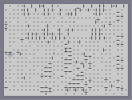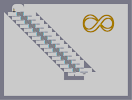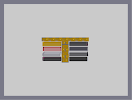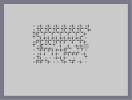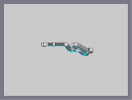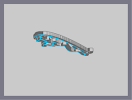Another Frustarating Maze Infinity Myspace Buttons Another, yet less frustrating, maze. Jag Test Jaguar

Pages: (0)

### Sparae Time?

Ya, I had a lot of spare time.
And now before thanksgiving, i have some more spare time.

The text?
The same way i do some of my other maps, but left an open space where the letters went.

### faved

how do you make the text O.o
lol
9999999/5

### EPIC 5/5

U must have a lot of spare time!

### yeah it's alright....

:)

seriously, my jaw dropped.

Shit....

### How do i do it?

This is how i do it...

### HOLY\$^%\$^^&#OF%^\$*&^\$&%^IN&^%*(&\$^&%^*FORTHELOVEOF^%&*^&((!!!!!

OOOOOO
MMMMMM
GGGGGG
HOW THE FUCK DO YOU DO YOU DO ALL OF THIS N-ART???!?

Awesome

### HOW THE FUCK DO YOU DO THIS?!?!?!?!?!?!

THIS IS INSANE!!!
holy \$h!t wow cra-z

in all :)
(5aved)

### Dude

you're awesome at n-art.
100000000000000000000000000000000000000000000000000000000000000000000000000000000000000000000000000000000000000000000000000000000000000000000000000000000000000000000000000000000000/5 stares in awe..............................o_O

### OM*G!!

THat is F*cking great!!

### your getting good

your starting to catch up to people like Dragon_Moon with your art

### My goodness...

I love it, just as good, if not better than your Jaguar. 5aved, and keep up the good work.

Are you going to make a series of car logos by any chance?
5/5
you are amazing at n-art!

super-fantabulistic!

4.5/5 upped

### Realy,

i don't think that this will get featured. Not as good as the other one.

However the next pic i am doing might.

It is going to be bigger. About as tall as the map screen.

### okay

now if THIS gets featured, I am... I think it will be inapropriate for all of us. I liked this one thinking that you did it in record time and it looks WAY better in full veiw, I would say to try to make the image bigger so you can work more on details and such. I hope this helps

~Wumbla

### Holy sh!p...

Now that's next O_o

### Ryzor...

i guess your right. oh well. :3

### Holy sh!t...

Now that's text O_o

### It is very precise.

and it's an original way of doing art (if you're not lying through your teeth). However, I dont see the style being very applicable/variable.
I like the Jaguar one more, but this one's pretty cool too. 4/5.

### If anyone

saw how it looked right when i posted for the first time, yes i did fix it.